# JP5214422B2 - Data storage system - Google Patents

## Info

Publication number
JP5214422B2
JP5214422B2 JP2008311551A JP2008311551A JP5214422B2 JP 5214422 B2 JP5214422 B2 JP 5214422B2 JP 2008311551 A JP2008311551 A JP 2008311551A JP 2008311551 A JP2008311551 A JP 2008311551A JP 5214422 B2 JP5214422 B2 JP 5214422B2
Authority
JP
Japan
Prior art keywords
bit
bits
code
information
number
Prior art date
Legal status (The legal status is an assumption and is not a legal conclusion. Google has not performed a legal analysis and makes no representation as to the accuracy of the status listed.)
Expired - Fee Related
Application number
JP2008311551A
Other languages
Japanese (ja)
Other versions
JP2009217922A (en
Inventor

Original Assignee

Priority date (The priority date is an assumption and is not a legal conclusion. Google has not performed a legal analysis and makes no representation as to the accuracy of the date listed.)
Filing date
Publication date
Priority to JP2008035269 priority Critical
Priority to JP2008035269 priority
Application filed by 株式会社東芝 filed Critical 株式会社東芝
Priority to JP2008311551A priority patent/JP5214422B2/en
Publication of JP2009217922A publication Critical patent/JP2009217922A/en
Application granted granted Critical
Publication of JP5214422B2 publication Critical patent/JP5214422B2/en
Application status is Expired - Fee Related legal-status Critical
Anticipated expiration legal-status Critical

## Images

•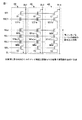•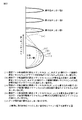•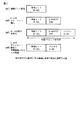•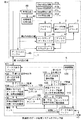•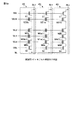•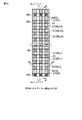•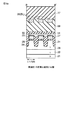••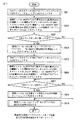•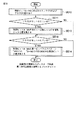•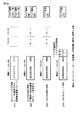•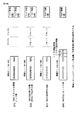••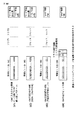•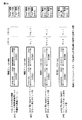•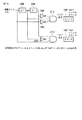••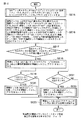•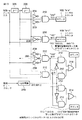•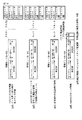•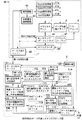•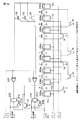•••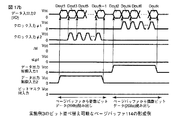•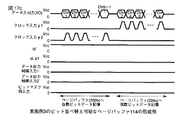•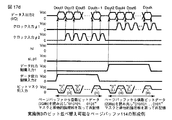•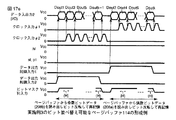••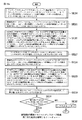•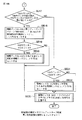•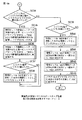•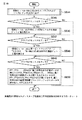••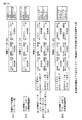•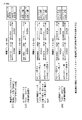•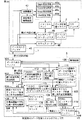•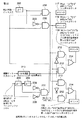•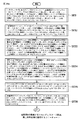•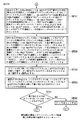••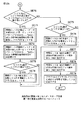••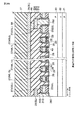•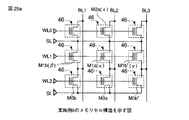•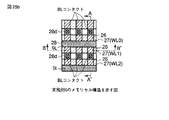•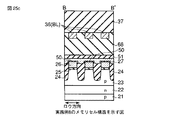•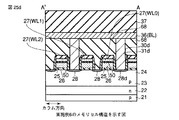•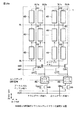•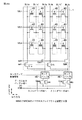•••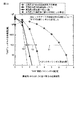••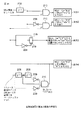•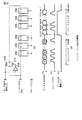•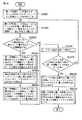•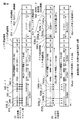•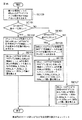•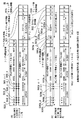•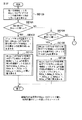•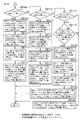•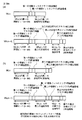•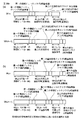•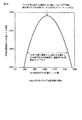## Classifications

• GPHYSICS
• G11INFORMATION STORAGE
• G11CSTATIC STORES
• G11C16/02Erasable programmable read-only memories electrically programmable
• G11C16/06Auxiliary circuits, e.g. for writing into memory
• G11C16/34Determination of programming status, e.g. threshold voltage, overprogramming or underprogramming, retention
• G11C16/3418Disturbance prevention or evaluation; Refreshing of disturbed memory data
• G11C16/3427Circuits or methods to prevent or reduce disturbance of the state of a memory cell when neighbouring cells are read or written
• GPHYSICS
• G06COMPUTING; CALCULATING; COUNTING
• G06FELECTRIC DIGITAL DATA PROCESSING
• G06F11/00Error detection; Error correction; Monitoring
• G06F11/07Responding to the occurrence of a fault, e.g. fault tolerance
• G06F11/08Error detection or correction by redundancy in data representation, e.g. by using checking codes
• G06F11/10Adding special bits or symbols to the coded information, e.g. parity check, casting out 9's or 11's
• G06F11/1008Adding special bits or symbols to the coded information, e.g. parity check, casting out 9's or 11's in individual solid state devices
• G06F11/1068Adding special bits or symbols to the coded information, e.g. parity check, casting out 9's or 11's in individual solid state devices in sector programmable memories, e.g. flash disk
• GPHYSICS
• G11INFORMATION STORAGE
• G11CSTATIC STORES
• G11C11/00Digital stores characterised by the use of particular electric or magnetic storage elements; Storage elements therefor
• G11C11/56Digital stores characterised by the use of particular electric or magnetic storage elements; Storage elements therefor using storage elements with more than two stable states represented by steps, e.g. of voltage, current, phase, frequency
• G11C11/5621Digital stores characterised by the use of particular electric or magnetic storage elements; Storage elements therefor using storage elements with more than two stable states represented by steps, e.g. of voltage, current, phase, frequency using charge storage in a floating gate
• G11C11/5628Programming or writing circuits; Data input circuits
• GPHYSICS
• G11INFORMATION STORAGE
• G11CSTATIC STORES
• G11C16/02Erasable programmable read-only memories electrically programmable
• G11C16/04Erasable programmable read-only memories electrically programmable using variable threshold transistors, e.g. FAMOS
• G11C16/0483Erasable programmable read-only memories electrically programmable using variable threshold transistors, e.g. FAMOS comprising cells having several storage transistors connected in series
• GPHYSICS
• G11INFORMATION STORAGE
• G11CSTATIC STORES
• G11C16/02Erasable programmable read-only memories electrically programmable
• G11C16/06Auxiliary circuits, e.g. for writing into memory
• G11C16/10Programming or data input circuits
• GPHYSICS
• G11INFORMATION STORAGE
• G11CSTATIC STORES
• G11C16/02Erasable programmable read-only memories electrically programmable
• G11C16/06Auxiliary circuits, e.g. for writing into memory
• G11C16/34Determination of programming status, e.g. threshold voltage, overprogramming or underprogramming, retention
• G11C16/3418Disturbance prevention or evaluation; Refreshing of disturbed memory data
• GPHYSICS
• G11INFORMATION STORAGE
• G11CSTATIC STORES
• G11C19/00Digital stores in which the information is moved stepwise, e.g. shift register stack stores, push-down stores
• GPHYSICS
• G11INFORMATION STORAGE
• G11CSTATIC STORES
• G11C29/00Checking stores for correct operation ; Subsequent repair; Testing stores during standby or offline operation
• GPHYSICS
• G11INFORMATION STORAGE
• G11CSTATIC STORES
• G11C7/00Arrangements for writing information into, or reading information out from, a digital store
• G11C7/10Input/output [I/O] data interface arrangements, e.g. I/O data control circuits, I/O data buffers
• G11C7/1006Data managing, e.g. manipulating data before writing or reading out, data bus switches or control circuits therefor
• GPHYSICS
• G11INFORMATION STORAGE
• G11CSTATIC STORES
• G11C8/00Arrangements for selecting an address in a digital store
• G11C8/04Arrangements for selecting an address in a digital store using a sequential addressing device, e.g. shift register, counter

## Description

The present invention relates to a data storage system and is applied to a highly reliable system that includes a semiconductor storage device and can be relieved even if a defective bit occurs, and is particularly reliable for margin reduction due to erroneous writing or capacity interference of adjacent cells. The present invention is applied to a semiconductor memory device or the like for improving the performance.

In a data storage system using semiconductor memory devices, for example, the charge injected by tunnel current from the channel through the insulating film to the charge storage layer is used as digital bit information storage, and the change in MOSFET conductance according to the amount of charge is measured. However, nonvolatile semiconductor memories (EEPROMs) that read information have been developed. Above all, NAND type EEPROM (NAND type flash memory) in which multiple memory cells are connected in series or in parallel to form a memory cell block can greatly reduce the number of select transistor gates compared to memory cells, resulting in higher density. can do. In addition, in the NAND type EEPROM, the well in which the memory cells are formed is made common so that erasing is performed collectively on a page composed of a plurality of memory cells, and writing is performed on each memory cell. Therefore, the density can be increased. Here, unlike the DRAM, the non-volatile memory can be read a plurality of times without data destruction.

However, if the capacitive coupling between adjacent cells increases due to further progress in miniaturization and densification, the threshold between the information bits changes as the threshold value changes depending on the amount of charge in the charge storage layer of the adjacent cells. The value voltage margin decreases. In particular, NAND flash memory has a problem that due to the data pattern at the time of writing, erroneous writing occurs in which a bit to be erased changes to a writing bit even when erased at once, and the threshold voltage margin further decreases. there were.

As one solution to alleviate such a problem of error bits, there is a method of using error correction using ECC (Error Correcting Code) as in Patent Document 1 disclosed by the present inventors. However, there is a problem that the erase threshold value or the write threshold value is increased due to capacitive coupling due to the adjacent memory cell being changed from the erase threshold value to the write threshold value, and more erroneous writing is caused by the adjacent memory cell pattern. There is no disclosure of an effective ECC configuration method that captures the characteristics of the problems that occur.

More specifically, when a BCH (Bose-Chaudhuri-Hocquenghem) code and an RS (Reed-Solomon) code are used as the ECC, for example, in the BCH code, the code length n is a natural number as a t-double error correction code. as is fixed at 2 m -1, corresponding information bit number k0 is limited to k0 = 2 m -1-mt. Further, in the RS code, as a t-heavy error correction code, 1 byte is m bits, the code length n is 2 m −1 (bytes), and the corresponding number of information bytes k1 is k1 = 2 m −1-2t. Limited. As described above, when a BCH (Bose-Chaudhuri-Hocquenghem) code or the like is used as the ECC, it is conventionally used for a method such as a shortened code, and the degree of freedom is small due to a restriction on the code length or the like.

As described above, the conventional BCH abbreviated code creation method and the RS abbreviated code creation method alone are not a correction method resistant to errors with respect to a specific continuous pattern of codes such as “010”, but random single bits, or It was an error correction method for a fixed number of random bytes.

Therefore, the above-mentioned erase threshold value and write threshold value may increase due to capacitive coupling due to the adjacent memory cell being changed from the erase threshold value to the write threshold value, and more erroneous writing may occur depending on the adjacent memory cell pattern. The problem that arises cannot be solved by capturing the characteristics of this problem. Therefore, conventionally, even when combined with a BCH code or an RS code, a more effective coding configuration method is not disclosed.

As described above, the conventional data storage system has a random single bit for randomization in which the erase threshold value and the write threshold value are changed by capacitive coupling and erroneous writing is caused by the pattern of adjacent memory cells, or The error correction for a fixed number of random bytes was performed. As a result, there has been a problem that, for example, a specific continuous pattern of codes such as “010” cannot capture errors more strongly and correct against errors.
JP-A-2005-243183

The present invention provides a data storage system that is more advantageous for error correction and captures more features with respect to a specific pattern of codes.

According to one aspect of the present invention, at least binary digital data of “1” and “0” is stored as charges in a charge storage layer, and a difference between two charge amounts in the charge storage layer is used as a write bit and an erase bit. A nonvolatile memory cell array that includes a memory cell to be used and erases a page including a plurality of memory cells adjacent to each other, and a code that corrects at least one bit of error data from an information bit is generated and recorded on the page An error correction code generation circuit that generates an error correction code to be corrected, an error correction code decoding circuit that corrects an error from the error correction code and restores the information bits from the digital data recorded on the page, and the input information bits and - taking the exclusive OR of the plurality of bits is repeated a plurality of times a sequence of "write bit erase bits", the error correction code generator It can provide data storage system comprising a code conversion circuit for outputting the road.

According to the present invention, it is possible to obtain a data storage system that is more advantageous for error correction and that captures more features with respect to a specific continuous pattern of codes.

[Comparative example]
First, in order to compare with the data storage system according to the first to seventh embodiments of the present invention described below, the data storage system according to the comparative example will be described with reference to FIGS. FIG. 1 shows a circuit configuration of a memory cell element of a NAND type EEPROM semiconductor device according to a comparative example.

As shown in FIG. 1, nonvolatile memory cells M0 to M31 made of MOS transistors having charge storage electrodes are connected in series, and one end is connected to a data transfer line denoted by BL via a select transistor S1. . The other end is connected to a common source line labeled SL through a selection transistor S2. Each transistor is formed on the same p-type well 23. The control electrodes of the memory cells M0 to M31 are connected to data selection lines (hereinafter referred to as WL) denoted WL0 to WL31. Further, in order to select one memory cell block from a plurality of memory cell blocks along the data transfer line and connect it to the data transfer line, the control electrode of the selection transistor S1 is connected to the block selection line SSL. Further, the control electrode of the selection transistor S2 is connected to the block selection line GSL, forming a so-called NAND type memory cell block 49 (dotted line region). Here, in this comparative example, the control wirings SSL and GSL of the selection gate are connected by cells adjacent to each other in the left-right direction on the paper surface by a conductor in the same layer as the charge storage layer 26 of the control wirings WL0 to WL31 of the memory cell element. Is formed. In this comparative example, a memory cell array structure connected to three data transfer lines (BL1, BL2, and BL3) will be described as an example in order to make the problem easy to understand without making the figure excessively complicated. However, of course, this parallel number is not three, and a structure in which a larger number, for example, 2100, 4200, 8400, 16800, and 33600 are connected in parallel is assumed.

Here, in order to explain the problem that the potential of the charge storage electrode changes due to capacitive coupling of adjacent memory cells, for example, the threshold distribution for the M1a (α) cell formed at the intersection of WL1 and BL2 For example, as shown in FIG. In a multi-value memory cell having a charge storage layer, negative charges are stored in adjacent memory cells, thereby causing a problem that the threshold value is increased through capacitive coupling between adjacent memory cells. To explain this problem, taking M1a (α) cell as an example, memory cells M1b (β) and M1b ′ (γ) adjacent in the BL direction, and adjacent to the S1 terminal side in the WL direction and connected in series FIG. 2 shows threshold fluctuations when the memory cell M2a (ε) changes from the erased state to the written state.

In FIG. 2, (i) shows that the adjacent memory cells (β, γ) on the adjacent data transfer line side and the adjacent memory cells (ε) on the S1 side word line side are all erased. The threshold distribution is shown. FIG. 2 shows a four-value write state. For example, assuming four-value storage, write threshold 3, write threshold 2, write threshold 1 from the higher threshold value side. Assume that the write threshold values are set in this order.

In (ii), adjacent memory cell 1 cell (γ) on the adjacent data transfer line side is in a write state, adjacent memory cell (β) on the opposite side, and adjacent memory cell on the word line side on S1 side ( An erasure threshold distribution of the memory cell α when ε) is in the erased state is shown. In this case, since one adjacent memory cell (γ) is in the written state, electrons are accumulated more than in the erased state. Therefore, as compared with (i), the threshold value of the memory cell α increases due to the capacitive coupling of adjacent memory cells. Furthermore, there is a problem in that the threshold value is raised and the upper tail distribution is generated compared to (i) due to so-called erroneous writing, which will be described in detail below. Here, in the NAND flash memory, the memory cells commonly connected to the data selection line apply the potential of WL as the data selection line, for example, a potential of about 15 V to about 30 V, and are electrically connected. The data transfer line (BL) potential is set to 0V, the source electrode and the drain electrode of the memory cell selected for writing are set to 0V, for example, and the channel potential is also set to 0V. On the other hand, a memory cell that is not selected for writing and is maintained in an erased state, that is, a source electrode, a drain electrode, and a channel potential of a so-called unselected memory cell are maintained at a data selection line, for example, between 5V and 10V. Writing is prevented by reducing the potential difference between the data transfer line and the data transfer line. Note that a non-selected memory cell is realized by raising the potential of the electrically connected BL from 0V. Further, in the NAND flash memory, in order to reduce the distribution width of the write threshold, by performing the verify read after the write, the memory cell that has reached the write lower limit threshold (write verify threshold) By selectively raising the potential of BL from 0V, additional writing is prevented from occurring.

Here, a case is considered in which a potential of about 15V to about 30V is applied to WL1 in order to keep the memory cell α connected to WL1 in the erased state and write another memory cell connected to WL1. In this case, at the time of writing in the state (ii), the source electrode, the drain electrode, and the channel potential of the memory cell γ to be written are 0V, and the source electrode, the drain electrode, and the channel potential of the memory cell α are from about 5V. It is kept at around 10V.

On the other hand, at the time of writing in the state (i), the source electrode, the drain electrode, and the channel potential of the memory cell γ and the memory cell α are maintained at about 5V to about 10V. Therefore, in the case of writing in the state (ii), a current flows from the source electrode or the drain electrode of the memory cell α to the source electrode or the drain electrode of the memory cell γ, whereby the source electrode or the drain electrode of the memory cell α. The potential decreases. Alternatively, when the potential of the source electrode and the drain electrode of the adjacent memory cell γ and the potential of the channel portion are lower in the case of (ii) than in (i), the channel portion of the memory cell α is caused by capacitive coupling in the BL direction. The voltage of also decreases in the case of (ii) than in (i). As a result, in (ii) as compared with (i), the voltage of the channel portion of the memory cell α is lowered, so that erroneous writing that causes writing to an unselected memory cell occurs, and the threshold value of the memory cell α An increase occurs. As described above, in the case of (ii) as compared with (i), there arises a problem that the threshold value of the erased memory cell α is further increased. In particular, in a memory cell that has undergone writing and erasing stress, a charge trap is formed in the tunnel insulating film, and stress induced leakage current (SILC) easily flows from the charge storage layer due to weak writing stress. In this case, compared to (i), the difference in the amount of charges stored in the charge storage layer is larger in the state (ii), and electric field stress is applied in the direction of the adjacent memory cell. Therefore, the upper skirt of the erasure distribution is likely to occur.

The problem that spreads when the above erase threshold is (ii) is that (ii) is that the adjacent memory cell 1 cell (β) on the adjacent data transfer line side is in the write state and the adjacent memory cell ( The same occurs in the erase threshold distribution of the memory cell α when the adjacent memory cell (ε) on the S1 side word line side is in the erased state.

Further, in (iii), when two adjacent memory cells (β, γ) are written in the adjacent data transfer line side and the adjacent memory cell (ε) on the S1 side word line side is in the erased state, An erasure threshold distribution is shown. In the case of (iii), since both of the memory cells (β, γ) adjacent in the BL direction are in the written state as compared with the case of (ii), more electrons are accumulated than in the erased state. . Therefore, compared with (ii), the threshold value of the α cell increases due to capacitive coupling of adjacent memory cells. Further, the threshold value is further increased compared to (ii) due to so-called erroneous write stress, and an upper skirt distribution is generated.

Here, a case is considered in which a potential of about 15V to about 30V is applied to WL1 in order to keep the memory cell α connected to WL1 in the erased state and write another memory cell connected to WL1. In this case, at the time of writing in the state (ii), the source electrode, the drain electrode, and the channel potential of the memory cells β and γ to be written are 0V, and the source electrode, the drain electrode, and the channel potential of the memory cell α are 5V. To 10V. On the other hand, at the time of writing in the state (ii), the source electrode, the drain electrode, and the channel potential of the memory cell β, the memory cell γ, and the memory cell α are maintained at about 5V to about 10V. Therefore, in the case of writing in the state (iii), a current flows from the source electrode or the drain electrode of the memory cell α to the source electrode or the drain electrode of the memory cell γ or the memory cell β, whereby the memory cell The potential of the source or drain electrode of α is lower than in the case of (ii). Alternatively, when the potential of the source electrode and the drain electrode of the adjacent memory cell β and the memory cell γ and the potential of the channel portion are lower in the case of (iii) than in (i), the memory cell is subjected to capacitive coupling in the BL direction. The voltage of the channel portion of α also decreases. As a result, in the case of (iii) compared to (i), the voltage of the channel portion of the memory cell α is lowered, so that erroneous writing that causes writing to a non-selected memory cell occurs, and the threshold value of the memory cell α An increase occurs. Further, even when compared with the case (ii), the potential of the channel portion of the memory cell adjacent on both sides in the BL direction is low, so that erroneous writing is more likely to occur than in the case (ii). From the above, in the case of (iii) compared to (ii), there arises a problem that the threshold value of the erased memory cell α is further increased.

Further, in (iv) of FIG. 2, the memory when two adjacent memory cells (β, γ) are in the write state on the adjacent data transfer line side and the adjacent memory cell (ε) on the word line side on the S1 side is in the write state. The erasure threshold distribution of the cell α is shown. In the case of (iv), compared to the case of (iii), the adjacent memory cell (ε) on the word line side on the S1 side is in the written state, so that electrons are accumulated more than in the erased state. Therefore, compared to (iii), the threshold value of the α cell is further increased than in the case of (iii) due to capacitive coupling of the adjacent memory cell (ε). The contribution of capacitive coupling of memory cells in the diagonal direction to WL and BL is as small as 40% or less and 1% or more compared to the contribution of capacitive coupling in other BL and WL directions. In this state (iv), the main pattern has a distribution spreading higher in the erase threshold than in the cases (i) to (iii).

In the multi-level NAND flash memory, conventionally, the same multi-level data is written sequentially from the cell on the SL side to the BL side. For this reason, the adjacent memory cell (ε) on the S1 side word line side is written with the same level of data after the memory cell (α). Cell (α) will receive. When the adjacent memory cell (β, γ, ε) is in the write state, the problem that the threshold distribution of the memory cell α spreads higher than when the adjacent memory cell is set to the erased state is This is a problem that the memory cell α also has a write threshold because of capacitive coupling between the memory cells. That is, in order to clarify the main problem of the present invention, the explanation and illustration are omitted, but the threshold value rise problem similarly in the case of the write threshold values 1, 2, and 3 in FIG. Occurs.

On the other hand, in FIG. 2 (v), for example, after performing a certain value of program erasure (generally referred to as Endurance) from 10 times to 100,000 times, for example, 85 degrees for 1 year to 10 years The distribution of the write threshold value 1 when the data is held as it is is shown. As for the write threshold, after Endurance, an electron trap is formed near the semiconductor substrate of the tunnel insulating film due to tunnel current or electric field stress. This electron trap has a feature that charges trapped in the tunnel insulating film are more likely to be released than charges accumulated in the charge storage region by leaving the electrons trapped for a long time after trapping electrons during writing. Therefore, as shown in (v), there arises a problem that the threshold value is lowered due to electron emission from the threshold value immediately after writing. Needless to say, after the endurance, electron or hole traps are formed in the tunnel insulating film due to tunnel current or electric field stress, which causes a problem of leakage current flowing through the trap level. When this problem occurs, the distribution tends to be tailed below the threshold as shown in the lower end of (v) of FIG. In any case, after charge retention, the write threshold value tends to decrease and the distribution tends to expand compared to the distribution immediately after writing.

Here, the write thresholds 1, 2, and 3 are assumed to be higher than the neutral threshold. This is a reasonable assumption in the conventional NAND type semiconductor memory device. In this case, if the write threshold value 3 is high, for example, the electric field of the tunnel insulating film at the time of charge retention increases, so that the leakage current through the tunnel insulating film increases and the data retention characteristics deteriorate. Also, if the write threshold value 3 is high, the electric field at the time of writing or holding the charge between the charge storage layer and the control electrode increases, resulting in an increase in leakage current and dielectric breakdown. Data retention characteristics and memory cell reliability deteriorate. Therefore, the write threshold value has an upper limit for maintaining the reliability of the memory cell, and is set in the range of about 4V to about 8V, for example. On the other hand, if the erase threshold is low, the electric field at the time of erasing the so-called interpoly insulating film between the charge storage layer and the control electrode increases, resulting in an increase in leakage current and dielectric breakdown. In addition, the reliability of the memory cell deteriorates. Therefore, the erase threshold has a lower limit for maintaining the reliability of the memory cell. For example, the lower limit is set in a range of about −4 V or less and −10 V or more. Since these are accompanied by deterioration of memory cell reliability and dielectric breakdown, the write threshold distribution and erase threshold of the memory cell fall within the upper limit of the write threshold and the lower limit of the erase threshold. It is necessary to set all distributions. As shown in FIG. 2, the distribution of the erase threshold value fluctuates to the higher side due to capacitive coupling between adjacent memory cells or erroneous writing, and the threshold value is lowered in the characteristics after the write threshold value is held. If the threshold distribution overlaps as shown in (iv) and (v), the write bit is erroneously detected and erroneously corrected as an erase bit, or the erase bit is erroneously detected and erroneously corrected as a write bit. Problems arise. As described above, the upper and lower limit range in which the threshold can be set is determined. To narrow the write threshold distribution, it is necessary to narrow the write program voltage step, and the trade-off with the decrease in write speed is a big problem It becomes. In addition, when the physical interval between adjacent memory cells is narrowed and the capacitive coupling between adjacent memory cells is further increased, the threshold values of the write threshold values 1 and 2 are also increased depending on the charge amount of the charge storage layer of the adjacent cells. There arises a problem that the threshold width increases. Therefore, in a high-density NAND memory cell in which miniaturization of the memory cell has progressed, it is difficult to suppress this error bit problem only by threshold distribution control.

In order to alleviate such a problem of error bits, error correction using ECC (Error Correcting Code) as disclosed in, for example, the above-mentioned Patent Document 1 (Japanese Patent Laid-Open No. 2005-243183). A method of using is known. However, there is a problem that the erase threshold value or the write threshold value is increased due to capacitive coupling due to the adjacent memory cell being changed from the erase threshold value to the write threshold value, and more erroneous writing is caused by the adjacent memory cell pattern. There is no disclosure of an ECC configuration method that captures characteristics and is effective for the problems that occur.

More specifically, when a BCH (Bose-Chaudhuri-Hocquenghem) code and an RS (Reed-Solomon) code are used as the ECC, for example, in the BCH code, the code length n is m as a double error correction code. Is fixed at 2 m −1, and the corresponding number of information bits k0 is limited to k0 = 2 m −1−mt. The RS code is a t-th error correction code, 1 byte is m bits, the code length n is 2 m −1 (bytes), and the corresponding number of information bytes k1 is k1 = 2 m −1-2t. Limited. When a BCH (Bose-Chaudhuri-Hocquenghem) code is used as the ECC, since the degree of freedom is smaller than the restriction of the code length, a method such as a shortened code has been used.

FIG. 3 (a) shows a shortened code method for the BCH code according to the comparative example.
First, as shown in (a1) of FIG. 3, k information bits fewer than the k0 bits are prepared. Next, l-bit “0” is added to (a2) to make it equal to the number of bits corresponding to the k0 bit described above. Further, the information sequence to which “0” bit is added is BCH-encoded to form an n-bit code word to which a parity to be a check bit is added as shown in (a3). Next, as indicated by (a4), the l-bit “0” in the encoded codeword is removed, and the (nl) -bit codeword is stored in the nonvolatile memory. In this way, a ((nl), k (= k0-l)) BCH shortened code is formed from the (n, k0) BCH code. Here, the information bits are separated from the check bits as information bits even after the BCH code. This feature is also present in the RS code.

However, only the BCH shortened code creation method and the RS shortened code creation method as described above are not correction methods that are resistant to errors with respect to a specific continuous pattern of codes such as “010”, but random single bits or random This is an error correction method for a certain number of bytes. Therefore, the above-described erase threshold value and write threshold value may increase due to capacitive coupling due to the adjacent memory cell changing from the erase threshold value to the write threshold value, and more erroneous writing may occur depending on the adjacent memory cell pattern. It is not a coding configuration method that is more effective when the characteristics of this problem are captured and combined with a BCH code or an RS code.

As described above, in the data storage system according to the comparative example, the random threshold is changed with respect to the randomization problem in which the erase threshold value and the write threshold value are changed by the capacitive coupling and the erroneous write is caused by the pattern of the adjacent memory cell. In this configuration, error correction is performed on a bit or a random fixed number of bytes. As a result, for example, it is disadvantageous in that a specific continuous pattern such as “010” cannot be subjected to error-proof correction that captures more features.

Hereinafter, some examples 1 to 7 which are considered to be the best will be described. In this description, common parts are denoted by common reference symbols throughout the drawings.

[Example 1]
Next, the data storage system and its error correction operation according to Embodiment 1 of the present invention will be described with reference to FIGS.

<1. Configuration example>
First, an example of the overall configuration of the data storage system according to the first embodiment will be described with reference to FIG. FIG. 4 is a block diagram layout of the data storage system according to the first embodiment.

As shown in the figure, the data storage system according to this example includes a storage device 7 and a memory controller 5.

A portion surrounded by a dotted line as the storage device 7 is formed on one semiconductor substrate as a NAND flash memory, for example. A portion surrounded by a dotted line as the memory controller 5 shows a circuit configuration corresponding to a so-called flash memory controller, and usually shows a circuit formed on a semiconductor substrate different from the storage device 7. Of course, they may be formed on the same semiconductor substrate. In this way, for example, the memory controller 5 and the storage device 7 are formed in the same package, board or card, thereby forming a data storage system.

The data input / output buffer 45 is connected to the data input / output buffer 101 of the flash memory controller 5 by a first internal I / O line.

Here, in all the embodiments of the present application, the memory cell is a memory cell that can be read a plurality of times without data destruction. Further, a specific configuration of the memory cell block 49 will be described below. Note that the logical value in the erased state is “1” and the logical value in the written state is “0” in accordance with the practice of flash memory. In addition, for simplicity of explanation, in all of the following embodiments, the threshold value of the binary memory cell is described unless otherwise specified, but of course, the application of the embodiment is also applied to the case of a multilevel memory cell. Is possible. In this case, when the threshold distributions representing the data holding bits are arranged in order from the higher threshold value to the lower threshold value of the memory cell, any two adjacent threshold distributions a, b (a The threshold distribution below a is read as “1”, and the threshold distribution above b is read as “0”. In NAND flash memory, erase memory cells connected to the same data control line can be erased at once, and NAND cell block 49 can also be erased at once, so the distribution of erase thresholds is 2V or more and 6V or less. Becomes wider than the distribution width of the write threshold value of 0.1V to 1V. For this reason, the threshold of the memory cell (α) when any threshold value of the adjacent memory cell (β, γ, ε) changes from the erase threshold value to the write threshold value in FIG. Since the value change influence becomes the largest, it is desirable to read as “1” for the logical value in the erased state and “0” for any other written state in the multi-value memory cell.

Next, a planar configuration example of the memory cell array will be described with reference to FIGS. 5A and 5B. 5a and 5b are an equivalent circuit and a plan view of the NAND cell block 49, respectively.

FIG. 5b shows a structure in which three cell blocks 49 of FIG. 5a are arranged in parallel. In particular, in FIG. 5b, only the structure below the control gate electrode 27 is shown for easy understanding of the cell structure. In FIG. 5a, nonvolatile memory cells M0 to M31 made of MOS transistors having charge storage electrodes 26 are connected in series, and one end is connected to a data transfer line denoted by BL through a selection transistor S1. The other end is connected to a common source line labeled SL through a selection transistor S2. Each transistor is formed on the same p-type well 23. The control electrodes of the memory cells M0 to M31 are connected to data selection lines denoted WL0 to WL31. Further, in order to select one memory cell block from a plurality of memory cell blocks along the data transfer line and connect it to the data transfer line, the control electrode of the selection transistor S1 is connected to the block selection line SSL. Further, the control electrode of the selection transistor S2 is connected to the block selection line GSL, forming a so-called NAND type memory cell block 49 (dotted line region). Here, in this embodiment, the control wirings SSL and GSL of the selection gate are connected by memory cells adjacent in the horizontal direction on the paper surface by a conductor in the same layer as the charge storage layer 26 of the control wirings WL0 to WL31 of the memory cell element. Is formed. Here, the memory cell block 49 only needs to have at least one SSL and GSL block selection line, and it is desirable that the memory cell block 49 be formed in the same direction as the data selection lines WL0 to WL31. In this embodiment, an example of the memory cell block 49 is 32 = 2 5 memory cells are connected, the number of memory cells connected to the data transfer lines and the data select lines may be plural, 2 n pieces or divisible, between the dummy memory cell S2 and WL0, 2 n +2 pieces plus between S1 and WL31 (n is a positive integer) on which it is to address decode a block of multi-level memory cells desirable.

Next, a cross-sectional configuration example of the memory cell array will be described with reference to FIGS. 6A and 6B. 6a and 6b are cross-sectional views in the direction of arrows BB 'and AA' in FIG. 5b. The BB ′ direction cross-sectional view corresponds to the memory cell portion cross-sectional view. 6A and 6B, for example, a silicon oxide film or oxynite having a thickness of 3 to 15 nm is formed in the p-type silicon region 23 having a boron impurity concentration of 10 14 cm −3 to 10 19 cm −3. through the oxynitride film 25, 25 SSL, 25 tunnel gate insulating film formed from a GSL, such as phosphorus or the charge storage layer 26, 26 SSL arsenic consisting 10 18 cm -3 from 10 21 cm -3 the added polysilicon , 26 GSL is formed with a thickness of 10 nm to 500 nm. These are formed in a self-aligned manner with the p-type silicon region 23 on a region where the element isolation insulating film 24 made of, for example, a silicon oxide film is not formed. This is because, for example, 25 and 26 are deposited over the entire surface of the semiconductor region 23, and then patterned and etched to a depth of, for example, 0.05 to 0.5 μm and embedded in the insulating film 24 until reaching the semiconductor region 23. Can be formed. As described above, since 25 and 26 can be formed on the entire surface without a step, it is possible to perform film formation with improved uniformity and uniform characteristics.

On this, for example, a silicon oxide film or an oxynitride film having a thickness of 5 nm to 30 nm, or a silicon oxide film / silicon nitride film / silicon oxide film, or an oxide film containing aluminum, hafnium, lanthanum, or zirconium element Polysilicon doped with 10 17 to 10 21 cm −3 of, for example, phosphorus, arsenic, or boron through block insulating films 50, 50 SSL , 50 GSL made of a laminated film of silicon oxide film and silicon nitride film Control gate 27 consisting of a stack structure of WSi (tungsten silicide) and polysilicon, or a stack structure of W and polysilicon, or a stack structure of NiSi, MoSi, TiSi, CoSi and polysilicon is 10 nm to 500 nm It is formed with the thickness of. The control gate 27 is formed up to the block boundary in the left-right direction in FIG. 5B so as to be connected by adjacent memory cell blocks in FIG. 5B, and forms data selection lines WL0 to WL31. Note that it is desirable that the p-type silicon region 23 can be applied with a voltage independently of the p-type semiconductor substrate 21 by the n-type silicon region 22 in order to reduce the booster circuit load during erasing and to reduce power consumption. . In the gate shape of this embodiment, the sidewall of the semiconductor region 23 is covered with the insulating film 24, so that it is not exposed by etching before forming the floating gate electrode 26, and the gate electrode 26 is larger than the semiconductor region 23. Can prevent you from coming down. Therefore, the gate field concentration at the boundary between the semiconductor region 23 and the insulating film 24 and a parasitic transistor with a lowered threshold voltage are unlikely to occur. Further, since a phenomenon of lowering a write threshold value due to electric field concentration, a so-called sidewalk phenomenon is less likely to occur, a more reliable transistor can be formed.

As shown in FIG. 6b, on both sides of these gate electrodes, for example, an n-type diffusion layer 28 serving as a source or drain electrode with a sidewall insulating film 43 formed of a silicon nitride film or a silicon oxide film having a thickness of 5 nm to 200 nm interposed therebetween. Is formed. The diffusion layer 28, the charge storage layer 26, and the control gate 27 form a floating gate type EEPROM cell that uses the amount of charge stored in the charge storage layer 26 as an information amount, and the gate length is 0.5um. Below 0.01um or more. As these source / drain n-type diffusion layers 28, for example, phosphorus, arsenic, and antimony are formed at a depth of 10 nm to 500 nm so that the surface concentration is 10 17 cm −3 to 10 21 cm −3 . Further, these n-type diffusion layers 28 are shared between adjacent memory cells, and NAND connection is realized. In the figure, 26 SSL and 26 GSL are gate electrodes connected to block selection lines corresponding to SSL and GSL, respectively, and are formed in the same layer as the floating gate electrode of the floating gate type EEPROM. . The gate length of the gate electrode 26 SSL and 26 GSL is longer than the gate length of the memory cell gate electrode.For example, by forming the gate length to be 1 um or less and 0.02 um or more, a large ON / OFF ratio can be secured when the block is selected and when it is not selected. Incorrect writing and reading can be prevented.

Further, 27 n-type diffusion layer 28 d to be the source or drain electrode formed on one side of the SSL, for example, tungsten, tungsten silicide, titanium, titanium nitride, copper or the data transfer line 36 made of aluminum, (BL) And a contact 31d . Here, the data transfer line 36 (BL) is formed up to the block boundary in the vertical direction of the drawing in FIG. 5B so as to be connected by adjacent memory cell blocks. On the other hand, the n-type diffusion layer 28 S that is the source or drain electrode formed on one side of 27 (GSL) is connected to the SL that becomes the source line via the contact contact 31 s . The source line SL is formed up to the block boundary in the left-right direction in FIG. 5B so as to be connected by adjacent memory cell blocks. Of course, the source line may be formed by forming the n-type diffusion layer 28 S to the block boundary in the left-right direction of the drawing. These BL contact and SL contact are filled with, for example, polysilicon doped with n-type or p-type, tungsten, tungsten silicide, Al, TiN, Ti, or the like to form a conductor region. Further, the space between the SL and BL and the transistor is filled with an interlayer film 68 made of, for example, SiO 2 or SiN. Further, an insulating film protective layer 37 made of, for example, SiO 2 , SiN, or polyimide, or an upper wiring made of, for example, W, Al, or Cu is formed on the BL, although not shown in the drawing. .

As shown in FIG. 5a, the cell array 1 is configured by arranging memory cell blocks 49 in which nonvolatile memory cells are connected in series or in parallel. A sense amplifier circuit 46 is provided via a data transfer line selection transistor in order to sense data on the data transfer line of the memory cell array 1 or hold write data. This sense amplifier circuit also serves as a data register, and is composed mainly of a flip-flop circuit, for example. Further, the sense amplifier circuit 46 is connected to the data input / output buffer 45. These connections are controlled by the output of the column decoder 48 that receives an address signal from the address buffer 47, the data applied to the data input / output buffer is written to the memory cell array 1, and the data is sent to the first internal I / O in FIG. Reading to the O line is possible. For the memory cell array 1, a row decoder 3 is provided to select a memory cell, specifically to control the data control lines WL0 to WL31 and the block selection gates SSL and GSL.

Also, in all embodiments of the present application, the number of data bits read in a series of sequences is the number of data bits read in a series of sequences, where m is a natural number and t is a natural number, [2 m-1 -t × (m-1) -1] greater than (2 m -t × m-1 ) is less than or equal to at least the (2 m -1) or more memory cells 49 FIGS. 5 (a) or FIG. 5 (b) Are prepared in parallel in the horizontal direction of the paper, that is, in the direction in which the data selection line extends, and used as one page. Further, in order to obtain an effect by adding 2 to 6 additional bits to the information bits due to the feature of this embodiment, m is at least 4 or more, and this embodiment suppresses an increase in array area. In general, m is 8 or more and 20 or less in the NAND flash memory. Note that the memory cells belonging to one page may be, for example, a plurality of memory cells commonly connected to one data selection line, and a plurality of memory cells may be simultaneously selected by one data selection. This is desirable. By preparing a memory cell in this way, for example, a bit error of t bits read out in a series of sequences of the cell array 1 using a Bose-Chaudhuri-Hocquenghem (BCH) code is detected and its position is obtained. Can do. Even in the case of the RS code, the data in one byte is read out simultaneously, so as described above, the memory cells 49 are prepared in parallel in the horizontal direction in FIG. 5a or 5b, that is, in the direction in which the data selection line extends, Used as one page.

Further, the input / output of the sense amplifier 46 is connected to a control logic 40 that controls reading, writing, and erasing of the memory cell. The control circuit 40 uses, for example, data given to the data buffer as a command input, and controls reading, writing, and erasing of the memory cell. Although not shown in the figure for simplification, the control circuit 40 is connected with a control signal for controlling the operation of the sense amplifier and the sel1 and sel2 control signals. Note that a portion surrounded by a dotted line 7 indicates a storage device conventionally formed on a single semiconductor substrate as a NAND flash memory, for example. Here, as for each of the above-described components, for example, a known circuit block may be used according to Japanese Patent Laid-Open No. 2002-150783, and thus detailed description thereof is omitted.

In the following, for the sake of clarity, the data string before encoding will be referred to as an information bit, and the bit added after encoding will be referred to as a check bit. Both the BCH code and the RS code form a code by adding a check bit to the original information bit. As shown in the prior art, the check bit is usually added after the information bit in order to simplify the circuit configuration of the code and decoding.

Here, a portion surrounded by a dotted line 5 in FIG. 4 shows a circuit corresponding to a so-called flash memory controller, and usually shows a circuit formed on a semiconductor substrate different from the storage device 7. Of course, they may be formed on the same semiconductor substrate. For example, data storage system is formed by forming 5 and 7 in the same package or board or card. The data input / output buffer 45 is connected to the data input / output buffer 101 of the flash memory controller 5 by a first internal I / O line.

On the other hand, an external input / output (I / O) is connected to another data input buffer 111, and inputs a flash memory write / erase / read address and data control information. Furthermore, the controller control circuit 1 20 and the flash memory controller 5, which performs control of input and output of data input and output of two data input-output buffer 101, 111. From the data input / output buffer 111, the information bits input through the external I / O are input to the code appearance probability averaging first encoding circuit 106. Here, the number of information bits input to 106 is assumed to be k0 bits. The code appearance probability averaging first encoding circuit 106 is, for example, for distributing the probability that the input data “0” and “1” appear almost evenly, and may be omitted. If present, it is a desirable circuit block. As the 106, for example, or period code generating circuit, in particular, M-sequence having a period shorter than k bits a1 bit (Maxi m um length code) may be used generating circuit for averaging bit mask generation circuit 110. The generated M-sequence code has a feature that “0” and “1” bits are distributed in the M1 period and are generated with a probability that “0” and “1” are equal in one period. Therefore, the information bits are divided in order for each a1 bit, exclusive ORed with each bit of a1 of the generated M sequence, output to 104 circuits, and, for example, an appropriate increment for each WL or each block of a1 To change the cycle start position. Alternatively, instead of the cycle start position, a second M-sequence code having a length of a2 bits is generated, and further, information bits are sequentially separated for each a1 bit, and exclusive OR is performed with each bit of a1 of the generated M-sequence. Then, the information bits are divided in order for each a2 bit and exclusive ORed with each bit of a2 of the generated second M-sequence. As a specific example, since the primitive polynomial of the 2-bit linear feedback shift register (LFSR) is x + 1, the exclusive OR of the second bit (x) and the first bit (1) is calculated. By setting the first bit of the next code and shifting the second bit from the first bit, 2 2 −1 = 3 states of “10”, “01”, and “11” can be obtained. Since 4 states with “00” that is 0 vector added to this are M-sequences, the information bits are separated in order every 2 bits, and “10 01 11 00 10 01 11 00. What is necessary is just to take a logical sum.

By doing this, the first encoded information bits in which all “0” and all “1” input information bits and “0” and “1” are appropriately distributed between WLs are transmitted to 104. The As a result, even when information data is randomly given from the external I / O, the information bits are input to 104 with the probability P = 0.5 which is equal to “0” and “1”, and the memory cells adjacent to each other are made uniform. can do. Of course, the averaged bit mask generation circuit 110 can share the same M series circuit or the second M series circuit in the decoding circuit 107, thereby generating each of the M series a1 and a2 generated in the same manner as the decoding circuit. By taking an exclusive OR with the bit, it can be correctly decoded.

Further, the information bits averaged by “0” and “1” input at 104 are converted into information bits and flags in which the disturbance by the adjacent memory cells is reduced by the adjacent memory cell disturb reduction second code conversion circuit 104. Is added to the error correction code generation circuit 102. Here, for example, a generally known BCH code generation circuit or RS code generation circuit may be used as 102. For example, the present invention can be realized by using the BCH encoding circuit disclosed in Japanese Unexamined Patent Application Publication No. 2005-243183, and is omitted here. As a result, the error correction code output from 102 is stored in the nonvolatile semiconductor memory element 7 through the data buffer 101.

Since the present invention performs encoding to reduce the capacity interference between physically adjacent memory cells or the erroneous writing problem, adjacent time-series information bits given to the nonvolatile semiconductor memory device 7 are It is assumed that data is written in memory cells that are physically adjacent except for well boundaries and source shunt regions. It goes without saying that such encoding is possible if appropriate physical-logical mapping is performed.

On the other hand, a code string that may contain an error read from the non-volatile memory 7 is input to a circuit 103 that decodes an error correction code through the data buffer 101, and is subjected to code error correction and disturbed by adjacent memory cells. A reduced information bit and a bit string to which a flag is added. For this 103, a generally known BCH code decoding circuit or RS code decoding circuit may be used. The BCH decoding circuit, for example, here, because the present inventors can be achieved by using the BCH encoding circuit disclosed in JP-2005-2 4 3183 will be omitted. Similarly, since the RS code is a kind of multi-element, 2 m- element (m is an integer) BCH code, it is known and obvious that the decoding circuit can be formed similarly.

Next, the information bit in which the disturbance by the adjacent memory cell is reduced and the bit string to which the flag is added are input to the adjacent memory cell disturb reduction second code inverse conversion circuit 105, and the second code decoding circuit 105 converts the first code. Decoded into applied information bits. Further, by inputting the information bit subjected to the first code to the first code decoding circuit 107, the original information bit is decoded. The information bit is output from the data input / output buffer 111 through an external I / O.

Note that, as described above, the first code decoding circuit 107 performs the exclusive OR with the same M sequence generated in 110 and used in the 106 circuit, thereby performing encoding and decoding. It is clear that the original information bits can be restored by taking the M-sequence and exclusive OR twice.

FIG. 9 shows, as an example, the input of 104 circuits in various information bit patterns, the state of an intermediate sequence, and an output example. "...." position indicated by shall be repeated pattern of 4 bits to the right periodically. In general, when the input information bits are k bits, the appearance probability is averaged in 106 circuits, but it is necessary to consider all states in which all information bits can be arbitrarily changed to “0” and “1”. There is. That is, as long as it can be encoded at 106 and decoded at 107, there are 2 k states for k information bits, and all 2 k × 2 k combinations between two adjacent word lines, The appearance probability is almost averaged in all states by 106 circuits. In all the following embodiments, the error correction code generation circuit 102, the adjacent memory cell disturbance reduction second code conversion circuit 104, the code appearance probability averaging first encoding circuit 106, and the error correction code decoding circuit 103, Adjacent memory cell disturb reduction second code inverse transformation circuit 105, first code decoding circuit 107, averaged bit mask generation circuit 110, “010” after exclusive OR of “01010101... 0101” Counter 112, “010” counter 113, “010” symbol counter 108, “101” symbol counter 109, counter 116, counter 117, counter 118 after taking the exclusive OR of “10101010... 1010” The counter 119, the page buffer 114 for rearranging the information bits, and the storage page buffer 115 for storing data on one source line side may be implemented by a hardware circuit. It is realized by Towea or firmware or a combination thereof of course good. In all the following embodiments, for the sake of simplicity, the case where the number of information bits k bits is even, for example, divisible by 2, or divisible by 4, is shown. If the number of bits is not divisible, for example, a method of enlarging the information bits by adding “1” until it becomes a multiple of 4 and coding so as to be divisible can be used. For example, when the information bit is “10101” of 5 bits, if it is “10101111 ” of 8 bits, it is clear that the code can be divided by either 2 or 4. As a code to be added, “1” is preferable to “0” in order to prevent a new generation of a “010” pattern due to the additional code.

<2. Error correction code / decoding operation>
Next, an error correction encoding / decoding operation of the data storage system according to the first embodiment will be described with reference to FIGS. FIG. 7 shows a specific sequence 104. In this description, description will be made in accordance with the sequence of FIG. 7 or FIG.

In the second code conversion circuit 104, first, in SE1, a “010” symbol counter 108 that counts the number of occurrences of “010” consecutive symbols of “010” in information bits, and “101” of 3 bits consecutive in information bits. The “101” symbol counter 109 that counts the number of generated symbols is reset, and flags 1 and 2 representing the coding state are reset. Hereinafter, for example, an “abc” symbol represents three consecutive bits indicated by “abc” in an information bit or code.

Next, in SE2, the number of consecutive bits “010” in k information bits is counted and stored in the “010” symbol counter 108. Further, the number of consecutive bits “101” in k information bits is counted and stored in the “101” symbol counter 109.

For example, as shown in FIG. 10, the “010” detection circuit can be easily input from the beginning of the information bit to the shift register using a clock synchronized with the information bit, combined with the “010” detection circuit, and counted by the counter. Can be realized. In FIG. 10, Z- 1 in 208 indicates a 1-bit 1-unit time delay element, 209 indicates an inverter, and 210 indicates a 3-input AND gate. In addition, as information bits, a series of information bits are sequentially input in time series from information bit input. In the unit time in which “010” is input in order, the “010” output is changed from “L” to “H” in the unit time in which “010” is input at the last “0”. In other cases, “L” It becomes. Therefore, this “010” output is synchronized with the unit time of the information bit input. For example, by triggering and counting when the logical value of the “010” output is stable in the unit time, the information bit “010” is output. The number can be counted. The count of “101” can be performed in the same manner. For example, the counter of FIG. 10 may be configured by a generally known binary counter, and therefore details of the counter are omitted here. As the 1-bit 1-unit time delay element represented by Z- 1 in 208 in this example, for example, a D-type flip-flop or a latch circuit is used, and in particular, the clock input is “L” (here, for convenience). The output is fixed at the moment when it rises from "0 [V]" to "H" (here, Vcc for convenience), and after that the clock remains "H" or "L". This can be easily realized by using an edge trigger type flip-flop in which the output does not change even if it changes from “L” to “L”.

Next, in SE3, it is determined whether the value of the “010” counter 108 is larger than the first threshold value. If the value is larger than the first threshold value, the information bit and the first bit mask pattern “01010101 .... 0101” (“01” repeated for k bits) are excluded in SE4. A sequence for performing logical OR is performed, information bits are replaced, and flag 1 is set. As this first bit mask pattern, “10101010... 1010” in which “10” is repeatedly generated for k bits may be used. Further, the first threshold value is considered to be the half of (k / 2) -1 which is the largest number of “010” in FIG. 9 (a1) and FIG. 9 (c1) in the information bits, and k Considering the case where the number is not divisible by 2 or 4, {(k / 4) rounded up to an integer} -1 makes the upper limit of the number of occurrences of the code “010” constant. Desirable.

In addition, when SE4 is implemented, in SE5, “010” symbol counter 108 that counts the number of occurrences of “010” 3-bit consecutive symbols in the information bits and “101” 3-bit continuous in the information bits The “101” symbol counter 109 that counts the number of generated symbols is reset, and flags 1 and 2 representing the coding state are reset. Further, at SE 6, the number of consecutive bits “010” in k information bits is counted and stored in the “010” symbol counter 108. Further, the number of consecutive bits “101” in k information bits is counted and stored in the “101” symbol counter 108.

Next, after joining the branch determined to be No in SE3, it is determined in SE7 whether the value of the “010” symbol counter 108 and the value of the “101” symbol counter 109 are larger. Here, if the value of the “010” symbol counter 108 is larger than the value of the “101” symbol counter 109, all the k-bit information bits are inverted in SE8, that is, the “0” bit is changed to the “1” bit. , Change “1” bit to “0” bit and set flag 2. Next, after joining the branch determined to be No in SE7, a code corresponding to flag 1 and flag 2 is added to the information bit in SE9.

(Decoding circuit 105 sequence)
Here, FIG. 8 shows a specific sequence of the decoding circuit 105 of the second code. In 105, first, in SE10, information corresponding to flags 1 and 2 added to the information bits is stored in, for example, a register or a temporary memory. Then, after determining whether flag 2 is set in SE11, if flag 2 is set, all information bits of k bits are inverted, that is, "0" bit is set to "1" in SE12. Bit, change “1” bit to “0” bit and replace with information bit. Next, after joining the branch determined to be No in SE11, it is determined in SE13 whether the flag 1 is set. If SE13 is Yes, that is, flag 1 is set, the exclusive OR of the information bits and the first bit mask pattern “01010101 .... 0101” (“01” repeated for k bits) A sequence is taken to replace the information bits. This first bit mask pattern is the same as “1010101010 .... 1010” when “10101010 .... 1010” is generated by repeatedly generating “10” for k bits when encoding 104. A mask bit may be used.

Here, as shown in (iii) and (iv) of FIG. 2 of the comparative example, the pattern in which the problem that the erase threshold value increases due to the threshold voltage rise of the adjacent memory cell is the memory cell (α) Is in the erased state, and the cells (β, γ) adjacent in the BL direction are in the written state, so that the bit string corresponds to “010”. Here, as shown in FIGS. 9 (a1) to (a4), in the configuration of the comparative example without 104 sequences, in the k-bit sequence “01010101 .... 0101”, “010” is [(k / 2) -1] is included, and the problem that the erase threshold value increases due to the threshold voltage increase of the adjacent memory cell occurs with a large probability. For example, one memory cell α is overlapped with the write threshold value (v) in FIG. 2 or the threshold for determining the read level as the write threshold is exceeded, and the erase bit is erroneously recognized as the write bit. Assuming that the failure probability is p0, it is assumed that p0 is sufficiently smaller than 1, and a failure of [(k / 2) -1] × p0 occurs. FIG. 9 (a1) and FIG. 9 (c1) are the worst patterns in which “010” occurs most in the comparative example. On the other hand, by using the sequence 104 of this embodiment, the number of “010” is [(k / 2) −1] in the data pattern input to 104 as shown in FIG. 9 (a1). By performing the sequence of SE3, the number of “010” before SE7 can be suppressed to {-1 (rounded up to (k / 4))} − 1 or less. Because, when branching to SE3 and proceeding to SE4, the number of “010” is more than {(value obtained by rounding up (k / 4) to an integer} -1), worst case, when “010” is arranged most densely However, “01010101 .... 01010” is occupied by (k / 2) +1 or more consecutive bits. Therefore, the number of bits other than “010”, particularly “000”, is less than (k / 2) bits at most. Therefore, when the information bits are exclusive-ORed with the mask of “01010101 .... 0101”, the “000” bits are inverted to the continuous bits of “010” and “010” is increased at most {( Value obtained by rounding up k / 4) to an integer} -1 or less.

On the other hand, if “010” is exclusive ORed with the mask “01010101 .... 0101”, either “010” or “101” will be selected depending on the start position of the “010” bit. This pattern is exclusive-ORed with that pattern. Since these are converted to “000” and “111”, respectively, the pattern of “010”, which is a problem, is reduced to {-1 (rounded up to (k / 4))}-1 or less as a whole. It will be. Of course, using “01010101... 0101” in which “01” is repeated as a mask with information bits is most effective in reducing the pattern of information bits “010”. However, for example, in the well contact or source shunt contact portion, dummy memory cells are adjacent to each other in the bit line direction, for example, 1 to 16 are formed between the memory cell for storing information and the well contact or source shunt contact portion. May be. For example, the present inventor has described the configuration of the dummy cell portion in detail in Japanese Patent Application Laid-Open No. 2007-221084, and is omitted here. In this case, since the memory cell is not written or erased in accordance with the information data in the dummy memory cell, the end of the cell column storing information adjacent to the dummy memory cell may be “0” or “1”. It has a feature that the problem of threshold change due to the adjacent memory cell described above hardly occurs. Therefore, for example, “01010101 .... 0101”, which is a repetition of “01”, or “10101010 .... 1010, which is a repetition of“ 10 ”, as appropriate for each memory cell array partition such as a well contact or a source shunt contact. Of course, it may be used by switching either of them, and the information bits are divided into substrings for each array delimiter of the memory cell, and the number of “010” and “101” is counted for each substring. Of course, the flags 1 and 2 shown at 104 corresponding to the respective subsequences may be added to reduce the probability that “010” appears. This may be applied to any embodiment described later. As shown above, when the number of “010” is greater than the threshold 1, the information bit is obtained by taking an exclusive OR with “01010101 .... 0101” or “10101010 .... 1010” The number of “010” in the worst is about half of the comparative example [(k / 2) -1], which can be reduced to less than {(k / 4) rounded up to an integer} -1 or less. This is the first unique feature found by the inventors. From the above, according to the sequence of FIG. 7, the number of “010” in the information bits is {(k / 4) −1] rounded up from the worst value [(k / 2) −1] of the comparative example} − It can be suppressed to 1 or less. Of course, since exclusive OR is performed with the bit mask “01010101... 0101”, the original information can be restored by performing exclusive OR with the same bit mask after encoding. Furthermore, if the number of error correction symbols is t, the amount of information processing that is proportional to approximately the square of t or more increases in decoding of BCH codes and RS codes, which causes a problem of speeding up decoding. On the other hand, in the second encoding circuit 104 and the second encoding / decoding circuit 105, the amount of information processing that increases only to the first power of the information bit length even if the information bit becomes longer in encoding and decoding at most. However, when t is 3 or more, the amount of information processing is much smaller than that of decoding, which is almost the same as the information processing amount of LFSR shift required for encoding of BCH code and RS code. Therefore, for example, even when the code length is longer than 1000 bits, the conversion to the second code and the reverse conversion can be performed in a sufficiently short time.

FIGS. 9 (a1), (b1), (c1), (d1), and (e1) show examples of input of various information bits, the number of “010” and the number of “101” at the right end. Next, FIGS. 9 (a2), (b2), (c2), and (d2) show the state after SE3 in 104. FIG. In FIG. 9 (a) and FIG. 9 (c), the number of “010” is larger than the threshold 1 {value obtained by rounding up (k / 4) to an integer} −1, the branch of SE3 becomes Yes, and SE4 Then, flag 1 is set, and in FIG. 9 (a) and FIG. 9 (c), the number of "010" in SE3 is equal to or less than threshold value 1, and the branch of SE3 is No. Next, in FIG. 9 (a3), (b3), (c3), (d3), the state before SE9 is shown at 104.

Here, as shown in FIGS. 2 (iii) and (iv) of the comparative example, the pattern in which the problem that the erase threshold value increases due to the threshold voltage rise of the adjacent memory cell is the memory cell (α) Since the cells (β, γ) adjacent in the erased state and the BL direction are in the written state, the bit string corresponds to “010”, and the bit-inverted “101” does not cause the problem of the comparative example. The bit string that becomes “010” in question is a bit-inverted version of “101”. By comparing the number of “010” with the number of “101” in SE7, The number of occurrences of “010” can be effectively reduced only by replacement. Here, an example in which the flag 2 is set in SE8 is shown in FIGS. 9 (d1) to (d4). As shown in FIG. 9 (d1), in the information bit pattern of repeating “0100”, there are (k / 4) number of “010” and 0 number of “101”. Therefore, after branching to the No side at SE3, at SE7, it is determined whether 108 counters counting the number of “010” are more than 109 counters counting the number of “101”. Proceed to the branch. Next, by performing all bit inversion in SE8, the number of “010” is 0 and the number of “101” is (k / 4), so the number of “010” can be reduced after all. it can. Of course, in SE7, it is determined whether 108 counters counting "010" are more than 109 counters counting "101". If No, bit inversion is not performed. Therefore, in this case, the number of “010” does not increase.

As described above, by using the sequence of SE7 and SE8 of 104 of the first embodiment, {the value obtained by rounding up the number of “010” in the information bits before SE7 {round up (k / 4) to an integer} — Since it is kept to 1 or less, the number of "010" before SE8 is {value obtained by rounding up (k / 4) to an integer} -1 or less, and more effective information bits that produce "010" are effective. Therefore, it can be converted into a bit in which “010” does not occur.

Because, in the sequence from SE3 to SE6, each information bit is only exclusive-ORed with the mask pattern, so the probability that “0” of each information bit is already output and “1” is already in 106 circuits. When the probability of exiting is approximately equalized, the probability that “0” is output for each information bit input to 104 is equal to the probability of “1” being output. Therefore, when considering all combinations of 2 to the power of 2 of information bits at the branch of SE7, the probability that the counter 108 is larger than the counter 109 is the same as the probability that the counter 108 is smaller than the counter 109. Therefore, by performing SE7 and SE8, the probability that “010” is generated in the remaining half of the information bit string in which the counter 108 matches the counter 109, for example, “01010111111111111 .... 1111” is excluded. And information bits in which fewer “010” are generated can be effectively realized.

As a method of adding the flag 1 and the flag 2 to the information bit, a method of adding each flag to the information bit as 2-bit continuous data is shown. This method is selected so that the state of “010” does not occur in any state of the flags 1 and 2, but of course, other encodings, for example, the same 2 bits are assigned to the flag 1 and the flag 2 is set to 1 A method of assigning bits may be used. In FIG. 9, the bits corresponding to flags 1 and 2 are aabb (a and b are “0” and “1” corresponding to flags 1 and 2, respectively), but bbaa and aab A bit whose order is changed, such as or bba, may be added as a bit corresponding to the flag. Of course, the bit corresponding to this flag is not added before or after the information bit, but, for example, the information bit may be divided into two and embedded in the information bit like bbaa. Of course, the information bits may be divided into three, and bb and aa may be embedded between the three information bits.

Also, after error correction coding, the check bits (parity) bits are divided into several pieces and rearranged (interleaved) so that two or more identical bits such as aa and bb are sandwiched between check bits. May be. In this way, since the bit contacting the flag composed of two or more same bits cannot be a “010” pattern, the possibility of the occurrence of the “010” pattern in the check bit portion can be further reduced.

FIGS. 9 (e1) to (e4) show examples of worst patterns in which “010” occurs in this embodiment. As shown in Fig. 9 (e1), if (k / 2) bits are repeated "10" bits and (k / 2) bits are repeated "00", the number of "010" patterns Is (k / 4) -1 and the number of "101" patterns is also (k / 4) -1. Therefore, in this case, in the sequence of FIG. 7, the flag 1 is reset and the flag 2 is also reset, but SE4, SE8 and the case where both the SE4 and SE8 sequences are temporarily performed are shown in FIG. 9 (e2), (e3) and (e4). In this case, the number of “010” patterns is either (k / 4) or (k / 4) -1 and the number of “101” patterns is also (k / 4) or (k / 4) -1 Therefore, even if SE4 or SE8 is performed, the selected number of “010” patterns is (k / 4) -1, which is one example of the worst pattern of the k-bit information bits “010” pattern. Become. This worst pattern can be further improved by the method described in the third embodiment.

The first embodiment has the advantage that it is not necessary to add counters 112 and 113, and the circuit scale can be further reduced and the circuit can be configured with a small area, compared to the second and third embodiments described later.

<3. Effects according to this embodiment>
According to the data storage system of this embodiment, at least the following effects (1) to (2) can be obtained.

(1) For example, it is advantageous for error correction that captures more features with respect to a specific continuous pattern of codes such as “010”.

As described above, in this embodiment, the worst state number in which “010” appears effectively can be obtained by adding at most 3 to 4 bits and at most 2 bits as information to k information bits. [(k / 2) -1] to {(k / 4) rounded up to an integer)}-1 or less, that is, about half or less.

For example, as indicated by an arrow Y1 in FIG. 29, when the number of “010” symbols is about 100, the worst state number in which “010” appears can be suppressed to less than half compared to the comparative example. .

Further, the number of occurrences of “010” in the information bits of the output of the second code conversion circuit 104 is always equal to or less than the number of occurrences of “010” in the information bits entering the second code conversion circuit 104. it can. Therefore, no matter what state the k-bit information bit is in 2 k , it is possible to suppress an increase in threshold value due to erroneous writing or capacitive coupling of adjacent memory cells in the BL direction with the worst pattern. Since the upper limit of the relative increase amount of the code length with respect to the addition of flag bits to the information bit length k is suppressed to 4 / k, this method is used when the information bits are sufficiently long, for example, 4 bits or more. In particular, the effect of suppressing the number of worst states in which “010” appears is great, and even if the number of information bits k increases, the encoding and decoding increase only to an extent that is proportional to k bits at most. Can be encoded and decoded. Therefore, an increase in the write threshold due to capacitive coupling of adjacent memory cells can be prevented, the electric field of the tunnel insulating film during charge retention is reduced, leakage current through the tunnel insulating film is suppressed, and data retention characteristics are deteriorated. The problem of mitigating the problem can be prevented. In addition, since the increase of the write threshold value can be prevented, the electric field at the time of writing or holding the charge of the so-called interpoly insulating film between the charge storage layer and the control electrode can be suppressed. Data retention characteristics and memory cell reliability can be maintained. In addition, it is possible to suppress threshold rise due to erroneous writing or capacitive coupling of adjacent memory cells in the BL direction with the worst pattern, so that a threshold separation margin between the erase threshold and the adjacent write threshold can be secured. . For example, a threshold separation margin between the write threshold value 1 and the write threshold value 2 in FIG. The distribution of the erase threshold can suppress the number of bits that fluctuate to the higher side due to capacitive coupling between adjacent memory cells or erroneous writing, and the threshold value is lowered in the characteristics after the write threshold is held. If the threshold distribution overlaps as shown in (iv) and (v), the write bit is erroneously detected and erroneously corrected as an erase bit, or the erase bit is erroneously detected and erroneously corrected as a write bit. Although a problem occurs, this problem can be suppressed.

In addition, the upper and lower limits of the erase threshold lower limit and the write threshold upper limit are determined by the reliability of the interpoly insulating film and the tunnel insulating film. However, by using this embodiment, a wide threshold distribution can be secured, the writing step can be further expanded, and the writing speed can be further improved.

(2) It is advantageous for high-speed operation.

In the first embodiment, BCH codes and RS codes are used as error correction codes, but these codes read all code strings including check codes at the time of decoding, calculate error codes and their occurrence locations. Perform error correction. Therefore, since it is necessary to output after correcting the first part of the code, it is necessary to connect the code correction circuit 103 with a buffer for one code length, that is, one page stored in the nonvolatile semiconductor device 7 at a time. Has been. Since this page buffer needs to read out and output the corrected data at high speed, it is formed of a volatile memory such as SRAM or shift register that can be read at high speed. Therefore, since the second encoding sequence SE4, SE6, SE8 and the decoding sequence SE12, SE14 of this embodiment can be performed on the information bits copied on the page register, a new page register It does not require a circuit area and can be realized at high speed.

Moreover, if the so-called separation code in which the information bits and the check bits are separated and the information bits are included in the code word is used as the error correction code, even if it is not a BCH code or an RS code, the second coding is performed. The feature of is kept. This is because the present embodiment has the effect of reducing the maximum number of “010” in the information bits, which is a feature that is retained in the codeword if it is a separation code. Therefore, for example, instead of the BCH code and the RS code, a majority code, that is, a cyclic code that can be decoded by majority logic, a code on a complete difference set may be used. Alternatively, in the so-called Low-Density-parity-check (LDPC) code, if the information bit and the check bit are separated and the separation code includes the information bit in the code word, this embodiment is used. Can do.

[Embodiment 2 (an example including counter circuits 112 and 113)]
Next, a data storage system according to the second embodiment will be described with reference to FIGS. FIG. 11 shows a block diagram of the second embodiment of the present invention.

The present embodiment is almost the same as the first embodiment, except that the exclusive OR of the “01010101... 0101” bit mask and the information bits is taken in advance, and the number of the resulting “010” patterns is counted. This is different from the first embodiment in that a quantitative comparison judgment is made and the number of code bits “010” is more reliably reduced. The same parts as those in the first embodiment and the same operation parts are denoted by the same reference numerals, and detailed description thereof is omitted.

Further, the difference in configuration between the first embodiment and the second embodiment is that k-bit information bits and “01010101... 0101”, that is, “01”, as is clear from comparison between FIG. And a symbol counter 112 that counts the number of occurrences of three consecutive bits of “010” in a bit string obtained by performing an exclusive OR with a repetition of k bits. Furthermore, the number of occurrences of 3 bits consecutive symbols of “010” in the bit string obtained by exclusive ORing k-bit information bits and “10101010... 1010”, ie, “10” repeated k bits It has a symbol counter 113 that counts. As a result, the number of “010” in the information bits after each conversion is counted in advance for the four cases where the code conversion of SE4 and SE8 of the first embodiment is performed. Thus, the appearance probability of 3 bits consecutive symbols of “010” which is a problem is further reduced.

<Error Correction Code Sequence of Second Code Conversion Circuit 104>
FIG. 12 shows a specific sequence 104 of the second embodiment.
In 104, first, in SE15, “010” symbol counter 108 for counting the number of occurrences of “010” 3-bit consecutive symbols in the information bits, and the number of occurrences of 3-bit consecutive symbols “101” in the information bits The “101” symbol counter 109 and the symbol counters 112 and 113 are reset, and the flag 1 and the flag 2 representing the coding state are reset.

Next, in SE16, the number of consecutive bits “010” in k information bits is counted and stored in the “010” symbol counter 108.

Further, the number of consecutive bits “101” in k information bits is counted and stored in the “101” symbol counter 109. Furthermore, the number of consecutive symbols of 3 bits of “010” in the bit string obtained by exclusive ORing k information bits and “01010101... 0101”, that is, “01” repeated k bits. Count and store in symbol counter 112. In addition, the number of consecutive symbols of “010” in the bit string obtained by performing an exclusive OR operation between k information bits and “10101010... 1010”, that is, “10” repeated k bits. Count and store in symbol counter 113.

<Configuration example of counters 112 and 113>
For example, a configuration example of the counter 112 and the counter 113 according to this example is illustrated as in FIG. As shown in the figure, the counter 112 and the counter 113 can be realized by inputting the information bits to the shift register from the beginning and combining the “000” detection circuit and the “111” detection circuit and counting them by the counter. In FIG. 13, 212 indicates a 2-input AND circuit, and 214 indicates a 2-input OR circuit. The circuit connected to the two counters 108 and 109 in FIG. 13 is the same as the circuit described in FIG. For information bits, a series of information bits are sequentially input in time series from input of information bits. In the circuit marked “000” output connected to the counter 112 in FIG. 13, the “000” output changes from “L” to “H” in the unit time in which “000” is sequentially input. Becomes “L”. This “000” corresponds to the case where an exclusive OR is performed with the “010” mask in the “01010101... 0101” bit mask and the “010” three-bit continuous information bits. In the circuit labeled “111” output connected to the counter 112, the “111” output changes from “L” to “H” in the unit time in which “111” is sequentially input. L ”. This “111” corresponds to the case where the exclusive OR is performed with the “101” mask in the “01010101... 0101” bit mask and the “010” three-bit continuous information bits. Here, when the clock that gives the information bits is divided by the 1/2 frequency divider 213, the output is, for example, “1” or “0” in the even number of x bits as the x bit of the information bits. Each is output. Therefore, the inputs electrically connected to the symbol counter 112 are, for example, (when the logic of “000” output is “H” and the x-th bit is an odd number) and (the logic of “111” output is Is “H” and the x-th bit is an even number), which is “010” in the bit string obtained by exclusive-ORing the information bits with “01010101... 0101” When there are 3 consecutive symbols of “”, it becomes “H” in the unit time when the last symbol of 3 consecutive symbols is input, and “L” in other cases. On the other hand, the inputs electrically connected to the symbol counter 113 are, for example (when the logic of the “111” output is “H” and the x-th bit is an odd number) and the logic of the “000” output is "H" and the x-th bit is an even number). This is because the last symbol of 3 consecutive symbols is input when there are 3 consecutive bits of “010” in the bit string obtained by taking the exclusive OR of the information bits and “10101010... 1010” “H” in unit time, “L” in other cases. Therefore, by synchronizing the output of the two-input OR circuit 214 with the unit time of the information bit input, for example, by triggering and counting when the logical value of the OR output is stabilized in the unit time, “01010101. 0101 ”, that is, the number of consecutive 3 bits of“ 010 ”in the bit string obtained by exclusive ORing with the bit string obtained by repeating“ 01 ”by k bits can be counted by the symbol counter 112, and k bits of information bits , “10101010... 1010”, that is, the symbol counter 113 can count the number of 3-bit consecutive symbols of “010” in the bit string obtained by exclusive OR with the bit string obtained by repeating “10” by k bits. For example, since the counter of FIG. 13 may be configured by a generally known binary counter, details of the counter are omitted here.

Next, in SE17, it is determined whether the minimum values of the 108 counter and the 109 counter are larger than the minimum values of the 112 counter and the 113 counter.

If YES in SE17, the exclusive OR of the information bits and the first bit mask pattern “01010101 .... 0101” (“01” repeated for k bits) in SE18 Is performed, the information bit is replaced, and the flag 1 is set.

Next, at SE19, it is determined whether the value of the symbol counter 112 and the value of the symbol counter 113 are greater.

Here, when the value of the symbol counter 112 is larger than the value of the symbol counter 113, in SE20, all the k bits of information bits are inverted, that is, “0” bit is changed to “1” bit and “1” bit is changed. Change to "0" bit and set flag 2.

Next, in SE23, codes corresponding to flags 1 and 2 are added to the information bits.

On the other hand, when it is determined No in SE17, it is determined in SE21 whether the value of the “010” symbol counter 108 and the value of the “101” symbol counter 109 are larger.

Here, if the value of the “010” symbol counter 108 is larger than the value of the “101” symbol counter 109, the k-bit information bits are all bit-inverted at SE22, that is, the “0” bit is changed to the “1” bit. , Change “1” bit to “0” bit and set flag 2.

Next, in SE23, codes corresponding to flags 1 and 2 are added to the information bits.

Through the above sequence, the number of “010” in the information bits after each conversion is counted in advance for the four cases where the code conversion of SE4 and SE8 of the first embodiment is performed, and quantitative comparison is performed. By doing so, the appearance probability of the consecutive symbols of “010”, which is a problem as described above, is further reduced. Note that the decoding of the second code in the present embodiment is omitted here because the method described in the first embodiment may be used.

Here, as shown in (iii) and (iv) of FIG. 2 of the comparative example, the pattern in which the problem that the erase threshold value increases due to the threshold voltage rise of the adjacent memory cell is the memory cell (α) Is in the erased state, and the cells (β, γ) adjacent in the BL direction are in the written state, so that the bit string corresponds to “010”. Here, as shown in FIGS. 9 (a1) to (a4), in the configuration of the comparative example without 104 sequences, in the k-bit series of “01010101 .... 0101”, “010” is [(k / 2) -1] is included, and the problem that the erase threshold value increases due to the threshold voltage increase of the adjacent memory cell occurs with a large probability. For example, one memory cell α is overlapped with the write threshold value (v) in FIG. 2 or the threshold for determining the read level as the write threshold is exceeded, and the erase bit is erroneously recognized as the write bit. Assuming that the failure probability is p0, it is assumed that p0 is sufficiently smaller than 1, and a failure of [(k / 2) -1] × p0 occurs.

Here, FIG. 14A shows an example in which the probability that a 3-bit string “010” is generated more in the second embodiment than in the first embodiment can be reduced.

As shown in FIG. 14 (a), in the data pattern input to 104 of the second embodiment, the number of occurrences of “010” consecutive 3-bit symbols is stored in the counter 108 (k / 4) −1, “ The number of occurrences of “101” consecutive 3-bit symbols is stored in the counter 109 (k / 4) −1, and the exclusive logic of “01010101... 0101”, ie “01” repeated k bits The number of consecutive 3 bits of “010” in the summed bit string is stored in the counter 112 and is 0, “10101010... 1010”, that is, exclusive OR with a bit string of “10” repeated k bits. The number of consecutive symbols of 3 bits “010” in the bit string taken is stored in the counter 113 and becomes one. Therefore, in the 104 sequence of the first embodiment, the number of symbols in which “010” occurs at 104 outputs remains (k / 4) −1. However, by using the 104 sequence of the present embodiment, 104 symbols can be obtained. The number of symbols in which “010” occurs in the output can be reduced to zero. Of course, the effects (1) and (2) of the first embodiment can also be obtained in this embodiment.

Of course, in the comparison of each counter of this embodiment, the number of occurrences of three consecutive symbols “010” is more than that of the comparison of SE3 in the case of the same four mask bits and bit inversion patterns as in the first embodiment. For example, as shown in FIG. 9 (a) and FIG. 9 (c), even when “010” in the comparative example has the worst [(k / 2) −1] pieces as shown in FIG. It is obvious that the value of / 4) rounded up to an integer} -1 can be suppressed. For example, one memory cell α is overlapped with the write threshold value (v) in FIG. 2 or the threshold for determining the read level as the write threshold is exceeded, and the erase bit is erroneously recognized as the write bit. Assuming that the probability of failure is p0, assuming that p0 is sufficiently smaller than 1, the probability of occurrence of a failure of [(k / 2) -1] × p0 in the comparative example is obtained by rounding up [{(k / 4) to an integer. } -1] × p0. One example of the worst pattern in which the number of appearances of three consecutive symbols “010” in the second embodiment increases most is a bit string as shown in FIG.

[Example 3 (an example further including a page buffer 114)]
Next, a data storage system according to the third embodiment will be described with reference to FIGS. First, FIG. 15 shows an example of the overall configuration of the third embodiment of the present invention.

According to the third embodiment, in addition to the implementation of the second embodiment, the number of appearance of “010” 3-bit continuous symbols can be further reduced. The same parts as those in the first and second embodiments and the same operation parts are denoted by the same reference numerals, and detailed description thereof is omitted. In the following explanations and figures, it is assumed that the information bits are input / output in time series, and the even bits are counted from the beginning of the time series as even bits and the odd bits are simplified as odd bits. Express.

The difference in configuration between the second embodiment and the third embodiment is that a page buffer 114 for rearranging the even bits and the odd bits of the information bits is further provided, as is clear from comparison between FIG. 11 and FIG. It is that you are. In the present embodiment, in addition to the second encoding in the second embodiment, the page buffer 114 is used to further change the information bits to the first, third, fifth,. . . . , (K-1) th odd-numbered bits and second, fourth, sixth,. . . . , The k-th even-numbered bits, and odd-numbered information bits are output to the error-correcting code circuit 102 in order, and then the even-numbered information bits are rearranged in order and output to the error-correcting code circuit 102. The second code decoding circuit 105 reversely rearranges it to further reduce “010” 3-bit continuous symbols. In the present embodiment, because of its characteristics, it is assumed that k bits of the total number of information bits are an even number, but normal NAND input / output, data transmission on the first internal I / O line in FIG. Since data input / output is performed in units of 1 byte (= 8 bits) × integer multiple, there is no practical problem. In the case of odd bits, the extension code may be formed by the method described in the first embodiment.

The page buffer 114 that performs the above-described rearrangement of information bits is connected to, for example, a second code conversion circuit 104 that reduces disturbance of adjacent memory cells, and a second code inverse conversion circuit that reduces disturbance of adjacent memory cells. Connected to 105. The page buffer 114 is composed of at least the same k-bit page buffer as the number of information bits. For example, the n-bit shift register flip-flop used in the error correction decoding circuit 103 is commonly used to reduce the circuit scale. can do. Of course, when it is not necessary to reduce the circuit scale, it may be formed separately from the n-bit shift register used in the error correction decoding circuit 103.

<Specific circuit example of page buffer 114>
FIG. 16A and FIG. 16B are specific circuit examples of the page buffer 114 of this embodiment. To make the figure easier to understand, in FIG. 16 (a), the information bits are the first, third, fifth,. . . . , (K-1) th odd-numbered bits and second, fourth, sixth,. . . . The circuit portion that is separated into the kth even-numbered bits and output to the error correction code circuit 102 is omitted, and this portion is shown in FIG. 16 (b).

Note that the transistors and wirings Q2 and Q3 in FIG. 16 (a) are applied to the corresponding parts in FIG. 16 (b), so that the entire circuit configuration is obtained. As Q1 to Q8, an NMOS transistor is taken as an example. Of course, a PMOS transistor may be used if the input is inverted, or a bidirectional switch described later may be used.

In FIG. 16 (a), a part of the n-bit shift register used in the error correction decoding circuit 103 is assumed, and k-bit D-type flip-flops 208a and 208b are connected in series via Q1. A k-bit shift register equal to the number of information bits is formed. Here, D-type flip-flops are used as 208a and 208b. Of course, a circuit may be formed by a latch circuit or another flip-flop circuit. Here, as a D-type flip-flop, the clock input changes from “L” (here, 0 [V] for convenience) to “H” (here, Vcc for convenience) at the rising edge of the clock input of the triangle mark. The Q output is determined by the data input to the D input at the moment when it starts up, and after that, it is output regardless of whether the clock remains "H" or "L" or changes from "H" to "L". Is an edge trigger flip-flop that does not change. The gate electrode of Q1 is connected in common to the input labeled Sl. For example, by changing Sl from 0 V to Vcc and switching from the cutoff state to the conductive state, i is an integer from 1 to (k-1), and the output of 208a or 208b of the (i + 1) -th bit connected in series, The i-th 208a or 208b input is connected. That is, it operates as a k-bit shift register as a whole. Here, in consideration of the operation as a shift register, the subscript of the bit to be input and shifted first is assigned to the smaller one, and the FF1, FF2,. . . . FF (k / 2), FF (k / 2 + 1),. . . . They are described in order of FF (k) in FIGS. 16 (a) and 16 (b). In FIG. 16 (b), FF1, FF2,. . . . Up to FF (k / 2) and FF (k / 2 + 1),. . . . Although it is displayed by folding back with FF (k), it is the same flip-flop connection circuit as FIG. 16 (a).

Further, a bidirectional switch is formed by the NMOS 215, the PMOS 216, and the inverter 209. The bidirectional switch is a circuit in which the current terminals of 215 and 216 are turned on when the data output control input 1 or 2 is “H” and turned off when the data output control input 1 or 2 is “L”. Although it has a feature that a voltage can be transferred between the current terminals of the NMOS 215 and the PMOS 216, for example, when there is no problem in output reduction due to a threshold value, an NMOS transistor may be used instead. Here, a so-called linear feedback shift register (Linear) in which the output of FF1 is fed back to the input of FF (k) by the element 217 taking an exclusive OR with Q5 and the bidirectional switch and the bit mask column input. Feedback Shift Resistor: LFSR). Therefore, with this k-stage shift register, k bits of information bits are first-in-first-out (FIFO) and information is FF1, FF2,. . . . , The information held in FF (k) can be sequentially output according to the clock input. FIG. 17 (a) shows the operation of the circuit. First, in order to operate as a series of k-bit shift registers, sl is set to Vcc ("H"), / sl, p1, and the bit mask string input to 0 V ("L") during reading and storing.

When data is stored in the D-type flip-flop, first, both the data output control inputs 1 and 2 are set to “L” so that the output of the flip-flop 208 is not output to the data input / output line. Furthermore, after the first bit digital data of Vcc ("H") or 0V ("L") is applied to the data input / output (I / O) line, both clock inputs 1 and 2 are switched from "L" to "H". "To. Thus, the first bit of digital data is held in the leftmost flip-flop FF (k) in FIG. Next, after supplying the second digital data of Vcc ("H") or 0V ("L") to the data input / output (I / O) line, the clock inputs φ1 and φ2 are both changed from "L" to "H". To. As a result, the digital data of the first bit is transferred and held in the second flip-flop FF (k-1) from the left in FIG. 16 (a), and the second digital data is stored in the leftmost flip-flop FF (k). Data is retained. After that, digital data is sequentially applied to the data input / output (I / O) line up to k [bit], and a clock is applied to hold the digital data in order from the right flip-flop in FIG. Bit] data can be retained.

Next, when reading data from 104, first, the data output control input 1 is set to “H”, the data output control input 2 and the bit mask string input are set to “L”, and the output of the rightmost flip-flop FF1 is set to the data. Output to the input / output line. As a result, the same data as the first bit digital data is output to the data input / output line. Further, both the clock inputs φ1 and φ2 are changed from “L” to “H”. As a result, 1-bit data is transferred and held in the flip-flop from the left to the right. As a result, the same data as the first bit digital data is output to the data input / output line. After that, by changing the clock inputs φ1 and φ2 from “L” to “H” a total of (k-1) times, the right flip-flop shown in FIG. 16 (a) is connected to the data input / output (I / O) line. Data is output in order, and k [Bit] data can be output. From the above, it is clear that k [bit] data is sequentially stored in the circuit of FIG. 16 (a) and can be retrieved in the stored order.

On the other hand, in FIG. 16 (a), the gate electrodes of Q2 and Q3 are commonly connected to the input written as / Sl. By changing / Sl of the gate input of Q3 from 0V to Vcc, for example, and switching from the cut-off state to the conductive state, the transistor indicated by Q3 causes the odd-numbered bit D-type flip-flop 208b of the k-bit shift register to Electrically connected in series, (k / 2) shift registers can be formed. Specifically, via Q3, for example, if i is an integer from 1 to (k / 2) -1, the 208b output of the (2i + 1) th bit is 208b of the (2i-1) th bit. Connected to the input. Furthermore, by changing / Sl of the gate input of Q2 from 0V to Vcc, for example, and switching from the cut-off state to the conductive state, the D-type of the even-numbered bit of the k-bit shift register through the transistor marked Q2 The flip-flops 208a are electrically connected in series so that (k / 2) shift registers can be formed. Specifically, for example, assuming that i is an integer from 1 to (k / 2) -1, the 208a output of the (2i + 2) th bit is connected to the 208a input of the (2i) th bit.

Here, the output of FF1 is fed back to the input of FF (k-1) by a bidirectional switch and an element 217 that performs exclusive OR with a bit mask string input, so-called linear feedback shift register (Linear Feedback Shift Resistor: LFSR). Further, according to Q4, when / Sl is Vcc ("H"), the input of FF (k-1) is connected to the data input / output (I / O). Therefore, with this (k / 2) stage shift register, (k / 2) bits which are odd bits of information bits are first-in-first-out (FIFO), and information is transferred to FF1, FF3, FF5, . . . . , FF (k−1) can be sequentially output according to the clock input φ2.

The output of FF2 is fed back to the input of FF (k) by the element 217 that performs exclusive OR with Q6 and the bidirectional switch and the bit mask column input of 217, so-called linear feedback shift register ( Linear Feedback Shift Resistor (LFSR). Therefore, with this (k / 2) -stage shift register, (k / 2) bits which are even bits of information bits are first-in-first-out (FIFO) and information is FF2, FF4, FF6, . . . . , FF (k) can be sequentially output according to the clock input φ1. These are even bits and odd bits, and information can be read and stored independently without mixing the bits.

FIGS. 17 (b) and 17 (c) show reading and storing operations of the circuit. First, in order to operate as a series of (k / 2) -bit shift registers, / sl is set to Vcc ("H"), sl, p1, and bit mask string input to 0 V ("L") during reading and storing. ).

Here, the description starts from the writing in FIG. When storing odd-bit data in the D-type flip-flop, first, the data output control inputs 1 and 2 are set to “L” so that the output of the flip-flop 208 is not output to the data input / output line. Furthermore, after the first bit digital data of Vcc ("H") or 0V ("L") is given to the data input / output (I / O) line, the clock input φ2 is changed from "L" to "H". . Thus, the first bit of digital data (corresponding to the first bit) is held in the second flip-flop FF (k−1) from the left side of FIG. Next, after giving the second digital data (corresponding to the third bit skipped by one) of Vcc ("H") or 0V ("L") to the data input / output (I / O) line, clock input φ2 Change from "L" to "H". As a result, the digital data of the first bit is transferred and held in the fourth flip-flop FF (k-3) from the left in FIG.16 (a), and is stored in the second flip-flop FF (k-1) from the left. Second digital data is retained. After that, digital data is sequentially applied to the data input / output (I / O) line up to (k-1) [bit], and the clock is applied, so that the digital data is sequentially skipped by the flip-flop of FIG. 16 (a). Can be held, and data for all odd-numbered bits (k / 2) bits can be held.

Also, when storing even-bit data in the D-type flip-flop, first, the data output control inputs 1 and 2 are set to "L" so that the output of the flip-flop 208 is not output to the data input / output line. To do. Furthermore, after giving the first bit digital data of Vcc ("H") or 0V ("L") to the data input / output (I / O) line, the clock input φ1 is changed from "L" to "H". . As a result, the first bit of digital data (corresponding to the second bit) is held in the first flip-flop FF (k) from the left side of FIG. Next, after giving the second digital data (corresponding to the 4th bit skipped by one) of Vcc ("H") or 0V ("L") to the data input / output (I / O) line, clock input φ1 Change from "L" to "H". As a result, the digital data of the first bit is transferred and held in the third flip-flop FF (k-2) from the left in FIG. 16 (a), and the second digital data is stored in the leftmost flip-flop FF (k). Data is retained. After that, digital data is sequentially applied to the data input / output (I / O) line up to k [bit] and a clock is applied, so that the digital data is held in order by one flip-flop in FIG. Data of all even-numbered data (k / 2) bits can be held.

Next, reading of FIG. 17B will be described. When reading odd-numbered bits from 104, first, set the data output control input 2 to "H", the data output control input 1 to "L", and the output of the rightmost flip-flop FF1 to the data input / output line. Output. As a result, the same data as the odd-numbered first bit digital data is output to the data input / output line. Further, the clock input φ2 is changed from “L” to “H”. As a result, the output of FF3 is transferred and held in FF1, and odd-bit data is transferred and held in order from left to right flip-flops. As a result, the same data as the odd-numbered second-bit digital data (corresponding to the third bit) is output to the data input / output line. After that, by changing the clock input φ2 from “L” to “H” a total of (k / 2-1) times, the data input / output (I / O) line is connected to the right flip-flop of FIG. 16 (a). The data is output in order, skipping one by one, and the data of all odd-numbered data (k / 2) bits is converted to the 1st, 3rd, 5th,. . . . The (k-1) th bit can be output. At this time, since the clock input φ1 is not changed, the even-bit data is held unchanged.

On the other hand, when reading even-numbered bit data from 104, first, the data output control input 1 is set to “H”, the data output control input 2 is set to “L”, and the output of the second flip-flop FF2 on the right side is set to data. Output to the input / output line. As a result, the same data as the first-bit digital data of even bits is output to the data input / output line. Further, the clock input φ1 is changed from “L” to “H”. As a result, the output of FF4 is transferred and held in FF2, and even-bit data is transferred and held in order from left to right flip-flops. As a result, the same data as the second bit digital data (corresponding to the fourth bit) is output to the data input / output line. After that, by changing the clock input φ1 from “L” to “H” a total of (k / 2-1) times, the data input / output (I / O) line is connected to the right flip-flop of FIG. The data is output in order, skipping one by one, and the data for all the even bits (k / 2) bits are converted into the second bit, the fourth bit, the sixth bit,. . . . (k) The bit can be output. At this time, since the clock input φ2 is not changed, the odd-bit data is held unchanged.

Further, on the left side of FIG. 17 (d), an exclusive logical sum of an odd-bit data string held in the flip-flop (208b) and, for example, a bit mask of “01010101. A method of re-storing data in the flip-flop (208b) while outputting data obtained by ORing will be described. In this case, a “01010101... 0101” input pulse serving as a bit mask is applied to the bit mask string input in synchronization with the clock input.

First, the data output control input 2 is set to “H”, the data output control input 1 is set to “L”, and the output of the rightmost flip-flop FF1 is set to the first bit “0” of the bit mask string input by the exclusive OR element 217. Is output to the data input / output line with an exclusive OR. As a result, the same data as the odd-numbered first bit digital data is output to the data input / output line. Further, the clock input φ2 is changed from “L” to “H”. As a result, the output of FF3 is transferred and held in FF1, and odd-bit data is transferred and held in order from left to right flip-flops. As a result, the exclusive OR element 217 performs exclusive OR with the second bit “1” of the bit mask string input and outputs the result to the data input / output line. Therefore, in this case, inverted data of the odd-numbered second-bit digital data (corresponding to the third bit) is output to the data input / output line. After that, by changing the clock input φ2 from “L” to “H” a total of (k / 2-1) times, the data input / output (I / O) line is connected to the right flip-flop of FIG. 16 (a). The data is output in order, skipping one by one, and the data of all odd-numbered data (k / 2) bits is converted to the 1st, 3rd, 5th,. . . . The (k-1) th bit and the first, second, third,. . . . The exclusive OR with the (k / 2) th bit is output in order. Finally, by changing the (k / 2) -th clock input φ2 from “L” to “H”, the first bit, the third bit, the fifth bit,. . . . The (k-1) th bit and the first, second, third,. . . . The exclusive OR with the (k / 2) th bit is FF1, FF3, FF5,. . . . Re-stored in FF (k-1) flip-flop. At this time, since the clock input φ1 is not changed, the even-bit data is held unchanged. Here, “01010101... 0101” is used as the bit string mask input, but other patterns may be used. For example, by using a bit mask of “11111111 ... 1111”, as shown on the left side of FIG. 17 (e), data obtained by bit-inversion of the odd-bit data string held in the flip-flop (208b) is output. On the other hand, a method of re-storing in the flip-flop (208b) can be easily realized.

Further, on the right side of FIG. 17 (d), an exclusive OR is taken between the even-bit data string held in the flip-flop (208a) and, for example, a bit mask of “01010101. A method of re-storing data in the flip-flop (208a) while outputting data obtained by ORing will be described. In this case, a “01010101... 0101” input pulse serving as a bit mask is applied to the bit mask string input in synchronization with the clock input.

First, the data output control input 1 is set to “H”, the data output control input 2 is set to “L”, and the output of the second flip-flop FF2 from the rightmost side is the first bit mask string input by the exclusive OR element 217. Is output to the data input / output line by exclusive OR with the bit “0”. As a result, the same data as the first-bit digital data of even bits is output to the data input / output line. Further, the clock input φ1 is changed from “L” to “H”. As a result, the output of FF4 is transferred and held in FF2, and even-bit data is transferred and held in order from left to right flip-flops. As a result, the exclusive OR element 217 performs exclusive OR with the second bit “1” of the bit mask string input and outputs the result to the data input / output line. Therefore, in this case, inverted data of even-bit second-bit digital data (corresponding to the fourth bit) is output to the data input / output line. After that, by changing the clock input φ1 from “L” to “H” a total of (k / 2-1) times, the data input / output (I / O) line is connected to the right flip-flop of FIG. The data is output in order, skipping one by one, and the data for all the even bits (k / 2) bits are converted into the second bit, the fourth bit, the sixth bit,. . . . (k) The first bit, the second bit, the third bit,. . . . The exclusive OR with the (k / 2) th bit is output in order. Finally, by changing the (k / 2) -th clock input φ1 from “L” to “H”, the second bit, the fourth bit, the sixth bit,. . . . (k) The first bit, the second bit, the third bit,. . . . The exclusive OR with the (k / 2) th bit is FF2, FF4, FF6,. . . . Re-stored in FF (k) flip-flop. At this time, since the clock input φ2 is not changed, the odd-bit data is held unchanged. Here, “01010101... 0101” is used as the bit string mask input, but other patterns may be used. For example, by using a bit mask of “11111111 ... 1111”, as shown on the right side of FIG. 17 (e), data obtained by bit-inverting the even-bit data string held in the flip-flop (208a) is output. On the other hand, the method of re-storing in the flip-flop (208a) can be easily realized. Of course, if the output of the averaged bit mask generation circuit 110 such as an M series is input as this bit mask string input, for example, as described in the first embodiment, the sequence described in FIG. 17 is used. The code appearance probability averaging first encoding circuit 106 and the first code decoding circuit 107 can be realized.

Further, in FIG. 16 (b), the gate electrode of Q7 is commonly connected to the input written as p1. By changing the gate input p1 of Q7 from 0V to Vcc, for example, by switching from the cut-off state to the conductive state, Q is an integer from 1 to k / 2 by the transistor marked Q7, and FF (k / 2 + i) Is connected to the input of FF (i). The output of FF (i + 1) is connected to the input of FF (k / 2 + i), where i is an integer from 1 to k / 2-1. As a result, as shown in FIG. 17 (f), the information bit string separated into the even bit string and the odd bit string by the encoding in the adjacent memory cell disturb reduction second code inverse transform circuit is later converted into the original odd- Even-odd-even. . . . It can be converted back to a bit string and output.

In FIG. 17 (f), by setting the p1 input to “H”, the data that was odd bits before the second encoding is sequentially input to FF1, FF2,. (k / 2 + 1), FF (k / 2 + 2), ... FF (k), when even-bit data before the second encoding is input in order, FF1, FF (k / 2 + 1), FF2, and FF (k / 2 + 2), that is, data can be read from the page buffer in the order of odd and even numbers.

Here, as the output after decoding of the error correction code, data that was odd bits before the second encoding is sequentially input to FF1, FF2, ..., FF (k / 2), and FF (k / 2 +1), FF (k / 2 + 2), ... FF (k) data that was even bits before the second encoding is sequentially input to the adjacent memory cell disturb reduction second code inverse conversion circuit 105, It is assumed that this code string is input to the page buffer 114. First, in order to operate as a series of k-bit shift registers, p1 is set to Vcc ("H"), sl, / sl, and bit mask string input is set to 0 V ("L") during reading and storing.

104 to odd-even-odd-even even before the second encoding. . . . Inverse conversion to a bit string and output to 107 is performed as follows. First, the data output control input 1 is set to “H”, the data output control input 2 is set to “L”, and the output of the first flip-flop FF1 on the right side is output to the data input / output line. As a result, the same data as the odd-numbered first bit digital data is output to the data input / output line. Further, the clock inputs φ1 and φ2 are changed from “L” to “H”. As a result, the output of FF (k / 2 + 1) is transferred and held at FF1, the output of FF2 is transferred and held at FF (k / 2 + 1), and is electrically connected by the current terminal of Q7. One bit is transferred and held in the flip-flop by wiring. As a result, the same data as the digital data of the (k / 2 + 1) th bit is output to the data input / output line. Further, the clock inputs φ1 and φ2 are changed from “L” to “H”. As a result, the same data as the digital data of the second bit is output to the data input / output line.

After that, by changing the clock input φ1 from “L” to “H” a total of (k-1) times, FF1, FF (k / 2 + 1) before reading to the data input / output (I / O) line , FF2, FF (k / 2 + 2),. . . . , FF (k / 2) and FF (k) are output in this order. This is the case where the odd bit data and the even bit data are separated by the second encoding and arranged in time series, and the inverse transformation is performed, and the original code is odd-even-odd-even. . . . It is nothing but restoring the bit string and outputting it in time series.

As described above, when the page counter 114 of this embodiment is used, at most (information bit −1) × 3 + 8 transistors, two inverters, and two two-inputs are exclusive to an error code decoding circuit using a conventional shift register. It is clear that the information bit code conversion circuit for the second encoding required for the third embodiment can be realized by using only the OR element.

<Sequence of Second Encoding Circuit 104>
Next, a specific sequence of the second encoding circuit 104 according to the third embodiment will be described with reference to FIG.

In 104, first, in SE24, flag 1, flag 2, and flag 3 are reset, and the first bit, third bit, fifth bit,... (K-1) bit from page buffer 114 are reset. The information bit data of the odd number of (k / 2) bits is read. For this purpose, for example, the odd bits may be output to the counter by, for example, outputting the odd information bits by the method on the left side of FIG. 17 (b) and inputting it to the circuit of FIG.

Next, in SE25, (k / 2) bits of information bit data of the second bit, the fourth bit, the sixth bit,... K bits are read from the page buffer 114. For example, an even number of information bits may be output after an odd number of bits are output by the method on the right side of FIG. 17B and input to the circuit of FIG.

Next, at SE26, for example, the "010" symbol in the information bits is counted by the counter 108, the "101" symbol is counted by the counter 109, and the odd-numbered bit and the even-numbered bit having the k-bit length are consecutive. The counter 112 counts “01010101... 0101”, that is, “01” repeatedly arranged from the beginning of the bit and “010” symbol obtained by exclusive OR. Further, the counter 113 counts “010” symbols obtained by exclusive ORing the information bits having a length of k bits with “10101010... 1010”, that is, “10” repeatedly arranged from the beginning of the bits. .

For this purpose, the information bits of SE24 and SE25 may be input to the information bit input of FIG.

Next, at SE27, the values of the symbol counter 108, the symbol counter 109, the symbol counter 112, and the symbol counter 113 are copied to the registers a, b, c, and d, respectively.

Next, in SE28, in the page buffer 114 of the third embodiment, the first bit, the second bit, the third bit,... Read bit information bit data. For this purpose, information bits may be output by the method on the right side of FIG.

Next, at SE28, for example, the counter 108 counts the "010" symbol in the information bits aligned with the 1st, 2nd, 3rd, ... kth bits of k-bit length. , “101” symbol is counted by the counter 109, and “01010101... 0101”, that is, “010” symbol obtained by exclusive ORing with “01” repeatedly arranged from the beginning of the bit is input to the counter 112. And count. Further, the counter 113 counts “010” symbols obtained by exclusive ORing the information bits having a length of k bits with “10101010... 1010”, that is, “10” repeatedly arranged from the beginning of the bits. . For this purpose, the information bit output of SE28 may be input to the information bit input of FIG.

Further, in SE30, the contents of the counters 108, 109, 112, and 113 and the registers a, b, c, and d are respectively compared to determine whether the counter or the register includes the minimum content.

As a result, in SE31, it is determined whether there is no register a, b, c, d, but counters 108, 109, 112, 113 which are the minimum values.

Here, when there is a condition that the counters 108, 109, 112, and 113 have the minimum value, the same sequence as SE17 to SE22 is performed while the flag 3 is reset.

Thereafter, in place of SE23, in SE33, codes corresponding to flags 1, 2 and 3 are added to information bits (k-bits) and output to the error correction code generation circuit 102.

Next, when there is no condition for the counter 108, 109, 112, 113 to be the minimum value, the flag 3 is set in SE32.

Next, the information bits are separated into odd bits and even bits, for example, the first bit, the third bit, the fifth bit,. . . . (k-1) bit, 2nd bit, 4th bit, 6th bit,. . . . The information arranged for the kth bit is used as an information bit, and the same sequence as in SE17 to SE22 is performed.

Here, in SE34, it is determined whether the minimum value of the register a and the register b is larger than the minimum value of the register c or the register d.

Here, if Yes in SE34, flag 1 is set in SE35, the odd bits of information bits, and the first bit mask pattern “01010101 .... 0101” (“01” from the beginning of the bit (k / 2) A sequence that performs exclusive OR with the one generated repeatedly for bits) is stored in the page buffer 114 again. For this purpose, the sequence on the left side of FIG.

Then, in SE36, the exclusive logic of the even number of information bits and the first bit mask pattern “01010101 .... 0101” (“01” is repeatedly generated from the beginning of the bit by (k / 2)) A sequence for calculating the sum is performed and stored in the page buffer 114 again. For this purpose, the sequence on the right side of FIG.

Next, in SE37, it is determined whether the value of the register c storing the value of the symbol counter 112 and the value of the register d storing the value of the symbol counter 113 are larger. Here, in the case of Yes in SE37, in SE38, all the k-bit information bits are inverted, that is, “0” bit is changed to “1” bit, “1” bit is changed to “0” bit, Set flag 2. For this, for example, the sequence shown in FIG.

On the other hand, if it is determined No in SE34, it is determined in SE39 whether the value of the register a storing the “010” symbol counter 108 and the value of the register b storing the “101” symbol counter 109 are larger. I do. If YES in SE39, all information bits of k bits are inverted, that is, “0” bit is changed to “1” bit, “1” bit is changed to “0” bit, and flag 2 is set. set. For this, for example, the sequence shown in FIG.

After that, in SE41, the odd data entered in the flip-flop 208b in the information bits is converted into the first bit, the third bit,. . . . , The (k−1) th bit is read out and output to the error correction coding circuit 102. For example, this information may be output in the sequence on the left side of FIG.

Further, in SE42, the even data that has entered the 208a flip-flop in the information bits is converted into the second bit, the fourth bit,. . . . The k-th bit is read out and output to the error correction code generation circuit 102. This information may be output in the sequence shown on the right side of FIG.

Further, in SE43, codes corresponding to the flags 1, 2, and 3 are output to the information bits as 102.

As in the first embodiment, as a method of adding the flag 1, flag 2, and flag 3 to the information bits, there is a method of adding each flag to the information bits as 2-bit continuous data. This method is selected so that the state of “010” does not occur in any state of the flags 1 and 2, but of course, other encodings such as any two of the flags 1, 2 and 3 are selected. A method of assigning the same two bits and assigning the remaining one flag to one bit may be used. This is because the bits corresponding to flags 1, 2, and 3 before or after the information bits are aabbcc (a, b, and c are "0" and "1" states corresponding to flags 1, 2, and 3, respectively) However, the ccbbaa, aabbc, ccbba, etc., which are appropriately changed in order, may be added as bits corresponding to the flag. Of course, the bit corresponding to this flag is not added before or after the information bit, but for example, the information bit may be divided into two and embedded in the information bit like ccbbaa. Of course, the information bits may be divided into four, and aa, bb, and cc may be embedded so as to fall between the four information bits. Also, after error correction coding, the check bits (parity) bits are divided into several pieces and rearranged so that two or more same bits such as aa, bb, and cc are sandwiched between check bits (interleaved) ) In this way, since the bits on both sides of the flag composed of two or more same bits cannot be a “010” pattern, the possibility of the occurrence of the “010” pattern in the check bit portion can be further reduced.

<Decoding Sequence of Second Code Inverse Conversion Circuit 105>
Next, a specific sequence of decoding by the second code inverse transform circuit 105 of the third embodiment will be described with reference to FIG.

In the second code inverse conversion circuit 105, first, in SE44, information corresponding to the flags 1, 2, and 3 added to the information bits is stored in, for example, a register or a temporary memory.

Then, after determining whether flag 2 is set at SE45, if flag 2 is set, all information bits of k bits are inverted, that is, "0" bit is set to "1" at SE46. Bit, change “1” bit to “0” bit and replace with information bit.

Next, after joining the branch determined to be No in SE45, it is determined in SE47 whether the flag 1 is set.

When SE47 is Yes, that is, when flag 1 is set, the information bit and the first bit mask pattern “01010101 .... 0101” (one of which “01” is repeatedly generated for k bits) are exclusive in SE48 Perform a logical OR sequence and replace with information bits. This first bit mask pattern is the same as “10101010 .... 1010” when “10101010 .... 1010” is used. A mask bit may be used.

Next, after joining the branch determined to be No in SE47, it is determined in SE49 whether the flag 3 is set.

Further, when flag 3 is set, in SE50, odd-numbered data before the second encoding is sequentially input to FF1, FF2,... FF (k / 2), and FF (k / 2 + 1 ), FF (k / 2 + 2), ... FF (k), even data before the second encoding is input in order, so FF1, FF (k / 2 + 1), FF2, FF ( Data is read from the page buffer in the order of k / 2 + 2), that is, in an odd-numbered order before the second encoding, and is output to the first code decoding circuit 107. For this, for example, the sequence of FIG. 17 (f) may be used.

If flag 3 is not set, 1st bit, 2nd bit, 3rd bit, .... kth bit and odd order from page buffer 114, that is, k bits of information bits in normal order Data may be read out and output to the first code decoding circuit 107.

By performing the above sequence, the number of “010” in the information bits after each conversion is counted in advance for the eight cases where the code conversion of the third embodiment is performed, and by quantitative comparison, As described above, the appearance probability of the consecutive 3 bits of “010” which is a problem is further reduced.

Here, FIG. 20 (a) is used as an example to illustrate that the probability that a string of 3-bit symbols of “010” is more likely to be generated in the third embodiment than in the first and second embodiments. FIG. 20 (a) shows a case where a bit string as shown in FIG. 9 (e), which is an example of the worst pattern in which the number of occurrences of three consecutive symbols “010” increases most in the case of the second embodiment, is input. In this case, odd bits and even bits are the 1st, 3rd, 5th,. . . . (k-1) bit, 2nd bit, 4th bit, 6th bit,. . . . By rearranging with the k-th bit, the information bits of (k / 2) bits “10101010 .... 1010” and (k / 2) bits “00000000 .... 0000” in FIG. 20 (a1) However, since the (k / 4) bit “11111111 .... 1111” and (3k / 4) bit “00000000 .... 0000” information bits are rearranged in FIG. 20 (a2) , “010” can be reduced to zero. Thereby, the data corresponding to FIG. 20 (a) is rearranged, and the flags 1, 2, and 3 are reset, reset, and set, respectively. As a result, an example of a code string output to the error correction code generation circuit 102 is shown in FIG. 20 (a3). In this way, the number of occurrences of “010” in information bits including a flag can be reduced to zero. In addition, for simplicity of explanation this time, the case where the exclusive OR of all the bit inversions and information bits with “01010101 .... 0101” is not shown, but the sign of FIG. In addition, in this case, when all bits are inverted, exclusive OR of “01010101 .... 0101” with information bits, or both are performed, “010” is similarly generated in the sequence of the third embodiment. The number can be reduced to zero. This is the case, for example, for the information bits “10101010 .... 1010 11111111 .... 1111”, not “10101010 .... 1010 00000000 .... 0000”.

Therefore, in the case of k information bits larger than general 16 bits, by using this embodiment, the number of occurrences of the worst “010” is at most {value obtained by rounding up (k / 6) to an integer} −1 or less. Can be reduced to locations. This is because the number of “010” in the information bits subjected to the code conversion of the third embodiment is {value obtained by rounding up (k / 6) to an integer} − Assume more than one. In this case, even when “010” is arranged most densely, “01010101... 010” is occupied by continuous bits of (k / 3) +1 bits or more. Therefore, the number of bits of the pattern other than “010” is at most (2k / 3) −1 bits. Here, the “010” pattern is increased by exclusive OR with “01010101 .... 010”, and the largest number of the increment per partial code length is “00000000 .... 0000”. Therefore, when the exclusive OR of “01010101 .... 010” with the information bit is taken, the place where the worst “010” occurs is at most {value obtained by rounding up (k / 6) to an integer} − The minimum partial code pattern for limiting to 1 is “00000000 .... 0000” is [(k / 3) -1] bits long, and “01010101 .... 010” is (k / 3) It is a continuous bit of +1 bit or more (hereinafter referred to as a continuous bit a pattern). Therefore, in order to satisfy the above-mentioned assumption, considering the overlap of 1 bit “0” at the end of the a pattern, the remaining information (k / 3) excluding the continuous bit pattern a in all k information bits There must be a combination of information bits in which the number of “010” in the second encoding of Embodiment 3 is less than +1 bit and the number of “010” is larger than {value obtained by rounding up (k / 6) to an integer} −1. is there. Here, from the discussion of the encoding of the second embodiment and the third embodiment in FIG. 20A, the odd bits and the even bits of the third embodiment are changed to the first bit, the third bit, the fifth bit,. . . . (k-1) bit, 2nd bit, 4th bit, 6th bit,. . . . The sequence to be rearranged with the k-th bit is a sequence that takes the bit inversion of the sign of Embodiments 1 and 2, and a sequence that takes an exclusive OR of the bit mask of “01010101 .... 0101” and the information bit, It can be implemented independently, and it is clear that there are 8 states in total, indicated by flags 1, 2, and 3. Furthermore, in FIG. 20 (a), the number of occurrences of “010” can be reduced to 0 in the continuous bit a pattern only by the rearrangement of even and odd bits. Therefore, in order to satisfy the above assumption, the number of remaining bits (k / 3) +1 bits or less excluding the continuous bit a pattern, in particular, the rearrangement of even and odd bits, {(k / 6) The value rounded up to an integer} -1 must be a pattern in which the continuous pattern of “010” increases. There is a pattern of “0x1x0x” (where x is either “0” or “1”), and the pattern that increases “010” pattern by even / odd bit rearrangement, especially “010” by even / odd bit rearrangement. A pattern whose pattern is increased by rearranging even and odd bits is a repetitive pattern of “00110011 ... 001100”. Other variations include a bit-inverted repetitive pattern of “00110011 .... 001100”, an exclusive OR of “01010101 .... 0101” to the repetitive pattern, and both. The pattern includes the applied one. The rightmost bit string in FIG. 20 (b1) corresponds to this, and the rear (k / 3) bits of the codes in FIGS. 20 (b2), (b3), and (b4) are the second code processing of the third embodiment. The pattern change when applying is shown. In this case, in FIG. 20 (b3), only (k / 6) -2 pieces of “010” are generated at the minimum. In particular, in the combination of the bit inversion shown in the second embodiment and the exclusive OR of “01010101 .... 0101”, the “00110011 .... 0011” portion does not generate three consecutive bits “010”. Please note that.

Here, in general, in order to increase the “010” pattern from {value obtained by rounding up (k / 6) to an integer} -1 by reordering, “00110011.” is at least (k / 3) +4 bits or more. ... 001100 ”is required. Excluding the duplication of 2 bits of “00” at the end of the continuous bit a pattern, the remainder excluding the continuous bit a pattern requires at least (k / 3) +2 bits. Therefore, since the assumption contradicts that the number of “010” in the information bits subjected to code conversion in the third embodiment is larger than {value obtained by rounding up (k / 6) to an integer} −1, the third embodiment is contradictory. The number of “010” in the information bits subjected to the code conversion is {value obtained by rounding up (k / 6) to an integer} −1.

Furthermore, the bit-inverted version of the second embodiment is applied to “00110011 .... 0011”, the exclusive pattern of “01010101 .... 0101” is added to the repetitive pattern, and both are applied. Since the cyclic pattern of “0011”, for example, “01100110.... 0110” is also produced, only “010” of at most (k / 6) −1 or less is generated.

From the above, FIG. 20 (b1) is an example in which the number of “010” is large even when the code conversion in the third embodiment is performed. In this case, the number of “010” is limited to (k / 6) -2, and the worst occurrence of “010” is at most {value obtained by rounding up (k / 6) to an integer} -1 or less. Can be reduced. In the case where the sign of FIG. 20 (b1) is exclusive ORed with “0101010 .... 1010” of all bits inversion and information bits in the second embodiment, or both, By the encoding of the third embodiment, the number of occurrences of “010” can be reduced to “010” which is {value obtained by rounding up (k / 6) to an integer} −1.

In FIG. 20 (c1), the pattern “010” is included, and all bits are inverted or exclusive OR of “0101010 .... 1010” with information bits is performed. In this case as well, an example is shown in which the frequency of occurrence of “010”, which is almost equal to the “010” pattern, is high. The values of the respective registers a, b, c, d and counters 108, 109, 112, 113 are also shown on the right. This pattern is a 4-bit repetition of “0100” or “0001”, and the number of occurrences of “010” cannot be reduced even by rearranging even and odd bits. Since the number of “010” is an invariable amount due to the time series inversion of the code, it is clear that the repetition of “0010” or “1000” considering the time series inversion also has the same count number. In this pattern as well, by performing the sequence of the third embodiment, the number of occurrences of “010” is at most {value obtained by rounding up (k / 6) to an integer} −1, where (k / 8) The number can be less than “010”, and the pattern shown in FIG. 20 (b) increases the number of “010” patterns. In this way, with the consecutive bits of “01010101 .... 0101” or “10101010... 1010”, the frequency of occurrence of “010” is about (bit length / 2) −1, but “010” When a code is created by inserting other bits following a symbol, for example, in a continuous bit of “01000100... 0100”, “010” is reduced to the maximum (bit length / 4). Therefore, the number of generated bits per partial information bit of “010” is “01010101 .... 0101” or “0” when “010” is distributed as shown in the pattern of FIG. Compared to the case where the continuous bits of 10101010... 1010 "are generated by the encoding of the third embodiment, when the length of the continuous bits is longer than 16 bits, it becomes 1/2 or less.

On the other hand, the upper limit of the number of occurrences of “010” in Examples 1 and 2, that is, {value obtained by rounding up (k / 4) to an integer} −1 value, and the number of occurrences of “010” in Example 3 The ratio to the upper limit value, that is, {value obtained by rounding up (k / 6) to an integer} -1 is k is 16 bits or more and 2 times or less. Also, when k is 20 bits or more, it is smaller than twice. Therefore, when 010 ”is distributed,“ 10101010. . . . When k is 16 bits or more, it is less than the upper limit of the number of occurrences of the worst “010”, and when k is 20 bits or more, it is smaller than when 1010 ”is continuously arranged.

As described above, according to the third embodiment, at least the same effects as the above (1) to (2) can be obtained.

Furthermore, from the above, by performing the second code conversion in the third embodiment, the number of occurrences of “010” patterns can be suppressed to the worst, {value obtained by rounding up (k / 6) to an integer} −1 or less. it can. Therefore, by performing the operation of the third embodiment, the number of occurrences of the worst “010” as compared with the first and second embodiments is reduced to {at the value obtained by rounding up (k / 6) to an integer} −1. Can do.

For example, as shown by an arrow Y3 in FIG. 29, when the number of “010” symbols is about 100, the number of worst states in which “010” appears can be further reduced as compared with the first and second embodiments. it can.

20 (b4) and FIG. 20 (c4), the patterns after the rearrangement of the even and odd bits in the third embodiment are “01010101... 0101”, “10101010. It is a combination of partial information bit strings of “1010”, “0000000... 0000”, “11111111. Therefore, even and odd bits are further rearranged for the information bit string in which even and odd bits have been rearranged, and then all bits are inverted and exclusive with “01010101 .... 0101”. The number of occurrences of “010” can be further reduced by performing logical sum or both. At this time, since it is clear that a new 1-bit flag 4 and a corresponding code should be added to the information bits in the same manner as the flag 3, it is omitted.

Here, FIG. 29 shows an example in which the rearrangement of the second and third embodiments and the even-numbered odd bits is further performed. In this example, assuming a binary 16 bits, that is, all 2 ^ 16 = 65536 information bit arrays having a code length k = 16, the number of consecutive “010” s in the information bit array was measured. The horizontal axis indicates the number of “010” included in one 16-bit code, and the vertical axis indicates the cumulative number of information bits including the “010” number. In order to make the relationship between the symbols easy to understand, the symbols are connected by a line. Of course, as the number of symbols, only discrete values of 0 and natural numbers are meaningful, and values smaller than 1 are The number of symbols means 0.

As described in the embodiment, the worst number of “010” included in one information bit is (k / 2) −1 = 7 in the comparative example indicated by “◯”. In the corresponding □, {value obtained by rounding up (k / 4) to an integer} -1 = 3 is included, and in the □ corresponding to Example 3 in which even-numbered and odd-numbered rearrangement is performed once, {(k / 6) rounded up to an integer} -1 = 2 is included, and the advantage of suppressing the worst number is obvious. Further, after the even-numbered and odd-numbered bits are rearranged once, they are regarded as information bits once again and the even-numbered and odd-numbered bits are rearranged. Specifically, a, b, c, d, e, f, g, h .... m, n, o, p are bits corresponding to 1 bit, respectively, and “abcdefgh .... When the information bit string “mnop” is divided every 16 bits, it is rearranged as “aceg .... mo bdfh .... np”, and finally “ae .... m cg .... o Corresponds to a reordering of bf .... n dh .... p ”. After this rearrangement, by performing exclusive OR of “01010101 .... 0101” with all bit inversions and information bits, or both, when “8 + 4 ways = 12 ways” 010 ”is extracted and plotted for the minimum number of cases. Thus, by repeating the rearrangement of even and odd bits twice, the number of “010” contained in the information bits can be further reduced to 1 or less in this example, and the “010” is included. When the number of information bits is one, the number can be reduced as compared with the comparative example. Of course, in these second encodings, since only the bit inversion, the exclusive OR, or the rearrangement is performed on the information bits, it is clear that there is no loss of information due to the encoding and that the inverse conversion can be easily performed. .

Of course, in the above embodiment, the rearrangement of the even number and the odd number is preceded by the even number bit before the odd number bit, but this may be preceded by the even number bit and after the odd number bit. In addition, an example in which even-numbered and odd-numbered bits are rearranged twice is shown this time. However, when the information bit length is long, it may be repeated three times or four times.

In the third embodiment, of course, the functions and effects of the second embodiment and the features (1) and (2) of the first embodiment also exist in this embodiment. Furthermore, in this embodiment, compared with the second embodiment, 1 bit as information of flag 3 and 2 bits as physical bits are added to the information bit, which is the upper limit of the number of occurrence of “010” in the second embodiment {( The value can be lowered from {value obtained by rounding up k / 4) to an integer} -1 or less to {value obtained by rounding up (k / 6) to an integer} -1 or less. For example, one memory cell α is overlapped with the write threshold value (v) in FIG. 2 or the threshold for determining the read level as the write threshold is exceeded, and the erase bit is erroneously recognized as the write bit. Assuming that the probability of failure is p0, assuming that p0 is sufficiently smaller than 1, the probability of occurrence of a failure of [(k / 2) -1] × p0 in the comparative example is obtained by rounding up [{(k / 6) to an integer. } -1] × p0.

The salient feature of Examples 1, 2, and 3 of the present application is that, although it is repeated, the numerical value of the upper limit of the number of occurrences of “010” is clearly given in each Example. As a result, as described in SE59 of Example 4 described later, another improvement method can be used in combination with the upper limit of the number of occurrences of “010” being kept below a certain level.

[Example 4]
Next, a data storage system according to the fourth embodiment will be described with reference to FIGS. FIG. 21 illustrates an example of the overall configuration of a data storage system according to the fourth embodiment.

In the fourth embodiment, in addition to the first to third embodiments, particularly the third embodiment, the “1” bit M1a (α) in the middle of the portion where the “010” symbol in FIG. By reducing the number of combinations in which the bit M2a (ε) physically adjacent in the WL direction is the write bit “0”, it is possible to reduce the threshold increase problem due to capacitive coupling of adjacent memory cells. Although this embodiment can be performed independently of the first to third embodiments, the same effect as the first to third embodiments, that is, the effect of mitigating the influence of the capacity interference of the adjacent memory cell is combined. It is most desirable to use. The same parts as those in the first embodiment, the second embodiment, and the third embodiment or the same operation parts are denoted by the same reference numerals, and detailed description thereof is omitted. Although the present embodiment can be applied to any of the first to third embodiments, here, an application example for the third embodiment that is most effective will be described.

As apparent from the comparison between FIG. 15 and FIG. 21, the difference in configuration between Example 3 and Example 4 is the memory cell adjacent to the memory cell to be written in the WL direction, in particular, already written. This further includes a page buffer 115 for storing a threshold value of information bits of a word line (data selection line) including a memory cell.

In the multi-level NAND flash memory, multi-level data of the same level is written in order from the side closer to the source line. Therefore, the page buffer 115 is preferably a buffer that stores data of memory cells formed on the adjacent word line on the source line side. Hereinafter, a word line (data selection line) including a memory cell to be written is WLn, and a word line (data selection line) that is physically adjacent to WLn and includes a memory cell that has already been written is WLn-1 or WL ( n-1).

The page buffer 115 is connected to the error correction code decoding circuit 103 and stores information bits of a word line (data selection line) including a memory cell already written from the flash memory 7. Here, it is preferable that the data stored in the page buffer 115 is data that has been determined to be “0” or “1” based on a threshold value because there are few error bits. However, when the error correction time and circuit scale increase, the information bit portion of the code before error correction may be used directly from the data input / output buffer 101 as the WLn-1 information bits. This is because the error correction code in this embodiment is a separation code in which the information bits and the check bits are separated and the information bits are directly included in the error correction code, and the reading method of this embodiment is implemented from the first embodiment. This is because WLn-1 need not be read unlike the case of writing as in Example 3, and a bit error is not directly caused by a WLn-1 bit error at the time of reading. Further, for example, when the present embodiment is applied to the configuration of the third embodiment, it is necessary to read WLn-1 data a plurality of times for encoding of data at the time of writing. Therefore, when reading of information bits from the nonvolatile memory 7 is sufficiently fast and there is no problem with processing time, the page buffer 115 is omitted, and the nonvolatile memory 7 is replaced with a method of sequentially reading data instead of the 115. It doesn't matter. The specific configuration of the page buffer 115 is omitted because it can be configured as a circuit if configured similarly to the page buffer 114.

A further difference in configuration between the first to third embodiments and the fourth embodiment is that the “1” bit M1a (α) in the middle of the WLn-1 portion where writing has already been performed and a continuous symbol of “010” is generated, A symbol counter 116, 117, 118, 119 circuit for counting combinations in which M2a (ε) of adjacent bits formed in WLn to be written is the write bit “0” is provided. For the eight combinations of code conversions used in Embodiment 3 for WLn, “010” in the information string of the memory cell in WLn−1 and “x0x” of WLn after each conversion in advance. A figure that is more problematic than the case of (iii) in FIG. 2 described in the embodiment 1-3 by counting the number of “(x is an arbitrary bit of“ 0 ”,“ 1 ”) and performing a quantitative comparison. The appearance probability of the state indicated by (iv) of 2 is further reduced.

<Circuit configuration example of symbol counters 116 to 119>
FIG. 22 shows a circuit configuration example of the symbol counters 116, 117, 118, and 119 according to the fourth embodiment.
For example, as shown in FIG. 22, the counter 116 and the counter 117 sequentially input a series of information bits from the beginning of WLn information bits to a one-unit time delay circuit composed of 208 in time series, and WLn-1 010 ”When a circuit that detects the number that the middle bit of 3 consecutive symbols is“ 1 ”in WLn is combined with a circuit that detects the number that is“ 0 ”becomes“ H ” This can be easily realized by counting the number of unit hours with a counter. In the case of the input connected to the counter 116 in FIG. 22, when WLn-1 becomes “010” and WLn becomes “x0x” in the unit time when “010” is sequentially input to WLn-1. , WLn-1 “010” The last “0” is input, the output changes from “L” to “H” for one unit time, and “L” in other cases. Further, in FIG. 22, when the input connected to the counter 117 is “n” when WLn-1 becomes “010” and WLn becomes “x1x” in the unit time in which “010” is sequentially inputted, WLn− In the unit time when “010” at the end of 1 is input, the output changes from “L” to “H” for one unit time, and “L” in other cases. Note that the “1” detection circuit and the “0” detection circuit perform a logical product of a circuit (corresponding to the lower left part of FIG. 22) that detects that “010” is a continuous 3-bit symbol in WLn-1. This is realized by counting with a counter. Here, it is assumed that a series of information bits are sequentially input in time series from the beginning of the information bits from the page buffer 115 containing the information bits of WLn-1 in synchronization with the information bit input of the WLn.

On the other hand, a circuit for detecting a case where WLn-1 is a symbol “010” and WLn becomes “x0x” after exclusive OR with a bit mask “01010101... 0101” is shown in FIG. Similar to the circuit description of FIG. 13, the circuit of FIG. Here, when the clock that gives the information bits is divided by the 1/2 divider 213, the output is, for example, “1” and “0” in the even and odd number of y bits as the yth bit of the information bits, respectively. Is output. Therefore, the input electrically connected to the symbol counter 118 is, for example, the symbol WLn-1 is “010” and (when WLn is “x1x” and the y-th bit is an even number) or (When WLn is “x0x” and the y-th bit is an odd number), and this is a symbol that WL (n−1) is “010” and the information bit of WLn and “01010101” Unit time when “0” of the last “010” of WLn-1 is input when there are 3 consecutive bits of “x0x” in the bit string obtained by exclusive OR with “... 0101” Therefore, it is 1 unit time “H”, otherwise it is “L”. On the other hand, the input electrically connected to the symbol counter 119 is, for example, the symbol WL (n−1) is “010” (when WLn is “x1x” and the y-th bit is an odd number) Or (when WLn is “x0x” and the y-th bit is an even number), and this is a symbol that WL (n−1) is “010” and the information bits of WLn, Unit in which the last “0” of “010” of WLn-1 is input when there are 3 consecutive bits of “x0x” in the bit string obtained by exclusive OR with “10101010 ... 1010” Time is 1 unit time “H”, otherwise “L”. Therefore, by synchronizing the output of these two-input OR circuits 214 with the unit time of information bit input, for example, by triggering and counting when the logical value of the OR output is stable in the unit time, the counter 118 and the counter 119 Can be counted. For example, since the counter of FIG. 22 may be configured by a generally known binary counter, details of the counter are omitted here.

<Specific Sequence of Second Code Conversion Circuit 104 According to Embodiment 4>
FIG. 23 shows a specific sequence 104 of the fourth embodiment.

In 104, first, in S51, flag 1, flag 2, and flag 3 are reset, and the first bit, the third bit, the fifth bit,. -1) Read the information bit data of the number (k / 2) of odd-numbered bits. Furthermore, from the nonvolatile memory circuit 7 or the page buffer 115, the first bit, the second bit, the third bit,... (K / 2) bit ( k / 2) Read bit information bits. As a method for outputting odd bits to the counter, for example, odd information bits may be output by the method on the left side of FIG.

Next, in SE52, the information bit data of the number (k / 2) of the even bits of the second bit, the fourth bit, the sixth bit,... K bit of WLn to be written is read from the page buffer 114. . Furthermore, from the page buffer 115, the (k / 2) +1 bit, (k / 2) +2 bit, (k / 2) +3 bit of WLn-1 written adjacent to WLn,. ... reads the information bit data of the k-th (k / 2) bit number. For example, even number information bits are output after odd number bits are output by the method on the right side of FIG.

Next, at SE53, for example, the “010” symbol in the information bits of WLn is counted by the counter 108, the “101” symbol is counted by the counter 109, and the odd-numbered bit and the even-numbered bit of the k-bit length are counted. The counter 112 counts “01010101... 0101” that are consecutive, that is, “010” symbols obtained by exclusive ORing with “01” repeatedly arranged from the beginning of the bit. Further, the counter 113 counts “010” symbols obtained by exclusive ORing the information bits having a length of k bits with “10101010... 1010”, that is, “10” repeatedly arranged from the beginning of the bits. . For this purpose, the information bits of SE51 and SE52 may be input to the information bit input of FIG. 13, for example.

Next, in SE54, for example, the “010” symbol in the information bits in WLn−1 and the “x0x” bits of the adjacent WLn after the second code conversion are detected. For this symbol detection, for example, the circuit shown in FIG. 22 may be used. The following is performed under the condition of detecting the symbol. First, the symbol counter 116 counts the number of bits “x0x” (the second bit is written) in the information bits (k-bit) of WLn. In addition, the symbol counter 117 counts the number of bits “x1x” (the second bit is erased) in the information bits (k-bit) of WLn. In addition, the symbol counter 118 counts the number of consecutive bits “x0x” of the bit string obtained by performing an exclusive OR operation between the information bits (k-bit) of WLn and “01010101... 0101”. In addition, the symbol counter 119 counts the number of consecutive bits “x0x” of the bit string obtained by performing an exclusive OR operation between the information bits (k-bit) of WLn and “10101010... 1010”. In this case, the information bits of WLn and WLn-1 of SE51 and SE52 may be input to the information bit input of FIG.

Next, at SE55, the values of the symbol counter 108, symbol counter 109, symbol counter 112, symbol counter 113, symbol counter 116, symbol counter 117, symbol counter 118, and symbol counter 119 are stored in registers a, b, c, d, e. , f, g, h, respectively.

Next, in SE56, in the page buffer 114 of the third embodiment, the first bit, the second bit, the third bit,... Read k bits of information bit data in the normal bit output order. For this purpose, information bits may be output by the method on the right side of FIG. Furthermore, from the nonvolatile memory circuit 7 or the page buffer 115, the first bit, the second bit, the third bit,... K bit (k) bits of WLn-1 that have already been written adjacent to WLn Read the number of information bit data. At this time, since the output order and contents of the data of WLn-1 of SE51, SE52 and SE56 are the same, the page buffer 115 can perform reading faster than the normal nonvolatile memory 7. Therefore, high-speed processing can be realized by writing data from the nonvolatile memory 7 to the page buffer 115 in SE51 and reading data from the page buffer 115 in SE56.

Next, at SE57, for example, the “010” symbol in the information bits of WLn is counted by the counter 108, and the “101” symbol is counted by the counter 109. The counter 112 counts the consecutive information bits in the bit output order of “01010101... 0101”, that is, “010” symbols obtained by exclusive ORing with “01” repeated from the beginning of the bit. . Further, the counter 113 counts “010” symbols obtained by exclusive ORing the information bits having a length of k bits with “10101010... 1010”, that is, “10” repeatedly arranged from the beginning of the bits. . For this purpose, the information bits of SE56 may be input to the information bit input of FIG. 13, for example.

Next, in SE58, the “010” symbol in the information bits in WLn−1 and the “x0x” bits of the adjacent WLn after the second code conversion are detected. For this symbol detection, for example, the circuit shown in FIG. 22 may be used. The following is performed under the condition of detecting the symbol. First, the symbol counter 116 counts the number of bits “x0x” (the second bit is written) in the information bits (k-bit) of WLn. In addition, the symbol counter 117 counts the number of bits “x1x” (the second bit is erased) in the information bits (k-bit) of WLn. In addition, the symbol counter 118 counts the number of consecutive bits “x0x” of the bit string obtained by performing an exclusive OR operation between the information bits (k-bit) of WLn and “01010101... 0101”. In addition, the symbol counter 119 counts the number of consecutive bits “x0x” of the bit string obtained by performing an exclusive OR operation between the information bits (k-bit) of WLn and “10101010... 1010”. For these, the information bits of WLn and WLn-1 of SE56 may be input to the information bit input of FIG.

Next, in SE59, the values of counter 108, counter 109, counter 112, counter 113, register a, register b, register c, and register d are compared, and the value is less than or equal to a predetermined threshold value 2. Select all counters and registers. This is selected as a. As the predetermined threshold 2, when Example 3 is used at the same time, {(k / 6) is rounded up to an integer} −1, and Example 1 or Example 2 is used at the same time. If it is, {(k / 4) rounded up to an integer} -1 is good. The basis of these values is the upper limit of the number of occurrences of “010”, and detailed description is given in detail in Examples 1, 2, and 3 and is omitted here. From the first, second, and third embodiments, the number of consecutive symbol strings of “010” is less than or equal to a predetermined threshold value 2 regardless of the combination of 2 k information bits of k bits. Since it is clear that encoding is possible, the code of the fourth embodiment is a code that satisfies a continuous symbol string of “010” that is equal to or less than the threshold value 2 of the embodiment used at the same time.

Here, when the counter 116 is selected in SE59 and SE60, the counter 108 corresponding to performing the same encoding process on WLn is also selected. When the counter 117 is selected, the counter 109 corresponding to performing the same encoding process on WLn is also selected. When the counter 118 is selected, the counter 112 corresponding to performing the same encoding process on WLn is also selected. When the counter 119 is selected, the counter 113 corresponding to performing the same encoding process on WLn is also selected.

Furthermore, here, when the register e is selected, the register a corresponding to performing the same encoding process on WLn is also selected. When register f is selected, register b corresponding to performing the same encoding process on WLn is also selected. When register g is selected, register c corresponding to performing the same encoding process on WLn is also selected. When register h is selected, register d corresponding to performing the same encoding process on WLn is also selected.

Next, in SE60, among the registers and counters that have been selected a, it is the counter or register that contains the contents that are the minimum values by comparing the contents of the counters 116, 117, 118, 119 and the registers e, f, g, h, respectively. Ask for. As a result, in SE61, it is determined whether there is no register a, b, c, d, but the counter 108, 109, 112, 113 is the minimum value.

Here, if there is a condition for the counters 108, 109, 112, and 113 to have a minimum value, the sequence of FIG. 23C is performed while the flag 3 is reset. SE63 to SE69 are the same as those described in FIG. 18 (b) except that the conditions of the conditional branches SE63, SE65, and SE67 are the same. The branch of SE63 is obtained by replacing the branch of SE17 in FIG. 18 (b), and in SE17, the smaller one of the value of counter 108 and the value of counter 109 is the value of counter 112 and the value of counter 113. In SE63, it is determined whether the smaller value of the counter 116 and the value of the counter 117 is larger than the smaller value of the counter 118 and the value of the counter 119. In SE17, the number of “010” symbols after each second code conversion of WLn is compared. In SE63, “1” bit M1a in the middle of the portion where WLn-1 is “010” is generated. (α) and the number of combinations in which the bit M2a (ε) in the WLn adjacent in the WL direction is the write bit “0” are compared after the second code conversion of the information bits written in the respective WLn. Replaced.

Note that register replacement is performed based on the correspondence between registers described in SE59. SE65 and SE67 are also the same as SE19 and SE21 in FIG.

Thereafter, in SE69, codes corresponding to flags 1, 2 and 3 are added to the information bits (k-bit) and output to the 102 error correction code generation circuit.

Next, if there is no condition for the counters 116, 117, 118, and 119 to have a minimum value in SE61, the flag 3 is set in SE62 and the sequence shown in FIG. 23 (d) is performed.

SE70 to SE79 are the same as those described in FIG. 18C except that the conditions of the conditional branches SE70, SE73, and SE75 are different. SE70, SE73, and SE75 are the same as SE34, SE37, and SE39 shown in FIG. 18C replaced with the rules described in FIG.

In this way, after the second code conversion, WLn is a code that satisfies the continuous symbol sequence of “010” that is equal to or less than the threshold value 2 of the embodiment used at the same time, and WLn−1 is “ The combination of the “1” bit M1a (α) in the middle of the portion where the symbol “010” is generated and the bit M2a (ε) in WLn adjacent in the WL direction being the write bit “0” can be minimized. .

By performing the above sequence, the number of “010” in the information bits after each conversion is counted in advance for the eight cases where the code conversion of the third embodiment is performed, and by quantitative comparison, As described above, the influence of the capacitive interference of the memory cell in the WL direction of FIG. 2 (iv) is also compared with the comparative example while maintaining the appearance probability of the 3 bits continuous symbol of “010” which is a problem to be a certain threshold value 2 or less. Can be reduced.

Regarding the decoding 105 of the second code, since the setting of the flags 1, 2, and 3 and the second encoding method correspond to those in the third embodiment, the same decoding as described in the third embodiment is performed. A circuit or sequence 105 may be used.

For example, in any case where the worst input pattern of the third embodiment shown in FIG. 20 (b) is WLn-1 and the input pattern of WLn is replaced every (k / 3) bits. By applying Example 4 to the worst “010” location of WLn-1 of 3, the half WLn location can be set to “1” instead of “0”, and the threshold due to capacitive coupling in the WLn direction The increase in value can be reduced. For example, the code sequence of WLn-1 is (k / 3) bits "10101010 ... 1010", (k / 3) bits "00000000 ... 0000", (k / 3) bits The code sequence of WLn is “00110011... 0011” of (k / 3) bits, “10101010... 1010” of (k / 3) bits, (k / 3) Set the bits to “00000000 ... 0000”. In this case, the number of locations where WLn-1 is “010” and WLn is “x0x” is equal to the number of locations where WLn-1 is “010” and WLn is “x1x”. However, half of the number of “010” can escape from the worst pattern of (iv) in FIG. If all the bits are inverted and the number of “010” portions of WLn does not change, as in the description of the second embodiment, the worst “010” portion of WLn−1 is half the worst WLn. This is because the location can be set to “1” instead of “0”. When the WLn conversion of the third embodiment is applied, by performing even-numbered odd-bit conversion, the code sequence of WLn is “01010101... 0101”, (k / 6) bits of (k / 6) bits. "11111111 ... 1111", (00/6) bits "00000000 ... 0000", (k / 6) bits "01010101 ... 0101," (k / 3) bits "00000000" ... 0000 ”, the number of locations where WLn-1 is“ 010 ”and WLn is“ x0x ”can be reduced to 0 by the sequence of this embodiment.

As a modification of the present embodiment, for example, k bits that are information bits are cyclically shifted by one or more bits, and WLn−1 is “010” and WLn-1 with no shift. There is a modification in which the number (= α) of locations where “x0x” is obtained is compared, and the minimum value of α is obtained under the condition that the number of “010” of WLn is equal to or less than a threshold value 2.

When reducing the worst number of locations where WLn-1 is "010" and WLn is "x0x", specifically, no shift, 1-bit cyclic shift, 2-bit cyclic shift, 3-bit Cyclic shift, 4-bit cyclic shift,..., Q-bit cyclic shift may be used. q is preferably 15 bits or more from the following discussion.

Here, the cyclic shift is, for example, in the page buffer of FIG. 16A, in the case of 1-bit cyclic shift, the output data of the second bit flip-flop FF2 is the data of the first bit flip-flop FF1. And the output data of the third bit flip-flop FF3 is input and held as the data data of the second bit flip-flop FF2. . . . The output data of the k-th flip-flop FF (k) is input and held as the data data of the (k-1) -th flip-flop FF (k-1), and the output data of the first-bit flip-flop Is input and held as data of k-th flip-flop data. This can be realized in the output state of Dout2 in which only one pulse of 0V → Vcc → 0V is input to the clock φ1 and the clock φ2 in the left diagram of FIG. 17 (a). Further, if k bits are input from the output of the page buffer 114 after inputting only one pulse as information bits to the symbol counter of FIG. 22 described in the fourth embodiment, WLn information bits are cyclically shifted by 1 bit, The number of locations where WLn-1 is “010” and WLn is “x0x” can be counted. Of course, if a pulse of 0V → Vcc → 0V is input to the clock φ1 and the clock φ2 in advance, it is clear that a p-bit cyclic shift state can be easily obtained. Further, in order to restore the information bits in the non-shifted state from the p-bit cyclic shifted state, it is clear that the information bit length is k and (kp) bit cyclic shift is performed. Decoding can be easily realized with the configuration of the fourth embodiment. It should be noted that in the code after this cycle and the code before the cycle, the number of “010” included is affected only by the maximum 1 bit at the end of the 1st bit and the kth bit. Even if they are used in combination, information is not lost if the number of circulated bits is recorded.

In this modification, for example, assuming that q steps are performed including a case where no shift is performed as a cyclic shift, the minimum value of α under the 8 × q condition is obtained. In this case, in the cyclic shift, at most one bit existing at the end contributes to the increase or decrease of “010”, so even if “010” increases due to the cyclic shift, there is at most one place. On the other hand, a location where WLn-1 is “010” and WLx is “x0x” is a location separated from each other by at least 2 bits. This is because the 1-bit shift location of the “010” symbol is “10x” or “x01” (x is an arbitrary bit of “0” and “1”), which cannot be “010”. Therefore, as the worst value of the fourth embodiment, when WLn-1 is “010” and “x0x”, all the numbers equal to the threshold value 2 are used, and bit shift and 2-bit shift, Assume that the number of worst states is not reduced by 3-bit shift, 4-bit shift,... Q-bit shift. Since the number of worst states does not decrease in both the 0-bit shift and the (0 + 2) -bit shift, the 1-bit shift pattern of WLn bits corresponding to the location of “010” in WLn-1 must be “000”. . Furthermore, since the number of worst states does not decrease from 1-bit shift to (1 + 2) -bit shift, the 2-bit shift pattern of WLn bits corresponding to “010” of WLn-1 must be “000”. There is. When the threshold value 2 is {value obtained by rounding up (k / 6) to an integer} -1 in the third embodiment, “01010101... 0010” is the worst value because (k / 3) -1 is the minimum value. The pattern of the portion facing “010” of “WLn” in the number of states is a continuous pattern of (k / 3) −1+ (q−2) bits of “00000000... 0000”. Therefore, considering in the same way as the argument for performing the conversion in the first embodiment, if q> = [integer +8] rounded up (k / 6), (k / 2) +5 bits or more are “00000000. ... 0000 ”, and the numbers including“ 010 ”and“ 101 ”in the remaining bits are all the maximum {value obtained by rounding up (k / 4) to an integer} −3. Therefore, when the threshold value of the second embodiment is used, even if all of the information bits of WLn are inverted, the “010” symbol therein is a value obtained by rounding up the maximum {(k / 4) to an integer. In addition to being able to be suppressed to less than or equal to -3, WLn corresponding to the location of "010" in WLn-1 can be changed from "0" to "1" by bit inversion. Even if there is an increase of 1 state, it is inconsistent with the above assumption. That is, if q bit shift is q> = [integer +8] rounded up (k / 6), WLn-1 is “010” and WLn is “x0x” where the upper limit is not shifted. Can also be suppressed. In the case of the conversion of the third embodiment, if at least q> = 15, the pattern of the portion facing “010” of “WLn” in the worst state number is “00000000. k / 3) -1+ (q-2)> = (k / 3) +12. If the same as the discussion in the third embodiment, WLn-1 is "010" and WLn " This can be suppressed more than the worst upper limit value when the upper limit portion where x0x ″ is not shifted.

Of course, as described above, in the case where “010” is dispersed and arranged apart from “0101010” continuously arranged in WLn-1, as in “0101010”, By shifting the bits below q, the upper limit of “n0x” of WLn can be reduced.

As described above, before performing the code conversion (even-odd odd-bit conversion) of the fourth embodiment, in this modification, the cyclic shift is first performed by q bits, WLn−1 is “010”, and WLn is “x0x”. By determining the number of shifts as a condition and determining the number of shifts, and cyclically shifting the data, it is possible to reduce the upper limit where WLn-1 is "010" and WLn is "x0x" . On the other hand, as in the third embodiment, the information bit may be encoded by adding a bit corresponding to a flag of [an integer obtained by rounding up log 2 (q + 1)] as a shift number q. In the restoration, when the flag bits are separated in SE44, the cyclic shift number q is similarly separated from the code, and in SE50, in FIG. 17 (f), the clocks φ1 and φ2 corresponding to (kq) bits are set to 0V in advance. ⇒It can be easily restored by inputting a pulse of Vcc⇒0V.

Of course, even in this modification, when WLn is restored, it is not necessary to read WLn-1, and a bit error of WLn-1 at the time of reading does not cause a direct bit error, thereby realizing high-speed reading.

[Example 5 (example when applied to MONOS type)]
Next, a data storage system according to Embodiment 5 will be described with reference to FIGS. 24a and 24b. In the fifth embodiment, the NAND cell array block 49 using floating gates in the first to fourth embodiments is changed to a NAND cell array block using MONOS gates. In this description, a detailed description of the same parts as those in the above embodiment is omitted.

24A and 24B are cross-sectional views corresponding to BB ′ and AA ′ of the NAND cell array block corresponding to FIGS. 6A and 6B, respectively. The plan view is the same as FIG.

In FIG. 24, for example, non-volatile memory cells M0 to M31 made of MOS transistors using SiN or SiON as charge storage electrodes 26 are connected in series, and one end is connected to a data transfer line marked BL via a selection transistor S1. Has been. The other end is connected to a common source line labeled SL through a selection transistor S2. Each transistor is formed on the same well. In FIG. 24, for example, a p-type silicon region 23 having a boron impurity concentration between 10 14 cm −3 and 10 19 cm −3 is made of, for example, a silicon oxide film or an oxynitride film having a thickness of 1 to 10 nm. A charge storage layer 26 made of, for example, SiN or SiON is formed with a thickness of 3 nm to 50 nm through the tunnel gate insulating film. On top of this, for example, polysilicon or WSi (tungsten silicide) via a silicon oxide film having a thickness of 2 nm to 20 nm or an interlayer insulating film 50 made of Al 2 O 3 , HfSiO, ZrSiO, HfSiON, or ZrSiON. ) And polysilicon stack structure, or NiSi, MoSi, TiSi, CoSi and polysilicon stack structure, TaN, TiN barrier metal and laminated gate 27 are formed with a thickness of 10nm to 500nm. Yes. The control gate 27 is formed up to the block boundary in the horizontal direction of the paper so as to be connected by adjacent memory cell blocks in FIG. 5 (b), and includes data selection lines WL0 to WL31 and selection gate control lines SSL, GSL. Is forming. Note that it is desirable that the p-type silicon region 23 can be applied with a voltage independently of the p-type semiconductor substrate 21 by the n-type silicon region 22 in order to reduce the booster circuit load during erasing and to reduce power consumption. . In the gate shape of the present embodiment, the sidewall of the semiconductor region 23 is covered with the insulating film 24, so that it is not exposed by etching before the charge storage layer 26 is formed. Can also prevent coming down. Therefore, the gate field concentration at the boundary between the semiconductor region 23 and the insulating film 24 and a parasitic transistor with a lowered threshold voltage are unlikely to occur. Further, since a phenomenon of lowering a write threshold value due to electric field concentration, a so-called sidewalk phenomenon is less likely to occur, a more reliable transistor can be formed.

On both sides of these gate electrodes, an n-type diffusion layer 28 serving as a source or drain electrode is formed with an insulating film 43 made of, for example, a silicon nitride film or a silicon oxide film having a thickness of 5 nm to 200 nm interposed therebetween. The diffusion layer 28, the charge storage layer 26, and the control gate 27 form an M-ONO-S nonvolatile EEPROM cell, and the gate length of the charge storage layer is 0.5 μm or less and 0.01 μm or more. As these source / drain n-type diffusion layers 28, for example, phosphorus, arsenic, and antimony are formed at a depth of 10 nm to 500 nm so that the surface concentration is 10 17 cm −3 to 10 21 cm −3 . Further, these n-type diffusion layers 28 are connected in series between the memory cells, and NAND connection is realized. In the figure, 27 SSL and 27 GSL are gate electrodes connected to block selection lines corresponding to SSL and GSL, respectively, and are formed in the same layer as the control electrode of the MONOS EEPROM. These gate electrodes are opposed to the p-type well 23 through gate insulating films 25 SSL and 25 GSL made of, for example, a silicon oxide film or oxynitride film having a thickness of 3 to 15 nm to form a MOS transistor. Here, the gate lengths of the gate electrodes 27 SSL and 27 GSL are longer than the gate length of the memory cell gate electrode, for example, 1 um or less and 0.02 um or more. A large amount can be secured, and erroneous writing and erroneous reading can be prevented.

Further, 27 n-type diffusion layer 28 d to be the source or drain electrode formed on one side of the SSL, for example, tungsten, tungsten silicide, titanium, titanium nitride or the data transfer line 36 made of aluminum, and (BL) contact Connected via 31d . Here, the data transfer line 36 (BL) is formed up to the block boundary in the vertical direction of FIG. 5B so as to be connected by adjacent memory cell blocks. On the other hand, the n-type diffusion layer 28 S that becomes the source or drain electrode formed on one side of the 27 GSL is connected to the SL that becomes the source line via the contact contact 31 s . The source line SL is formed up to the block boundary in the left-right direction in FIG. 5B so as to be connected by adjacent memory cell blocks. Of course, the source line may be formed by forming the n-type diffusion layer 28 S to the block boundary in the left-right direction of the drawing. These BL contact and SL contact are filled with, for example, polysilicon doped with n-type or p-type, tungsten, tungsten silicide, Al, TiN, Ti, or the like to form a conductor region. Further, the space between the SL and BL and the transistor is filled with an interlayer film 28 made of, for example, SiO 2 or SiN. Further, an insulating film protective layer 37 made of, for example, SiO 2 , SiN, or polyimide, or an upper wiring made of, for example, W, Al, or Cu is formed on the BL, although not shown in the drawing. .

In this embodiment, in addition to the effects of Embodiments 1, 2, 3, and 4, a MONOS type cell is used. Therefore, a write voltage and an erase voltage are higher than those of the floating gate type EEPROM cells of Embodiments 1, 2, 3, and 4. Thus, the withstand voltage can be maintained even if the element isolation interval is narrowed and the gate insulating film thickness is reduced. Therefore, the area of the circuit to which the high voltage is applied can be reduced, and the chip area can be further reduced.

Furthermore, compared with Examples 1, 2, 3, and 4, the thickness of the charge storage layer 26 can be reduced to 20 nm or less, the aspect during gate formation can be further reduced, the processing shape of the gate electrode can be improved, and the interlayer The filling between the gates of the insulating film 28 can also be improved, and the breakdown voltage can be further improved. Further, the process for forming the floating gate electrode and the slit creation process are unnecessary, and the process steps can be further shortened.

In addition, since the charge storage layer 26 is an insulator, and charges are trapped in each charge trap, it is difficult for the charges to escape and it is possible to have strong resistance. Furthermore, even if the sidewall insulating film 43 of the charge storage layer 26 is thinned, good retention characteristics can be maintained without any charges trapped in the charge storage layer 26 being lost. Further, the charge storage layer 26 can be formed without misalignment with the semiconductor region 23, and a more uniform capacity between the charge storage layer and the semiconductor region 23 can be realized. Thereby, the capacity variation of memory cells and the capacity variation between memory cells can be reduced.

[Example 6 (example when applied to NOR type)]
Next, a data storage system according to Embodiment 6 will be described with reference to FIG. In the sixth embodiment, the NAND cell array block 49 of the first to fifth embodiments is changed to a NOR cell array block. In this description, the same parts as those in the first to fifth embodiments are denoted by the same reference numerals, and detailed description thereof is omitted. Further, in the first embodiment to the fifth embodiment, the description of the same effect described in detail without limitation of the embodiment is omitted.

25A, 25B, 25C, and 25D are a circuit diagram, a plan view, a row direction memory cell section sectional view, and a column direction memory cell sectional view of a NOR cell array block, respectively. In FIG. 25 (a), a plurality of nonvolatile memory cells M0 to M2 made of MOS transistors having charge storage layer electrodes are connected in parallel with current terminals, and one end is connected to a data transfer line denoted by BL. The other end is connected to the common source line SL. In the NOR memory cell, a memory cell block 49 is formed by one transistor. Each transistor is formed on the same well. Control electrodes of the respective memory cells M0 to M1 are connected to data selection lines denoted WL0 to WL1. Further, FIG. 25 (b), FIG. 25 (c), and FIG. 25 (d) are a plan view, a cross-sectional view in the direction of arrow BB ′, and a cross-sectional view in the direction of arrow AA ′ of the memory cell block 49. . In particular, FIG. 25 (b) shows only the structure below the gate electrode 27 in order to make the cell structure easy to understand. In FIGS. 25 (c) and 25 (d), for example, phosphorus or arsenic is added through a tunnel gate insulating film formed of a silicon oxide film or oxynitride film 25 having a thickness of 3 to 15 nm, for example. A charge storage layer 26 made of polysilicon doped with 18 cm −3 to 10 21 cm −3 is formed with a thickness of 10 nm to 500 nm. For example, these are formed on the p-type silicon region 23 on a region where the element isolation insulating film 24 made of a silicon oxide film is not formed.

On this, a block insulating film 50 made of, for example, a silicon oxide film or oxynitride film having a thickness of 5 nm to 30 nm, or a silicon oxide film / silicon nitride film / silicon oxide film is formed. For example, these are formed on the p-type silicon region 23 in a region where the element isolation insulating film 24 made of a silicon oxide film is not formed.

Further, a control gate 27 made of polysilicon, a stack structure of WSi (tungsten silicide) and polysilicon, or a stack structure of CoSi and polysilicon is formed with a thickness of 10 nm to 500 nm. The control gate 27 is formed up to the block boundary in the left-right direction of the paper so as to be connected by adjacent memory cell blocks in FIG. 25 (b), and forms data selection lines WL0 to WL2. Note that it is desirable that the p-type silicon region 23 can be applied with a voltage independently of the p-type semiconductor substrate 21 by the n-type silicon region 22 in order to reduce the booster circuit load during erasing and to reduce power consumption. .

As shown in FIG. 25 (d), an interlayer insulating film made of, for example, a silicon oxide film or an oxynitride film having a thickness of 5 nm to 200 nm below these gate electrodes in the AA ′ cross section corresponding to the memory cell. An n-type diffusion layer 28 serving as a source or drain electrode is formed with 56 interposed therebetween. These diffusion layer 28, charge storage layer 26, and control gate 27 form a floating gate type EEPROM cell that uses the amount of charge stored in the charge storage layer as an information amount, and the gate length is 0.5um or less. 0.01um or more. As shown in FIG. 25 (b) and FIG. 25 (d), the n-type diffusion layer 28 paired with the n-type diffusion layer 28d connected to the BL extends adjacent to each other in the horizontal direction of FIG. 25 (b). A source line SL for connecting memory cells is formed. Even in such a NOR type cell, the charge storage layer of the memory cell adjacent in the data transfer line direction or the data selection line direction with the SL interposed therebetween is formed of an insulating film. Value fluctuation occurs. Therefore, for example, as shown in M1a and M2a of FIG. 25 (a), when writing each bit for two adjacent cells across the source line and storing or reading as a series of information bit strings By applying the embodiments described in the first to fourth embodiments to written and read information bit strings, threshold fluctuation due to capacitive coupling between charge storage layers can be suppressed.

Further, as shown in FIG. 25 (a), between two memory cells adjacent in the row direction, such as M1a and M1b, or M1a and M1b ′, is also embedded with an insulating film, Threshold variation due to capacitive coupling occurs. Therefore, for example, as in M1a and M1b in FIG. 25 (a) or M1a and M1b ′, writing is performed for each adjacent bit in two adjacent memory cells adjacent in the row direction, and a series of information bit strings In the case of storing or reading out, the embodiments described in the first to fourth embodiments are applied to the written and read information bit strings, thereby suppressing threshold fluctuation due to capacitive coupling between the charge storage layers. it can.

[Example 7 (example when applied to MONOS type of Virtual Ground)]
Next, a data storage system according to Embodiment 7 will be described with reference to FIGS. 26, 27, and 28. FIG. In the seventh embodiment, the NAND cell array block 49 of the first to fifth embodiments is changed to a virtual ground cell array block 49 formed with a MONOS structure. In this description, the same parts as those in the first to sixth embodiments are denoted by the same reference numerals, and detailed description thereof is omitted. In the first to sixth embodiments, the description of the same effects that are described in detail without limitation of the embodiments is omitted.

FIG. 26A shows the wiring relationship between the matrix 1 of the NAND cell array and FIG. 26B shows the wiring relationship of the matrix 1 of the NAND cell array. As shown in FIG. 26 (b), a memory cell block 49 in which nonvolatile memory cells are connected in parallel is arranged. A sense amplifier circuit 46 is provided, for example, via data transfer line selection transistors Q1a, Q2a ... Q1k, Q2k in order to sense data on the data transfer lines of the memory cell array 1 or hold write data. . This sense amplifier circuit is composed mainly of, for example, a flip-flop circuit. Further, the sense amplifier circuit 46 is connected to the data input / output buffer 45. These connections are controlled by the output of the column decoder 48 that receives an address signal from the address buffer 47, and the data given to the data input / output buffer is written to the memory cell array 1 and the data is sent to the second internal I / O line. Readable. A row decoder 3 is provided to select a memory cell for the memory cell array 1, specifically to control the data control lines WL0 to WL2 and the data transfer line selection transistors sel1 and sel2. .

FIGS. 27 and 28 are cross-sectional views of the memory cell section in the row direction and column direction of the Virtual Ground array block, respectively, showing a structure in which two memory cells are connected to each other. FIG. 27 is a cross-sectional view of the MONOS memory of Example 7, showing two memory cells in which the gate electrode 8 is connected by the control electrode. FIG. 28 shows a cross-sectional structure in a cross section orthogonal to FIG. 27 passing through the gate electrode.

In this embodiment, the formation direction of the data control lines (corresponding to 11 and 8 ′) connected to the gate electrode 8 and the formation direction of the channel coincide. Further, in the present embodiment, charges are accumulated in the vicinity of the source and drain electrodes of each memory cell so as to store at least 2 bits of information per cell. With such a structure, each bit can be read out by a method known in US Pat. No. 6,201,282, for example, depending on the voltage direction of the source electrode and the drain electrode. In this case, since the current terminal of the bit that does not read information and the current terminal of the bit that reads information are equivalent to being connected in series, the bit that does not read information is subjected to the same read disturb stress as the NAND memory cell. The Therefore, the bit on the side from which information is not read changes from the erased state to the written state by repeating reading.

Even in this structure, the charge storage region 26 is formed adjacent to the data transfer line direction and the data selection line direction so that when information is simultaneously written in a plurality of adjacent memory cells, Threshold fluctuation occurs due to capacitive coupling by the memory cell. The effects of the second encoding from the first embodiment to the fourth embodiment can be obtained. In FIG. 27 and FIG. 28, for example, silicon oxide having a thickness of, for example, 0.5 nm to 10 nm is formed on the p-type silicon semiconductor region 23 having a boron or indium impurity concentration of 10 14 cm −3 to 10 19 cm −3. A first insulating film 25 made of a film or an oxynitride film is formed. Further, a charge storage layer 26 made of, for example, a silicon nitride film is formed on the first gate insulating film with a thickness of 3 nm to 50 nm. On this, for example, a block insulating film (second insulating film) made of a silicon oxide film or an oxynitride film, Al 2 O 3 , ZrSiO, HfSiO, ZrSiON or HfSiON with a thickness of 5 nm or more and 30 nm or less 50), for example, polysilicon layers 51 and 27 doped with boron, phosphorus, arsenic in the range of 1x10 19 cm -3 to 1x10 21 cm -3 are formed with a thickness of 10 nm to 500 nm . Here, the boron, phosphorus, or arsenic concentration of the polysilicon layers 51 and 27 should be 1 × 10 19 cm −3 or more, the depletion of the control electrode reduces the electric field applied to the ONO stacked film, and the erase time or This is desirable to prevent an increase in write time.

Further, for example, the metal backing layer 27 'of the control gate made of this polysilicon 27 or WSi (tungsten silicide) or NiSi, MoSi, TiSi, CoSi, W, Al, AlCu is 10 nm to 500 nm thick. It may be formed by. The polysilicon layer 51 forms a control electrode, and the metal backing layer 27 ′ reduces the resistance of the control electrode.

Further, an insulating film 60 made of, for example, a silicon nitride film or a silicon oxide film may be formed on the metal backing layer with a thickness of 5 to 500 nm. This functions as an insulating film serving as a mask when processing the gate electrode. Further, sidewall insulating films 19 made of a silicon oxide film or a silicon oxynitride film are formed on both sides of these gate electrodes. At least the side surface of the side wall insulating film 19 in contact with the gate electrode 51 has a smaller charge trap density and higher dielectric breakdown voltage than the insulating film formed of the deposited film, and the gate electrode 51 and the insulating film 19 In order to reduce the interface state density therebetween, the gate electrode 51 containing silicon is formed by oxidation or oxynitridation. Thus, a thermal oxide film having a higher quality than the deposited film can be used on the gate sidewall.

27 shows an example in which the charge storage film 26 is partially removed on the source region and the drain region 28, and the element isolation insulating film 12 made of, for example, a silicon oxide film is formed. Is formed of an insulator, it is not always necessary to remove the charge storage film 26 on the source and drain regions 28, and they may be formed continuously.

The shape of FIG. 27 can be formed by the following procedure, for example. For example, without forming the metal backing layer 27 ′, the gate electrode material 51 containing silicon is laminated, and is patterned by linear patterning so that the front and back direction of the paper is long until it reaches the first insulating film 25. Etching is performed to remove the gate electrode material 51, the second insulating film 50, the charge storage layer 26, and the first insulating film 25. Thereafter, the side wall insulating film 19 is formed by oxidation or oxynitridation of the gate electrode 51, and the n-type layer 28 serving as the source region and the drain region is formed from, for example, phosphorus, arsenic, or antimony with a surface concentration of 10 17 cm −3 to 10 Ion implantation is performed at a depth of 10 nm to 500 nm so as to be 21 cm −3 . Thereafter, a silicon oxide film, silicate glass, or inorganic glass that becomes the insulating film 68 is deposited on the entire surface in a thickness range of 10 nm to 1000 nm, and then the insulating film 68 is planarized by CMP (Chemical Mechanical Polishing), for example. Then, the upper surface of the gate electrode 51 is exposed by wet etching such as ammonium fluoride solution. Thereafter, a second gate electrode material 27 made of, for example, polysilicon or SiGe mixed crystal to be a control electrode is deposited in a range of 10 nm to 300 nm, and further, a metal backing layer 27 ′ and a mask insulating film 60 are deposited on the entire surface. To do. Here, the boron, phosphorus, or arsenic concentration of the second gate electrode material 27 should be 1 × 10 19 cm −3 or more, the depletion of the control electrode reduces the electric field applied to the ONO laminated film, and the erase time Or it is desirable to prevent an increase in writing time. Next, in the memory cell, linear patterning is performed in a direction orthogonal to the pattern of FIG. 27, anisotropic etching is performed, mask insulating film 60, metal backing layer 27 ′, second gate electrode material 27, and gate electrode Etching is performed up to the material 51 and the second insulating film 50. Thereafter, in order to reduce the leakage current between the channels of the memory cell indicated by the two gate electrodes 51 shown in FIG. 28, for example, boron, BF 2 , or indium has a surface concentration of 10 16 cm −3 to 10 18. The p-type layer 18 may be formed by ion implantation so that the depth is between 10 nm and 500 nm so as to be cm −3 . Furthermore, an insulating film 61 made of a silicon nitride film, a silicon oxynitride film, or an alumina film is formed on the entire surface in a thickness range of 5 nm to 200 nm, for example.

The insulating film 61 is a deposited insulating film formed by, for example, a CVD (Chemical Vapor Deposition) method or a sputtering method. Further, it is desirable that the entire surface is deposited on the memory cell, because it prevents gas, radicals, and ions from the film formed above the insulating film 61 from adversely affecting the memory cell.

Further, on the insulating film 61, for example, an interlayer insulating film 62 made of silicate glass such as BPSG, PSG, BSG containing boron or phosphorus of 1 × 10 20 cm −3 or more is formed in a thickness range of 10 nm to 1000 nm. Yes. This silicate glass has a function of gettering alkali ions, and it is desirable to form the silicate glass on the entire upper surface of the memory cell in order to prevent contamination by alkali ions. In FIGS. 27 and 28, the interlayer insulating film 62 is formed in direct contact with the insulating film 61. However, the interlayer insulating film 62 is not necessarily formed in contact with the insulating film 61. For example, it is formed as an insulating film between wiring layers or an insulating film on a wiring layer. However, the gettering effect can be obtained.

In addition, since silicate glass generally has poor embeddability immediately after deposition, after the deposition, for example, annealing is performed at a temperature between 750 ° C. and 1000 ° C. for 2 minutes to 120 minutes to make the fluid flow and flatten. During this annealing, moisture or hydronium ions contained in the silicate glass are liberated, but by forming the insulating film 61, the gate end of the memory cell is oxidized by this moisture, and the block oxide film at the end of the gate electrode 51 Can be prevented from becoming thick and changing its shape.

In addition, as the interlayer insulating film 62, for example, an inorganic glass formed from cyclopentasilane or polysilazane may be used. In this case, an oxidation process is required to convert cyclopentasilane or polysilazane into inorganic glass. However, since this oxidizing agent oxidizes the gate edge portion of the memory cell, a block oxide film at the end of the gate electrode 8 is used. Can be prevented by forming the film 61. The film 62 may use a laminated structure with another interlayer film such as a silicon oxide film or HSQ formed by, for example, TEOS or HDP.

Further, an upper wiring 36 made of, for example, W, Al, AlCu, or Cu is formed above the interlayer insulating film 62. In this example, only one layer is shown as a wiring layer because it is not important as a constituent element of the patent. Of course, a multilayer wiring structure may be laminated. In addition, for example, a silicon oxide film 37 ′ deposited by plasma enhanced chemical vapor deposition through a silicon oxide film formed by TEOS or HDP or an interlayer insulating film 37 called HSQ is formed in a range of 20 nm to 1 μm. The entire surface is deposited. This silicon nitride film layer has a function of blocking moisture diffused from the outside (upper surface) of the chip.

In this example, in addition to the effects of Example 1-4, at least the following effects (3) to (4) can be obtained.

(3) A control electrode 27 (in the left-right direction in FIG. 27) is formed perpendicular to the direction in which the source region and drain region 28 are formed (in FIG. 27, the front and back direction in the drawing). A structure in which the drain electrode and the drain electrode are connected in parallel, for example, a Virtual Ground Array type is realized.

For this reason, the series resistance of the memory cell block can be made small and constant, which is suitable for stabilizing the threshold value when multi-valued.

Further, since the element isolation film 12, the source region and the drain region 28, and the charge storage layer 26 can be formed in a self-aligned manner, it is not necessary to secure a margin of misalignment between these layers, and the higher density Memory cells can be realized. These array configurations and effects are described in, for example, Japanese Patent Application No. 2001-264754, which is a prior application by the present inventors, and will be omitted here.

(4) In this embodiment, in addition to the features of the first and second embodiments, the MONOS type cell is used. Therefore, the write voltage and the erase voltage are made lower than those of the floating gate type EEPROM cell of the first and second embodiments. In addition, the withstand voltage can be maintained even when the element separation interval is narrowed and the gate insulating film thickness is reduced. Therefore, the area of the circuit to which the high voltage is applied can be reduced, and the chip area can be further reduced. Furthermore, compared to the first and second embodiments, the thickness of the charge storage layer 26 can be reduced to 20 nm or less, the aspect during gate formation can be further reduced, the processing shape of the gate electrode can be improved, and the interlayer insulating film 62 The embedding between the gates can be improved, and the breakdown voltage can be further improved. Further, the process for forming the floating gate electrode and the slit creation process are unnecessary, and the process steps can be further shortened. In addition, since the charge storage layer 26 is an insulator, and charges are trapped in each charge trap, it is difficult for the charges to escape and it is possible to have strong resistance.

[Example 8]
In the eighth embodiment of the present invention, the “1” bit M1a (α) in the middle of the WL (n−1) portion where the continuous symbol of “010” is generated, and the WLn to be written from now on are further compared to the fourth embodiment. The fact that the M2a (ε) of adjacent bits formed in (1) is the write bit “0”, for example, relates to an example that can be significantly reduced to the number 0, for example. Although this embodiment can be performed independently of the first to third embodiments, the same effect as the first to third embodiments, that is, the effect of alleviating the influence of the capacity interference of the adjacent memory cells is combined. It is most desirable to use. The same parts as those in the first embodiment, the second embodiment, and the third embodiment and the same operation parts are denoted by the same reference numerals and detailed description thereof is omitted. Although the present embodiment can be applied to any of the first to third embodiments, here, an application example for the third embodiment that is most effective will be described.

<Configuration example>
Overall configuration example
First, as an example of the overall configuration according to this example, FIG. 30 is a block diagram of the data storage system of the embodiment. In this embodiment, similarly to FIG. 21 of the embodiment 4, the memory cells adjacent to the memory cell to be written in the WL direction, in particular, the information bit of the word line (data selection line) including the already written memory cell. A page buffer 115 for storing a threshold value is further provided. In this embodiment, the page buffer 114 is a page buffer that rearranges information bits. This only needs to be able to change the bits recorded in the page buffer 114 to erase bits, and can be easily realized by the page buffer described with reference to FIG. Furthermore, the page buffers 115 and 114 differ from the fourth embodiment in that a code bit for mitigating the effect of cell capacity interference is added in addition to the information bits. For example, in the third embodiment, a code length of 1 + 1 / (6-1) times may be prepared together with the information bits. In the first and second embodiments, a code length of 1 + 1 / (4-1) times may be prepared together with the information bits.

As shown in the figure, the code conversion circuit detects a sequential bit sequence of write bits / erase bits / write bits of information bits of a second page physically adjacent to the first page to be written, and this first page. There is a fifth circuit for outputting the bits of the first page adjacent to the erase bits in the continuous bit sequence of the write bits / erase bits / write bits of the two pages. When this fifth circuit detects a continuous bit sequence of write bits / erase bits / write bits, the second circuit adjacent to the erase bits in the continuous bit sequence of write bits / erase bits / write bits of this second page Replace the bits of one page with erasure bits to generate encoded information bits. Also, the output of the first page of the fifth circuit is used as the second code, and this second code is added to the encoded information bits of the second page to generate the second information bit. And when the second bit of information is input and the sequential bit sequence of the write bit / erase bit / write bit of the information bit of the physically adjacent second page is detected, the second information bit is detected. Decoding means is provided for replacing the bits of the first page adjacent to the erase bits in the continuous bit sequence of page write bits / erase bits / write bits with a series of bits of the second code.

In FIG. 30 of the present embodiment, the information bits of the second page physically adjacent to the first page to be written in the adjacent memory cell disturb reduction second code conversion circuit 104 are written / erased bits / A fifth circuit for detecting a continuous bit sequence of the write bits and outputting a bit of the first page adjacent to the erase bit in the continuous bit sequence of the write bit / erase bit / write bit of the second page It is included.

Fifth circuit configuration example
A configuration example of the fifth circuit according to this example will be described with reference to FIG. As shown in the figure, the components of the circuit to be output to the symbol counters 116 and 117 in FIG. 22 are basically used as the output circuit of the fifth circuit example, and parts having the same functions are denoted by the same reference numerals. Is omitted. The fifth circuit has, for example, an output where WLn = x1x when WL (n-1) = "010" and an output where WLn = x0x when WL (n-1) = "010" As shown in FIG. 31, from the beginning of WLn information bits, a series of information bits are sequentially input to a one-unit time delay circuit consisting of 208 in a time series, and WL010 has a 3-bit symbol “010” continuous. This can be easily realized by combining a circuit that detects the number in which the middle bit of WLn becomes “1” with WLn and a circuit that detects the number that becomes “0”.

In the output 2 in the figure, when WLn-1 becomes “010” and WLn becomes “x0x” in the unit time when WLn-1 is sequentially input “010”, WLn-1 “ 010 “The last“ 0 ”is the unit time that is input, and the output changes from“ L ”to“ H ”for one unit time, and“ L ”in other cases. Also, in the output 1 in the figure, when WLn-1 becomes “010” and WLn becomes “x1x” in the unit time when “010” is input in order, the last “010” of WLn-1 “0” is the unit time when “0” is input, and the output changes from “L” to “H” for one unit time, and “L” in other cases. Here, it is assumed that a series of information bits are sequentially input in time series from the beginning of the information bits from the page buffer 115 containing the information bits of WLn-1 in synchronization with the information bit input of the WLn.

Furthermore, as output 3 in the figure, the write bits / erase bits / write bits of the information bits of the second WL (n-1) page physically adjacent to the first WLn page to be written are consecutive. Detect the bit sequence and output the bits of the first WLn page adjacent to the erase bit in the continuous bit sequence of the write bit / erase bit / write bit of the second WL (n-1) page . In the circuit that outputs this output 3, in the unit time when “010” is sequentially input, WLn-1 becomes “010”, and the WLn bit corresponding to the erase “1” bit in the center of WLn-1 is output. The As shown in the figure, this can be easily formed by using an inverter 209 and a bilateral gate formed by an NMOS transistor 215 and a PMOS transistor 216.

Further, the output 4 of FIG. 31 shows that when WLn-1 becomes “010” in the unit time in which “010” is sequentially input, the unit time in which “0” of the last “010” of WLn-1 is input. The output changes from “L” to “H” for one unit time, and “L” in other cases.

Further, in FIG. 30, the bit output from the fifth circuit is arranged as a second code sequence, and a buffer 188 for storing the second code is provided. By using the output 4 as a trigger and sequentially holding the output 3 in the buffer 188, the second code can be held.

Here, the adjacent memory cell disturb reduced second code conversion circuit 104 including the fifth circuit is a block diagram in the same block diagram as the adjacent memory cell disturb reduced second code conversion circuit of FIG. 21 of the fourth embodiment. There is a fifth circuit as described above.

Page buffer memory configuration and operation example
Furthermore, a circuit and an operation example of the page buffer memory will be described with reference to FIGS. As shown in FIG. 32 (a), an example of a page buffer 11 that temporarily stores a second code bit having an aa bit length is shown. In this example, D flip-flops 208 are connected in series in k stages, and the output of the final stage is output by a bi-lateral switching circuit composed of an n-type transistor 215, a p-type transistor 216, and an inverter 209. Connected. In the D-type flip-flop 208 of this example, at the moment when the clock input rises from “L” (for convenience, 0 [V] here) to “H” (for convenience here, Vcc). The output is fixed, and after that, the edge trigger type flip-flop that does not change even if the clock remains "H" or "L" and changes from "H" to "L". . Further, this bidirectional switch circuit is a circuit in which the current terminals of 215 and 216 are turned on when the data output control input is “H”, and are turned off when the data output control input is “L”.

Subsequently, FIG. 32B shows the operation of the circuit. As shown in the figure, when data is stored in the D-type flip-flop, first, the data output control input is set to “L” so that the output of the flip-flop 208 is not output to the data input / output line. Further, after Vcc ("H") or 0 V ("L") digital data Din1 is applied to the data input / output (I / O) line, the clock is changed from "L" to "H". As a result, the data of Din1 is held in the leftmost flip-flop 208 in FIG. Next, Vcc ("H") or 0 V ("L") digital data Din2 is applied to the data input / output (I / O) line, and then the clock is changed from "L" to "H". Thereby, the data of Din1 is transferred and held in the second flip-flop 201 from the left in FIG. 32A, and Din2 is held in the leftmost flip-flop 208. After that, digital data is sequentially applied to the data input / output (I / O) line up to aa [bit], and a clock is applied, so that Din1, Din2,... Dinaa in order from the right flip-flop in FIG. Data is retained and aa [Bit] data can be retained.

Next, an operation for reading data from the page buffer 1 will be described. First, the data output control input is set to “H”, and the output of the rightmost flip-flop 208 is output to the data input / output line. As a result, the same data as Din1 data (here, Dout1) is output to the data input / output line. Further, the clock input is changed from the clock “L” to “H”. As a result, 1-bit data is transferred and held in the flip-flop from the left to the right. As a result, the same data as Din2 data (here, Dout2) is output to the data input / output line. Thereafter, by changing the clock input from clock "L" to "H" a total of (aa-1) times, the data input / output (I / O) line is connected to Din1 sequentially from the right flip-flop of FIG. , Din2 ... Dinaa data is output and aa [Bit] data can be output.

<Code formation flow>
Next, an operation flow for forming a code according to the eighth embodiment will be described with reference to FIG. In the following WL (n-1), WL (n), at least one bit is written by sequentially writing the bits as WL (n-1), WL (n), and writing is performed sequentially with n as a natural number. To. In addition, as described in the third embodiment, for example, the write bit / erase bit is more effective when the execution is already performed to reduce the continuous arrangement of the write bit / erase bit / write bit in the information bits of WLn. / It is desirable to reduce the upper limit of the continuous sequence of write bits.

First, in SE201, the counter kk indicating the bit position in the first information bit is set to 1. Further, the checksum recording counter cs is set to 0. In addition, the buffer 188 is reset to “L” in advance. Next, in SE202, it is checked whether there is a continuous sequence of write bits / erase bits / write bits at the position of kk of the first information bit. For this purpose, it is only necessary to detect whether the output 4 of the fifth circuit shown in FIG. 31 is “H” or “L”.

Next, in SE203, it is determined whether or not there is a continuous arrangement of write bits / erase bits / write bits. If not, the value of the counter kk is incremented by 1 in SE207. Next, in SE208, it is determined whether the value obtained by adding 2 to the value of the counter kk is larger than the first information bit. Here, in the case of No in SE208, the process returns to SE202, and it is checked whether or not there is a continuous sequence of write bit / erase bit / write bit for the bit next to the first information bit.

On the other hand, in the case of Yes branch in SE203, in WL204, WLn adjacent to the erase bit in the consecutive bit sequence of WL (n-1) write bit / erase bit / write bit of the first information bit Are output from the output 3 of the fifth circuit. This is stored in order in the buffer 188 in SE205 to form a second code. At this time, the value (kk + 1) is further added to the checksum counter cs. This means that a value representing the position of the erase bit in the WL (n-1) write bit / erase bit / write bit sequence, that is, the address value is added to cs.

Further, in SE206, the WLn bit adjacent to the erase bit in the consecutive bit sequence of WL (n-1) write bit / erase bit / write bit of the first information bit is replaced with the erase bit. As a result, the WLn bit adjacent to the erase bit in the WL (n-1) write bit / erase bit / write bit sequence does not become a write bit. The code after this replacement is the first code. As a result, it is possible to prevent a bit pattern having a large memory cell capacity interference. After that, it merges with the sequence of SE207.

On the other hand, in the case of Yes branch at SE208, the length of the second code is obtained for the second code recorded in the buffer 188 at SE209. Obviously, the length of the second code can be determined by counting the number of “H” s in the output of the buffer storing the second code.

Further, the value of the checksum counter cs is obtained as a checksum. Next, a second information bit is created by adding the second code, the checksum cs, and the length information of the second code to the first code. For example, the first code is recorded in the page buffer 114 for rearranging the information bits, and the output of the buffer 188 for recording the second code, the checksum cs, and the second code are recorded. Information bits of length can be created by inputting to the page buffer 114 to be recorded after the first code.

Here, for example, when the implementation for reducing the continuous arrangement of the write bit / erase bit / write bit in the information bits of WLn has already been performed, the flag 1 is set to the first information bit as shown in FIG. A second information bit is created by adding the second code, the checksum cs, and the length information of the second code to the equivalent information and flag 2 equivalent information added. do it. Note that the second code, the check sum cs, and the length information of the second code are added as a second additional code.

In the present embodiment, first, as in the fourth embodiment, the information bits of WLn adjacent to the “010” portion of WL (n−1) are set to 500_1,500_2,500_3,..., 500_c-2,500_c-1,500 _c. The total number of 500_1,500_2,500_3,..., 500_c-2,500_c-1,500_c is equal to or less than {value obtained by rounding up (k / 6) to an integer} -1 in the third embodiment. When Example 2 is used simultaneously, {(k / 4) rounded up to an integer} -1 is obtained.

Here, it is assumed that the information bits 500_1, 500_2,500_3,..., 500_c-2,500_c-1, 500_c are arranged in order from the left side to the right side of the first information bit, for example. These may be arranged in order from right to left. Next, 500_1,500_2,500_3, ...., 500_c-2,500_c-1,500_c information bits are extracted, and 500a_1,500a_2,500a_3,…. Are arranged in order as part of the second additional code. It is convenient to arrange the first information bits from the left to the right, but this may be arranged from the right to the left, or 500_1,500_2,500_3, ...., 500_c-2,500_c-1,500_c The c codes may be rearranged according to a fixed agreement using a cyclic code that circulates once.

Furthermore, as described above, the length of this part of the second additional code is equal to or less than {value obtained by rounding up (k / 6) to an integer} -1 in the third embodiment. When Example 2 is used simultaneously, {(value obtained by rounding up (k / 4) to an integer} -1). Further, the information bits corresponding to 500_1,500_2,500_3,..., 500_c-2,500_c-1,500_c of WLn are replaced with “1” as an erasure bit. This is 500b_1, 500b_2,500b_3,..., 500b_c-2,500b_c-1,500b_c shown in FIG. Thereby, all the information bits of WLn adjacent to the “010” portion of WL (n−1) in the first information bit can be set to “1”. Therefore, the state of the write bit “0” adjacent to the worst “010” as shown in FIG. 2 (iv) can be made zero in the first information bit of k-bit in FIG.

Here, in the second additional code, 500a_1, 500a_2,500a_3,... Are sequentially arranged with respect to the information sequence of the portion opposed to “1” of the code string “010” of WL (n−1) of the first code. ..., 500a_c-2,500a_c-1,500a_c. Therefore, for decoding, the information bits of WLn facing the “1” portion of “010” of the first information bit of WL (n−1) are counted, for example, 500a_1, 500a_2,500a_3,. ..., 500a_c-2,500a_c-1,500a_c.

Here, the code formation method can shorten the code length compared to recording all addresses 500_1,500_2,500_3, ...., 500_c-2,500_c-1,500_c, but WL (n-1) If one occurrence of “010” is lost or mistakenly generated, there is a possibility that an error will occur by the length of this portion of the second additional code from the decoding method. Therefore, in particular, it is desirable that error correction can be performed for the “010” portion of WL (n−1) that opposes 500_1,500_2,500_3,..., 500_c-2,500_c-1,500_c.

Therefore, as shown in FIG. 34, for example, 500_1,500_2,500_3, 500_4,..., 500_c-2,500_c-1,500_c, for example, the address counted from the left end of the information bits, that is, how many bits A so-called checksum 501 is obtained as the second additional code. Further, the number 502 of information bits of 500_1,500_2,500_3, 500_4,..., 500_c-2,500_c-1,500_c is added to the second additional code.

For example, in FIG. 34, 500_1 corresponds to the third bit counting from the left end, 500_2 corresponds to the fifth bit counting from the left end, and 500_3 corresponds to the seventh bit counting from the left end. + 5 + 7 +. . . . + (Position from the left end of the first information bit of 500_c-1) + (position from the left end of the first information bit of 500_c) may be used. Further, when the number of “010” in WL (n−1) of the first information bit is c, c may be used as the number 502 of information bits.

Composite sequence
Depending on the number of these checksums and information bits, for example, 500_1,500_2,500_3, 500_4,. . . . , 500_c-2,500_c-1,500_c, even if a 1-bit error occurs in the opposite WL (n-1) "010" code string, error correction can be performed. The decoding method for codes that may include the 1-bit error is decoded by the sequence indicated by SE101 to SE107 in FIG.

Here, for example, first, assume that a 1-bit error occurs in “011”, “000”, or “110” of the “010” code string of WL (n−1) adjacent to 500_3 of WL (n). . Here, in order to explain the story simply, a 1-bit error was taken as an example, but when the triplet bit of “010” is 1 word and the “010” code string is converted to a different word such as “111” But it can be restored correctly.

When a 1-bit error occurs in the “010” code string, first, the difference in the number of information bits 502 is obtained from the sum of the number of “010” patterns in WL (n−1) of the first information bits. For example, let this difference be difference A. If the difference A is not 1 or -1, it is determined that there is no code error, and the information bit of WLn facing the "1" portion of "010" of WL (n-1) of the first information bit Can be decoded, for example, by replacing them with 500a_1, 500a_2,500a_3,..., 500a_c-2,500a_c-1,500a_c counting from the left (SE107). Of course, this may be done in the order in which the original information bits can be decoded according to the order of arrangement performed at the time of encoding. The arrangement of these information bits can be used similarly in the following modification of the present embodiment.

If the difference A is -1, the branch of Yes is made at SE101, and 500_1,500_2,500_4,. . . . , 500_c-2,500_c-1,500_c, a word error occurs in the “010” code sequence of WL (n-1), and one “010” code sequence in WL (n-1) disappears. It shows that. In the case of Yes branch in SE101, that is, (checksum 501)-{sum of positions from the left end of the information bit of "1" in the code string of "010" of WL (n-1)} Thus, the position of the information bit of “1” in the code string of “010” of WL (n−1), that is, the position of the information bit of 500_3 in this case can be obtained (SE102). Therefore, it can be seen that there is an information bit adjacent to the code string of “010” of WL (n−1) at the position of the information bit of 500_3 between the information bit of 500_2 and the information bit of 500_4. Is added to the information bits of WLn adjacent to the “1” portion of “010” of WL (n−1) by adding the position of the information bits of 500_3, for example, 500b_1, 500b_2,500b_3,. , 500b_c-2,500b_c-1,500b_c from the left, 500a_1,500a_2,500a_3,. . . . , 500a_c-2,500a_c-1,500a_c can be correctly decoded (SE103).

On the other hand, for example, it is assumed that an error has occurred in which a code string other than the “010” code string of WL (n−1) changes to the word “010”. As an example, assume that the sign of WL (n−1) between the positions 500_4 and 500_5 has changed from “011” to “010”.

When a 1-bit error occurs in a code string other than the “010” code string, the number of information bits 502 is first calculated from the sum of the number of code strings “010” of WL (n−1) in the information bits. When the difference is obtained, the difference A is 1. When this difference A is 1, that is, when the branch is Yes in SE104, WL (n− of the first information bits facing 500_1,500_2,500_3,..., 500_c-2,500_c-1,500_c This shows that an error has occurred in the code sequence of 1), and the “010” code sequence in WL (n−1) has been erroneously increased by one. In this case, an error occurs by taking {sum of the position from the left end of the information bit of “1” in the code sequence of “010” of WL (n−1)} − (checksum 501) The position a of the information bit “1” in the code string “010” of WL (n−1) can be obtained (SE105). Therefore, the WLn bit corresponding to the information bit position a is skipped without being changed, that is, the WLn bit corresponding to the information bit position a is set to “010” of WL (n−1). The information bits can be correctly decoded by subtracting from the information bit string of WLn facing “1” in the code string. In this example, for example, it is found that the WL (n-1) code of the first information bit is erroneously set to “010” at a position between the 500_4 information bit and the 500_5 information bit. , WLn's information bits 500b_1, 500b_2,500b_3,. . . . , 500b_c-2,500b_c-1,500b_c are replaced with, for example, 500a_1,500a_2,500a_3,..., 500a_c-2,500a_c-1,500a_c counting from the left, and can be correctly decoded (SE106).

The decoding method for correcting these 1-bit errors described above can correct 1 bit according to the flowchart shown in FIG. 35 as described above.

[Modification of Example 8]
Next, various modified examples according to the eighth embodiment will be described with reference to FIGS. In this description, description of portions substantially overlapping with those of the eighth embodiment is omitted.

First, for example, FIGS. 36A and 36B show a code configuration method that can be corrected when a “010” code string in WL (n−1) has two bit 1 bit errors. Yes. For example, the address corresponding to the information bits of 500_1,500_2,500_3,500_4, ..., 500_c-2,500_c-1,500_c, for example, the address counted from the left end of the information bits, that is, the number of bits is obtained, and the sum of the addresses is obtained. The so-called checksum 501 is added to the second additional code.

Further, the number 502 of information bits of 500_1,500_2,500_3, 500_4,..., 500_c-2,500_c-1,500_c is added to the second additional code. Further, for example, an address counted from the left end of the information bits, that is, the number of bits is obtained, and a product of the addresses, that is, a so-called check product 503 is added to the second additional code.

Furthermore, in FIGS. 36A and 36B, for example, 500_1 corresponds to the third bit counted from the left end, 500_2 corresponds to the fifth bit counted from the left end, and 500_3 corresponds to the seventh bit counted from the left end. These check products 503 include 3x5x7x. . . . x (position from the left end of the first information bit of 500_c-1) x (position from the left end of the first information bit of 500_c) may be used. Also, 500_1,500_2,500_3, 500_4,. . . . , 500_c-2,500_c-1,500_c, c may be used as the number 502 of information bits.

Depending on the number of checksums, check products, and information bits, for example, 500_1,500_2,500_3, 500_4,. . . . , 500_c-2,500_c-1,500_c, even if a two-word error occurs in the “010” code string of WL (n−1) which is opposed to each other, error correction can be performed.

Sequence that corrects the bits at the opposite positions of 1-word and 2-word errors
Next, a code that may contain a two-word error will be described using a sequence that is decoded by a sequence indicated by SE100 to SE124 in FIGS. Here, for example, assume that a “010” code string has a 1-bit error. In this case, a 1-bit error can be corrected by using the sequence from SE100 to SE106 in FIG.

Next, when the “010” code string in WL (n-1) has two locations and one word error, the “010” code string of WL (n-1) is not “010” in two places. The column shows the case where it has changed due to an information error. In this case, first, by obtaining the difference of the number of information bits 502 from the sum of the number of information bits that counters the worst “010” pattern of WL (n−1), the difference A becomes −2. When the difference A is −2, the branch of Yes is made in SE108 of FIG. 38, and 500_1,500_2,500_4,. . . . , 500_c-2,500_c-1,500_c, two word errors occur in the "010" code string of WL (n-1) facing each other, and two words of the "010" code string in WL (n-1) disappear It shows that. In the case of Yes branch in this SE108, that is, (checksum 501)-{sum of positions from the left end of the information bit of "1" in the code string of "010" of WL (n-1)} Therefore, the sum of the positions of the first information bits of “1” in the code sequence of “010” of WL (n−1), that is, in this case, the first of WL (n−1) It is possible to obtain the sum aa of the positions of the information bits in which two words have occurred in one information bit and the “010” code string in WL (n−1) has disappeared by two words (SE109). Next, (check product 503) / {the product of the position from the left end of the information bit of “1” in the code sequence of “010” of WL (n−1)}, an error in the “010” word part Product of the position of the information bit of “1” in the code sequence of “010” of WL (n−1), that is, in this case, 2 in the code sequence of “010” of WL (n-1) A product bb of information bits in which a word error has occurred and two words of the “010” code string in WL (n−1) have been lost can be obtained (SE110). Next, by solving the equation x * x-aa * x + bb = 0 with x as a variable, “010” error occurs, and “1” in the code sequence “010” of WL (n−1) "2" information bit positions and error positions 1 and 2 can be obtained (SE111). Next, using the information bit position of WLn opposite to "1" in the code string of "010" of WL (n-1), using the two error positions 1, 2 added, and for decoding, WLn's information bits 500b_1, 500b_2,500b_3,. . . . , 500b_c-2,500
b_c-1,500b_c, for example, 500a_1,500a_2,500a_3,. . . . , 500a_c-2,500a_c-1,500a_c can be correctly decoded (SE112).

Next, when the “010” code string in WL (n-1) has 1 bit error in 2 places, the code string that is not “010” in WL (n-1) is “010” code in 2 places. The column shows the case where it has changed due to an information error. In this case, first, by obtaining the difference of the number of information bits 502 from the sum of the number of information bits adjacent to the worst “010” pattern of WL (n−1), the difference A becomes 2. When the difference A is 2, the branch of Yes is made in SE113 of FIG. 38, and 500_1,500_2,500_4,. . . . , 500_c-2,500_c-1,500_c, WL (n-1) opposite in the “010” code string has a 2-bit error, and the “010” code string in WL (n-1) increases by two. It shows that. In the case of Yes branch in SE113, that is, {sum of positions from the left end of the information bit of “1” in the code sequence of “010” of WL (n−1)} — (checksum 501) Thus, the sum of the positions of the information bits of “1” in the code sequence of “010” of WL (n−1) where an error has occurred, that is, in this case, in the code sequence of WL (n−1) A sum aa of information bit positions where a 2-word error has occurred and the “010” code string in WL (n−1) is increased by 2 words can be obtained (SE114). Next, by taking {the product of the position from the left end of the information bit of “1” in the code sequence of “010” of WL (n−1)} / (check product 503), an error to “010” is obtained. The resulting product of the position of the information bit of “1” in the code sequence of “010” of WL (n−1), that is, in this case, 2 words in the code sequence of “010” of WL (n-1) A product bb of information bits in which an error occurs and the “010” code string in WL (n−1) is increased by 2 words can be obtained (SE115). Next, by solving the equation of x * x-aa * x + bb = 0 with x as a variable, an error from a code sequence other than “010” code to “010” occurred, WL (n−1) Two positions of information bit “1” in the code string “010” and error positions 1 and 2 can be obtained (SE111). Next, use the information bit of WLn opposite to "1" in the code sequence of "010" of WL (n-1) and the position of the information bit, that is, subtracting the error positions 1 and 2 , For decryption, WLn first information bits 500b_1, 500b_2,500b_3,. . . .
, 500b_c-2,500b_c-1,500b_c, for example, 500a_1,500a_2,500a_3,. . . . , 500a_c-2,500a_c-1,500a_c can be correctly decoded (SE117).

In addition, when the “010” code string in WL (n-1) has one word error in two places, the code string that is not “010” in WL (n-1) is “010” code in one place. This shows a case where the sequence is changed due to an information error, and the code sequence of “010” of WL (n−1) is changed to a code sequence other than “010” at one location due to an information error.

In this case, first, by obtaining the difference of the number of information bits 502 from the sum of the number of information bits opposed to the worst “010” pattern of WL (n−1), the difference A becomes zero. When the difference A is other than −2, −1,0,1, and 2, the branch of No is made in SE118 in FIG. 38, and the information bit cannot be restored. For example, “010” of WL (n−1) WLn information bits 500b_1, 500b_2,500b_3,. . . . , 500b_c-2,500b_c-1,500b_c are sequentially replaced with 500a_1,500a_2,500a_3,..., 500a_c-2,500a_c-1,500a_c (SE124). If the branch is Yes in SE118 in FIG. 38, the (check product) is compared with {the product of the position from the left end of the information bit of “1” in the code sequence of “010” of WL (n−1)}. If they are equal, that is, if the branch is No in SE119, the first information bit of WLn facing “1” in the code sequence of “010” of WL (n−1) is assumed that there is no error in the information bits. 500b_1,500b_2,500b_3,. . . . , 500b_c-2,500b_c-1,500b_c are sequentially replaced with 500a_1,500a_2,500a_3,..., 500a_c-2,500a_c-1,500a_c (SE124).

Further, in the case of Yes branch at SE119, the code sequence that is not “010” of WL (n−1) is changed to “010” code sequence at one location due to an information error. This indicates that the code string “010” in (n-1) is changed to a code string other than “010” due to an information error. In this case, (the sum of positions from the left end of the information bit of “1” in the code string of “010” of WL (n−1)) 501− {where the checksum is recorded} = aa is obtained (SE120). aa is (the position of “1” in the “010” word of the lost WL (n−1) information bits) − (1) in the “010” word of the WL (n-1) information bits that have increased in error. Next, (the 503 in which the check product is recorded) / {the product of the position from the left end of the information bit of “1” in the code sequence of “010” of WL (n−1)} = bb is obtained (SE121), where bb is the position of “1” in the “010” word of the lost WL (n−1) information bit // ”of the WL (n-1) information bit whose error has increased” Corresponds to “010” in the word “1”. From these, the error position 1, that is, the position of “0” in the “010” word of the lost WL (n−1) information bit is obtained by aa / (bb−1). Also, error position 2, that is, the position of “1” in the “010” word of the information bits of WL (n−1) having increased errors is obtained by aa * bb / (bb−1) (SE122). Next, the WLn information bits at the error positions 1 and 2 are added and subtracted from the WLn information bits facing "1" in the code string "010" of WL (n-1). The information bits 500b_1, 500b_2,500b_3, ...., 500b_c-2,500b_c-1,500b_c are sequentially replaced with 500a_1,500a_2,500a_3, ...., 500a_c-2,500a_c-1,500a_c (SE123).

From the above, according to the above flowchart, even if the “010” code string in WL (n−1) has two word errors, it can be corrected.

In the above embodiment, the number of information bits 502 is α = {value obtained by rounding up (k / 6) to an integer} -1 in the case of Embodiment 3, and in the case of Embodiments 1 and 2. , Α = {(k / 4) rounded up to an integer} −1}, log 2 {α} rounded up to an integer should be prepared for the number of bits. Furthermore, as the checksum 501, the sum can be stored without any information loss if the number of bits corresponding to the value obtained by rounding up log 2 {k (k + 1) / 2} to an integer is prepared. Further, as the check product 503, if the number of bits corresponding to the value obtained by rounding up αxlog 2 {k} to an integer is prepared, the product can be stored without any information loss.

Here, as the checksum 501, when correcting up to 2 words as described above, 2 words in k bits may be designated. In addition, since the sum of the addresses at the word error positions does not exceed 2k, the checksum 501 has to prepare at least the number of bits corresponding to a value obtained by rounding up log 2 {2k} to an integer. In addition, the sum portion of the address exceeding the prepared number of bits can be correctly decoded even if it is compared by ignoring it.

As the check product 503, when correcting up to 2 words, 2 bits in k bits may be designated. Further, since the product of word error positions never exceeds k * k, the check product 503 needs to prepare at least the number of bits corresponding to a value obtained by rounding up 2log 2 {k} to an integer. Further, the product portion of the address exceeding the prepared number of bits can be correctly decoded even if it is considered that it matches even if ignored.

From the above, the code lengths of 500a_1, 500a_2,500a_3,..., 500a_c-2,500a_c-1,500a_c in the second code are about α that is substantially proportional to k. Therefore, if k is 1kbit = 2 ^ 10bit, 501 is a value obtained by rounding up log 2 {2k} to an integer ~ about 11bit, and the number of information bits 502 is a value obtained by rounding log 2 {α} up to an integer ~ 10bit In the following, 503 should be prepared up to 20 bits up to 2log 2 {k} rounded up to an integer, and their sum is 41 bits at most. Therefore, this is less than 25% of α {(k / 6) rounded up to an integer} -1 to 170 bits, 25% compared to when no error correction is performed even when 2-bit error correction is performed. Therefore, it can be suppressed to the extent that the code length of the second additional code is increased.

Of course, for example, if k is larger than 1 kbit, the code length required for the checksum 501, the number of information bits 502, and the check product 503 only increases in proportion to log 2 (k), compared to k. The ratio to α is small. Therefore, error correction can be performed even if the rate of increase of the checksum 501, the number of information bits 502, and the check product 503 with respect to the code length is small.

For example, if 64kbit = 2 ^ 16bit, the checksum 501 is a value obtained by rounding up log 2 {2k} to an integer of about 17 bits, and the number of information bits 502 is a value obtained by rounding up log 2 {α} to an integer up to 16 bits. In the following, 503 should be prepared up to 32 bits, which is 2log 2 {k} rounded up to an integer, and the sum of these is 65 bits. Therefore, this is less than 0.6% compared with {value obtained by rounding up (k / 6) to an integer} -1 to 10922 bits. Therefore, even when error correction of 2 words is performed, it can be suppressed to an extent that the code length of the second additional code is increased by 0.6% compared to the case where error correction is not performed.

As mentioned above, 500a_1,500a_2,500a_3, ...., 500a_c-2,500a_c-1,500 by using the encoding that forms the second additional code from the first information bits with k bits It is sufficient if the length of a_c is α. Therefore, when k is 1024 bits or more, even if the checksum 501, the number of information bits 502, and the check product 503 are added, the second additional code length is about 25% longer than α. It is within 211 bits, and the code length can be significantly shortened compared to kbit of the first information bit. In addition, the number of WLn information bits adjacent to the “010” portion of WL (n−1) described above, which is “0”, is suppressed to a value obtained by rounding up the second additional code length to an integer. It is done.

Example of applying the encoding according to the first to third embodiments to the second additional code of WL (n)
Next, with reference to FIGS. 39a (a) and 39 (b) and FIGS. 39b (a) and (b), in this embodiment, the second additional code of WL (n) is compared with the first to third embodiments. The structure which applies the encoding demonstrated until now is shown. 39a (a), (b) and FIGS. 39b (a), (b) are examples of the method. FIG. 39 (a) shows that the information bit of WLn adjacent to the “010” portion of WL (n−1) in the first to third embodiments is “0” with respect to the second additional code described above. The method of decreasing the number of “is used.

As shown, the relationship between the second additional code explained in FIGS. 30 to 38 and WL (n−1) and WL (n) is shown. Also, the flag 1 equivalent information of the second additional code with WL (n-1), the information of the flag 2 of the second additional code, the third additional code, and the flag 1 of the third additional code The equivalent information and the information of the flag 2 of the third additional code are added. These flags 1 and 2 are obtained by applying the first to third embodiments to the first information bit, the second additional code, and the third additional code, respectively, in order to reduce the number of “010”. Detailed explanation is omitted.

Here, as shown in FIG. 39a (b), the first to third embodiments are applied to the second additional code, the flag 1 equivalent information of the second additional code, and the flag of the second additional code. By adding 2-equivalent information, the code string “010” included in the second additional code included in WL (n) can be reduced. When the second additional code length is k2, in the case of the third embodiment, {value obtained by rounding up (k2 / 6) to an integer} -1; and in the case of the first and second embodiments, The number of code strings of “010” of WL (n) can be reduced to {value obtained by rounding up (k2 / 4) to an integer} −1. As a result, the number of “0” s of WL (n + 1) information bits adjacent to the “010” portion of WL (n) can be reduced.

In addition, by similarly using the above code conversion for WL (n-1) in advance, in the case of the third embodiment, {value obtained by rounding up (k2 / 6) to an integer} -1 and the first embodiment , 2, the number of code strings of “010” in WL (n−1) can be reduced to {value obtained by rounding up (k2 / 4) to an integer} −1.

Further, as shown in FIG. 39b (a), the second additional code of WL (n) shown in the figure is replaced with the first information bit, and the second additional code is replaced with the third additional code, thereby obtaining the second additional code. WLn information bits adjacent to the “010” portion of WL (n−1) of the additional code are extracted to form a third code of WL (n). For example, when k is 1024 bits or more, the second additional code length can be significantly shortened within 211 bits, and k2 can be 211 bits. Further, in the application of FIG. 39b (a), the number of WLn information bits adjacent to the “010” portion of WL (n−1) being “0” is a value obtained by rounding up (k2 / 6) to an integer} It can be reduced to -1 to 35 bits.

Further, as shown in FIG. 39b (b), by adding the flag 1 equivalent information of the third additional code and the flag 2 of the third additional code as in the first to third embodiments, WL (n ) In the third additional code included in) can be reduced. When the third additional code length is k3, in the case of the third embodiment, {value obtained by rounding up (k3 / 6) to an integer} -1; and in the case of the first and second embodiments, The number of code strings of “010” of WL (n) can be reduced to {value obtained by rounding up (k3 / 4) to an integer} −1. As a result, the number of “0” s of WL (n + 1) information bits adjacent to the “010” portion of WL (n) can be reduced.

In addition, by similarly using the above code conversion for WL (n-1) in advance, in the case of the third embodiment, {value obtained by rounding up (k2 / 6) to an integer} -1 and the first embodiment , 2, the number of code strings of “010” in WL (n−1) can be reduced to {value obtained by rounding up (k2 / 4) to an integer} −1.

Although not shown in the figure, by further repeating the code conversion that forms the third additional code from the second additional code in this example, the WLn adjacent to the “010” portion of WL (n−1) It is clear that the number of “0” can be sufficiently reduced. In addition, when this code conversion is repeated a plurality of times and the number of WLn “0” s adjacent to the “010” part of WL (n−1) is, for example, 10 or less, the code length is not increased by 10 bits or more. It is easy to make it zero. For this purpose, as described in the description of the flags of the first to third embodiments, the method of assigning the flags 1 and 2 to each bit is the same as that of the WLn adjacent to the “010” portion of WL (n−1). It may be used for the number of “0”. Specifically, the “010” part of WL (n-1) is “0110”, and when the WLn bit adjacent to the “010 part” is “0”, “00” is “1” and “11” is “11”. Can be implemented by assigning "".

On the other hand, in order to decode this code, first, the second additional code sequence of WLn facing “1” in the code sequence of “010” of WL (n−1) is used as the third additional code. Replace in order. Of course, at this time, the information error correction using the checksum, the number of codes, and the check product described above may be performed to further reduce the information error for the code string “010” of WL (n−1). .

Further, the code sequence of the information bits of WLn facing “1” in the code sequence of “010” of WL (n−1) is sequentially replaced with the second additional code. Thereby, the first information can be decoded. It is obvious that by repeating these methods recursively, decoding can be performed even when the fourth additional code is formed from the third additional code.

In this description, it is shown that the sum and product codes are used to correct 2-word errors. Of course, however, so-called, for example, a majority code is used to further correct a plurality of error positions. be able to.

Further, in the present embodiment, an example in which the embodiment of the first to third embodiments is combined has been described. Even if the example combined with this example is not used, a modification of the present embodiment that can obtain the effect can be configured. Here, the code appearance frequency of the “010” consecutive bit part ”of WL (n−1) is (code length) x (1/2) ^ 3-1 = ( It is distributed in a very narrow range centering on (code length) / 8-1.

Distribution width of frequency of occurrence of “010”
Next, with reference to FIG. 40, the frequency distribution width of the number of occurrences of “010” calculated by the present inventors will be described. As shown in FIG. 40, the horizontal axis represents the number of “010” consecutive bits included when the code length is 8 kbit (= 8192 bits), and the vertical axis represents a logarithm (10 based on the frequency of code generation for that number). Here, γ is taken).

In this example, it was found that the bit corresponding to (8 kbit) / 8-1 = 1023 bit has the highest frequency of “010” and γ is distributed in a substantially normal distribution shape. In addition, with a code length of 8 bits or more, within the range exceeding the code length of 1023 bits ± 20%, the appearance frequency of the mode code is γ-10 or less, that is, the code occurrence frequency is only 10 -10 or less. I discovered that. Further, in the code using the coding probability averaging circuit 106 for a code of 8 kbit or more, “0” and “1” are also applied to the WL (n) bit adjacent to the WL (n−1) bit. It is possible to average the appearance probabilities.

Here, when this is applied, the most frequent number of “0” of WLn adjacent to the “010” portion of WL (n-1) is [(code length) x (1/2) ^ 3-1 ] / 2 rounded up to an integer = [(code length) / 8-1] / 2 rounded down to an integer. Therefore, even when the combination of the first to third embodiments is not used together, for example, if the encoding probability averaging circuit 106 is used for a code of 8 kbit or more, for example, α of the second encoding is {(k / 16 rounded up to an integer} * 1.2 times, so that the number of information bits “0” of WLn adjacent to the information bit “010” portion of WL (n−1) is less than or equal to α. By recursively repeating the encoding described in FIGS. 39a and 39b, the number of WLn “0” s adjacent to the “010” portion of WL (n−1) can be reduced to zero.

In addition, the number of codes in which the number of WLn information bits “0” adjacent to the information bit “010” portion of WL (n−1) is greater than α is adjacent to the “010” portion of WL (n-1). The number of WLn “0” s cannot be reduced to 0, but the α component can be reduced. That is, the number of codes can be sufficiently reduced to 1 ppm or less in all codes. Therefore, in the case where a code error resulting from the number of WLn “0” s adjacent to the “010” portion of WL (n−1) is allowed with a certain threshold, a shorter second code can be formed.

Further, for example, considering that all combinations of codes with code lengths ka and kb constitute a code length code of (ka + kb), all combinations of codes with code length ka and kb codes A code having a code length of (ka + kb) is formed by the product of all combinations. Therefore, when the logarithm is taken with respect to the number of states of the appearance frequency of the code, the product is converted into a sum by the logarithm, and the law of large numbers is established.

From the above, for codes longer than 8 kbit, the (variation) / (average) of the occurrence frequency of WL (n-1) "010" can be further reduced from the law of large numbers, so WL (n-1 The number of WLn “0” s adjacent to the “010” part of () can be further reduced in all codes compared to the case of 8 kbits.

Furthermore, α of the second encoding is {(value obtained by rounding up k / 16 to an integer} * 1.2 times. For example, by increasing and decreasing 1.2 times, γ is distributed in a substantially normal distribution shape. It is apparent that the occurrence probability of the number of WLn information bits “0” adjacent to the WL (n−1) information bit “010” portion in all codes can be made equal to or less than the set value. For an 8 Kbit code, the normal distribution function is N (mean, standard deviation), and the logarithm value with the code generation frequency of 10 is the base of N (1023, 2100), it is easy to calculate the appearance probability of the code and the cumulative appearance probability of the code of α or more.

Further, in the modification of the present embodiment, of course, as described in the present embodiment, WL (n-1) is combined with the information error correction using the checksum, the number of codes, and the check product described above. The information error for the code string of “010” may be further reduced.

Here, in the modification of the present embodiment, as in FIG. 21 of the fourth embodiment, the first encoding circuit 106 that averages the code appearance probabilities of the input bits and outputs the information bits, inputs the information bits, and The configuration is different in that it includes a first decoding circuit 107 that decodes input bits.

The present invention is not limited to the above embodiment.
That is, in the first to fourth embodiments, the flag bit of information of 4 bits or 6 bits is added at most, so that the number of rising bits of the threshold due to erroneous writing or capacitive coupling by adjacent memory cells can be reduced from 1/2. The effect is reduced to less than 1/3. Therefore, when the error bit occurs i bits due to the erroneous writing or the threshold rise due to the capacitive coupling by the adjacent memory cell, and the code length is increased by f (i) bits, by using the above embodiment, f (i)> When f (i / 2) +4 [bit] is satisfied, the first and second embodiments have an effect of reducing the area of the NAND memory array cell. The third embodiment has an effect that the area of the NAND memory array cell can be reduced when f (i)> f (i / 3) +6 [bit] is satisfied. Here, in the BCH code, the information bit length is m, and the code after BCH encoding is f (i) = i × (log 2 m + 1 is rounded down to an integer to correct i bits of error bits. ) The code length increases. Therefore, when using a BCH code, it is effective if f (i) is substituted and used in a range where the above inequality holds. For example, when 2 is used as i, if m is 16 bits or more, the conditions of Embodiments 1 and 2 are sufficiently satisfied, and if m is 512 bits or more, the conditions of Embodiments 3 and 4 can be sufficiently satisfied.

Further, in the RS code, in order to correct an error bit by i bytes using a code consisting of 1 j of 2 j as an information bit, ix2 bytes = ix2xj bit code length is extended. Therefore, when the RS code is used, it is effective if the range in which the above inequality holds is substituted for f (i). For example, when 2 is used as i, the conditions of Embodiments 1 to 4 can be satisfied sufficiently if 2 j is 8 bits or more.

In the present embodiment, as in the first to third embodiments, only a calculation amount proportional to the information bit length is required for the second encoding and the decoding of the second code. The encoding is independent. On the other hand, when the number of error correction bits such as RS code and BCH code increases, the decoding time of 103 increases to the square of the error correction bits or more. Therefore, when the second encoding of the first to fourth embodiments is used in combination with 102 error correction encoding and 103 error correction encoding, it is due to erroneous writing or capacitive coupling by adjacent memory cells. It is possible to reduce the number of error bits due to an increase in the threshold value, to shorten the code required for error correction, and to reduce the total calculation amount and circuit required for decoding.

Further, the counters 108, 109, 112, 113 described in the first and second embodiments, the registers a, b, c, d described in the third embodiment, and the counters 116, 117, 118, 119, and the registers described in the fourth embodiment. The number of bits required for e, f, g, and h is such that the number of information bits is m, and the number of counters and register bits that are at most high (log 2 m + 1 is rounded down to an integer) is sufficient. It can be realized with a small circuit scale equivalent to a pointer indicating the position of

The counters 108, 109, 112, 113 described in the first and second embodiments, the registers a, b, c, d described in the third embodiment, and the counters 116, 117, 118, 119 described in the fourth embodiment, and An example of branching SE by comparing the count numbers between registers e, f, g, h has been shown. It is also possible to branch to the No side.

For example, in SE7, if 108 counter> 109 counter, the process branches to YES. However, if 108 counter ≧ 109 counter, the process may branch to YES. Moreover, it is not necessary to branch. Similarly, in SE17, if the minimum value of 108 counter and 109 counter is larger than the minimum value of 112 counter and 113 counter, it will branch to YES side, but the minimum value of 108 counter and 109 counter However, when the value is equal to or greater than the minimum value of the 112 counter and the 113 counter, it may branch to the YES side. In SE19, if 112 counter> 113 counter, the process branches to YES. However, if 112 counter ≧ 113 counter, the process may branch to YES. In SE21, if 108 counter> 109 counter, the process branches to YES. However, if 108 counter ≧ 109 counter, the process may branch to YES.

Similarly, in SE34, if the minimum value of the register a and the register b is larger than the minimum value of the register c and the register d, the process branches to YES, but the minimum value of the register a and the register b is determined. However, when the value is equal to or greater than the minimum value of the register c and the register d, it may branch to the YES side. In SE37, if the value of register c is larger than the value of register d, the process branches to YES, but if the value of register c is greater than the value of register d, the process branches to YES. Also good. In SE39, if the value of register a is greater than the value of register b, the process branches to YES, but if the value of register a is greater than the value of register b, the process branches to YES. Also good.

Similarly, in SE63, if the minimum value of 116 counter and 117 counter is larger than the minimum value of 118 counter and 119 counter, it is supposed to branch to YES side, but the minimum value of 116 counter and 117 counter However, when the value is equal to or greater than the minimum value of the 118 counter and the 119 counter, the process may branch to the YES side. In SE65, if 118 counter> 119 counter, the process branches to YES, but if 108 counter ≧ 109 counter, the process may branch to YES. In SE67, if 116 counter> 117 counter, the process branches to YES. However, if 116 counter ≧ 117 counter, the process may branch to YES.

Similarly, in SE70, if the minimum value of register e and register f is larger than the minimum value of register g and register h, the branch is to YES, but the minimum value of register e and register f However, if the value is equal to or greater than the minimum value of the register g and the register h, the process may branch to the YES side. In SE75, if the value of register e is greater than the value of register f, it branches to YES, but if the value of register e is greater than the value of register f, it branches to YES. Also good. In SE73, if the value of the register g is larger than the value of the register h, the process branches to YES. If the value of the register g is equal to or larger than the value of the register h, the process branches to YES. Also good.

Further, in the embodiment, the method of performing the second encoding before the error correction code is shown. The order of 105 and 103 may be switched. At this time, the code corresponding to the flag bit may be added by making it a majority code such as aaa representing 3 bits and 1 a-bit information. Of course, an error correction code may be separately generated for the flag bit and converted.

Further, when the order of 104 and 102 and the order of 105 and 103 are switched, the second encoding method according to the first to fourth embodiments is performed by replacing the information bits with the error correction code for the entire error correction code. May be applied, or information bits included in the error correction code may be extracted and the second encoding of this portion may be performed. It is more desirable to perform the second encoding on the entire former error correction code because the number of occurrences of “010” including check bits and the number of adjacent word lines can be reduced.

Furthermore, in all the embodiments, the insulating film forming method of the element isolation film and the interlayer insulating film itself is a method other than those for converting silicon into a silicon oxide film or a silicon nitride film, for example, a method of injecting oxygen ions into deposited silicon. Alternatively, a method of oxidizing the deposited silicon may be used. The charge storage layer 26 may be made of TiO 2 , Al 2 O 3 , a tantalum oxide film, strontium titanate, barium titanate, lead zirconium titanate, or a laminated film thereof. As an example, a p-type Si substrate was assumed as the semiconductor substrates 1 and 1 ′, but instead, an n-type Si substrate, an SOI silicon layer of an SOI substrate, or a single crystal containing silicon, such as a SiGe mixed crystal or SiGeC mixed crystal Any semiconductor substrate may be used. Furthermore, although the formation of the n-type MONOS-FET on the p-type semiconductor layer 23 has been described, it may be replaced with the formation of the p-type MONOS-FET on the n-type semiconductor layer 23. The n-type for the drain electrode and the semiconductor region may be read as p-type, the p-type as n-type, and the doping impurity species As, P, and Sb may be read as either In or B. The gate electrodes 8 and 8 ′ may be made of a Si semiconductor, a SiGe mixed crystal, or a SiGeC mixed crystal, and may be polycrystalline or a laminated structure thereof. Further, amorphous Si, amorphous SiGe mixed crystal, or amorphous SiGeC mixed crystal can be used, and a laminated structure of these may be used. However, it is desirable that it is a semiconductor, in particular, a semiconductor containing Si, because the favorable sidewall insulating film 19 can be formed by oxidation or oxynitridation of the gate electrode 51. Furthermore, the charge storage layer 5 may be separated between the source and drain, or may be formed in a dot shape, and it goes without saying that this method can be applied. In the present embodiment, the cross section of the metal backing layer 27 ′ formed by the deposition method on the gate electrode 8 or 8 ′ is shown. For example, a metal such as Ti, Co, Ni, Mo, Pd, and Pt and the gate electrode 27 are shown. To form a silicide and form the metal backing layer 11.

In the embodiment of the present application, an example of a binary semiconductor memory cell transistor of “0” and “1” is shown for easy understanding. However, a semiconductor memory cell transistor that stores multiple values of two or more values is used. When used, the interval between the plurality of threshold values is narrower, and threshold increase due to capacitive coupling with adjacent memory cells or erroneous writing becomes a problem, so that a larger effect can be obtained. It is obvious. Here, it is desirable that the threshold value stored in one memory cell is 2 n because decoding of information data is simplified.

Moreover, the said Example contains the following aspects.
(1) a memory cell that stores at least binary digital data of “1” and “0” as charges in a charge storage layer and uses a difference between two charge amounts of the charge storage layer as a write bit and an erase bit; A non-volatile memory cell array (49 in FIG. 5) for collectively erasing a page formed by adjoining a plurality of the memory cells; and generating a correction code for correcting at least one bit of error data from the second information bit An error correction code generation circuit (104 in FIG. 30); an error correction code decoding circuit (105 in FIG. 30) that corrects an error from the correction code and restores the second information bit; and the error correction code generation Code conversion circuit that outputs to the circuit; the code conversion circuit (104 in FIG. 30) is a write bit / erase bit / write of the information bits of the second page physically adjacent to the first page to be written Consecutive bits The fifth circuit (in FIG. 30) detects the sequence and outputs the bit of the first page adjacent to the erase bit in the continuous bit sequence of the write bit / erase bit / write bit of the second page. 105); and when the fifth circuit detects a continuous bit sequence of write bits / erase bits / write bits, it is within a continuous bit sequence of write bits / erase bits / write bits of the second page. The bit of the first page adjacent to the erasure bit is replaced with the erasure bit to generate an encoded information bit, and the output of the first page of the fifth circuit is set as the second code, Code generating means for generating a second information bit by adding to the encoded information bit of the second page; writing the information bit of the physically adjacent second page using the second information bit as an input bit/ When the consecutive bit sequence of the last bit / write bit is detected, the bit of the first page adjacent to the erase bit in the continuous bit sequence of the write bit / erase bit / write bit of the second page is A data storage system comprising decoding means for replacing a series of bits of the code.

(2) In the data storage system of (1), a first counter circuit (WL (n−1 in FIG. 31) for detecting the number of consecutive write bits / erase bits / write bits of the input information bits. ), An exclusive OR of the input information bit and a plurality of bits obtained by repeating the sequence of “write bit-erase bit” a plurality of times, and write bit / A second counter circuit for detecting the number of consecutive bit sequences of erase bits / write bits; and selectively when the number of output sequences of the two counter circuit is smaller than the number of output sequences of the first counter circuit A code conversion circuit that takes an exclusive OR of the inputted information bits and a plurality of bits obtained by repeating a sequence of “write bit-erase bit” a plurality of times and outputs the result to the error correction code generation circuit Further comprising a.

(3) In the data storage system of (1), information on the sum of the addresses of the consecutive bits of the write bit / erase bit / write bit of the second page and the write bit / erase bit / of the second page Information on the number of consecutive write bits is added to the second information bit (described in FIG. 34).

(4) In the data storage system of (1), the information of the product of the addresses of the continuous bit sequence of the write bit / erase bit / write bit of the second page is added to the second information bit (see FIG. 34). Listed).

(5) In the data storage system of (1), when the second code is input and the fifth circuit detects a sequential bit sequence of write bits / erase bits / write bits, the second page is written The bit of the first page adjacent to the erasure bit in the bit / erase bit / write bit sequence is replaced with the erasure bit to generate the encoded second code, and the series of the fifth circuit A code generating means for generating a third information bit by setting the output of the first page as a third code and adding it to the encoded second code of the second page; and As an input, when the sequential bit sequence of the write bit / erase bit / write bit of the information bits of the physically adjacent second page is detected, the write bit / erase bit / write bit of the second page is detected. Decoding means that replaces the second code of the first page adjacent to the erasure bits in the consecutive bit sequence of the third code with a series of bits of the third code (described in FIGS. 39a and 39b). .

(6) In the data storage system of (1), a first encoding circuit (106 in FIG. 4) that averages the code appearance probabilities of input bits and outputs information bits; And a first decoding circuit (107 in FIG. 4).

In addition, various modifications can be made without departing from the scope of the present invention.

That is, the present invention has been described above using the comparative example and examples 1 to 8. However, the present invention is not limited to the comparative example and each example, and departs from the gist at the stage of implementation. Various modifications can be made without departing from the scope. The comparative examples and the examples include inventions at various stages, and various inventions can be extracted by appropriately combining a plurality of disclosed constituent elements. For example, even if some constituent elements are deleted from all the constituent elements shown in the comparative example and each example, at least one of the problems described in the column of problems to be solved by the invention can be solved, and the effects of the invention can be solved. In a case where at least one of the effects described in the column can be obtained, a configuration in which this configuration requirement is deleted can be extracted as an invention.

The figure explaining the NAND cell array structure which concerns on the comparative example of this invention, and the capacity interference problem of an adjacent cell. The figure explaining the threshold value distribution and margin fall of the NAND cell which concerns on a comparative example. The figure explaining the shortening code | symbol preparation method of the comparative example BCH code | symbol. 1 is a block diagram of a data storage system according to Embodiment 1 of the present invention. 1 is a diagram showing a memory cell structure according to Embodiment 1. FIG. 1 is a diagram showing a memory cell structure according to Embodiment 1. FIG. 2 is a diagram showing a cross section of a memory cell according to Embodiment 1. FIG. 1 is a diagram illustrating a structure of a memory cell according to Embodiment 1. FIG. FIG. 6 is a flowchart for explaining second code conversion according to the first embodiment. FIG. 5 is a flowchart for explaining second code inverse transformation according to the first embodiment. FIG. 6 is a diagram for explaining an example of second code conversion according to the first embodiment. FIG. 6 is a diagram for explaining an example of second code conversion according to the first embodiment. FIG. 6 is a diagram for explaining an example of second code conversion according to the first embodiment. FIG. 6 is a diagram for explaining an example of second code conversion according to the first embodiment. FIG. 6 is a diagram for explaining an example of second code conversion according to the first embodiment. FIG. 3 is an equivalent circuit diagram illustrating a configuration example of the symbol counter according to the first embodiment. The block diagram of the data storage system which concerns on Example 2 of this invention. FIG. 9 is a flowchart for explaining second code conversion according to the second embodiment. FIG. 6 is an equivalent circuit diagram illustrating a configuration example of a symbol counter according to the second embodiment. FIG. 10 is a diagram for explaining an example of second code conversion according to the second embodiment. The block diagram of the data storage system which concerns on Example 3 of this invention. FIG. 9 is an equivalent circuit diagram illustrating a configuration example of a page buffer according to the third embodiment. FIG. 9 is an equivalent circuit diagram illustrating a configuration example of a page buffer according to the third embodiment. FIG. 10 is a timing chart illustrating an example of forming a page buffer according to the third embodiment. FIG. 10 is a timing chart illustrating an example of forming a page buffer according to the third embodiment. FIG. 10 is a timing chart illustrating an example of forming a page buffer according to the third embodiment. FIG. 10 is a timing chart illustrating an example of forming a page buffer according to the third embodiment. FIG. 10 is a timing chart illustrating an example of forming a page buffer according to the third embodiment. FIG. 10 is a timing chart illustrating an example of forming a page buffer according to the third embodiment. FIG. 9 is a flowchart for explaining second code conversion according to the third embodiment. FIG. 9 is a flowchart for explaining second code conversion according to the third embodiment. FIG. 9 is a flowchart for explaining second code conversion according to the third embodiment. FIG. 10 is a flowchart for explaining second code inverse transformation according to the third embodiment. FIG. 10 is a diagram for explaining an example of second code conversion according to the third embodiment. FIG. 10 is a diagram for explaining an example of second code conversion according to the third embodiment. FIG. 10 is a diagram for explaining an example of second code conversion according to the third embodiment. The block diagram of the data storage system which concerns on Example 4 of this invention. FIG. 9 is an equivalent circuit diagram illustrating a configuration example of a symbol counter according to the fourth embodiment. FIG. 10 is a flowchart for explaining second code conversion according to the fourth embodiment. FIG. 10 is a flowchart for explaining second code conversion according to the fourth embodiment. FIG. 10 is a flowchart for explaining second code conversion according to the fourth embodiment. FIG. 10 is a flowchart for explaining second code conversion according to the fourth embodiment. Sectional drawing of the memory cell array based on Example 5 of this invention. FIG. 10 is a cross-sectional view of a memory cell array according to the fifth embodiment. FIG. 10 is an equivalent circuit diagram of a memory cell array according to Embodiment 6 of the present invention. FIG. 10 is a plan view of a memory cell array according to Embodiment 6. FIG. 10 is a cross-sectional view of a memory cell array according to Embodiment 6; FIG. 10 is a cross-sectional view of a memory cell array according to Embodiment 6; FIG. 10 is a layout diagram of a NAND memory cell array according to Embodiment 6; FIG. 10 is a layout diagram of an NROM type memory cell array according to Embodiment 6; Sectional drawing of the NROM type | mold memory cell array based on Example 7 of this invention. FIG. 10 is a cross-sectional view of an NROM type memory cell array according to Embodiment 7. The figure which shows the example of one effect of the rearrangement which concerns on Example 1 thru | or 3. FIG. 10 is a block diagram of a data storage system according to an eighth embodiment. FIG. 31 is a circuit diagram showing a configuration example of a fifth circuit in FIG. 30. FIG. 10 is a circuit diagram illustrating a configuration example of a page buffer according to an eighth embodiment. FIG. 10 is a flowchart of code formation according to an eighth embodiment. FIG. 10 is a diagram for explaining an example of a second code according to the eighth embodiment. FIG. 10 is a sequence diagram for correcting a corresponding position of a one-word error according to an eighth embodiment. FIG. 20 is a diagram for explaining an example of a second additional code according to the eighth embodiment. FIG. 10 is a sequence diagram for correcting a corresponding position of a one-word error according to an eighth embodiment. FIG. 10 is a sequence diagram for correcting a corresponding position of a two-word error according to an eighth embodiment. . (A), (b) is a figure explaining an example using recursively the adjacent memory deterred code conversion based on Example 8. FIG. (A), (b) is a figure explaining an example using recursively the adjacent memory deterred code conversion based on Example 8. FIG. The figure which shows distribution of the frequency of the code appearance which concerns on Example 8. FIG.

Explanation of symbols

7: Non-volatile memory cell array, 102: Error correction code generation circuit, 103: Error correction code decoding circuit, 104: Code conversion circuit.

## Claims (9)

1. A memory cell that stores at least binary digital data of "1" and "0" as charges in a charge storage layer and uses a difference between two charge amounts of the charge storage layer as a write bit and an erase bit; A non-volatile memory cell array that collectively erases a page composed of adjacent memory cells;
An error correction code generation circuit for generating a code for correcting error data of at least one bit from information bits, and generating an error correction code to be recorded on the page;
An error correction code decoding circuit that corrects an error from the error correction code and restores the information bits from the digital data recorded on the page;
The information bit to have been entered - taking an exclusive OR of a plurality of bits is repeated a plurality of times a sequence of "write bit erase bits", that it comprises a code conversion circuit for outputting the error correcting code generating circuit A data storage system.
2. A memory cell that stores at least binary digital data of "1" and "0" as charges in a charge storage layer and uses a difference between two charge amounts of the charge storage layer as a write bit and an erase bit; A non-volatile memory cell array that collectively erases a page composed of adjacent memory cells;
An error correction code generation circuit for generating a code for correcting error data of at least one bit from information bits, and generating an error correction code to be recorded on the page;
An error correction code decoding circuit that corrects an error from the error correction code and restores the information bits from the digital data recorded on the page;
A first counter circuit for detecting the number of consecutive bits sequence of the write bit / erase bits / write bit of the information bit inputted,
The information bit to have entered - first detects the number of arrangement of an exclusive OR operation a plurality of times repeatedly plural bits, successive bit sequence of the write bit / erase bits / write bit for "write bit erase bits" Two counter circuits;
If the number of arrangement of the output of said second counter circuit than the number of arrangement of the output of the first counter circuit is small, selectively, the information bits inputted and - a plurality of sequences of "write bit erase bits" A data storage system comprising: a code conversion circuit that performs exclusive OR with a plurality of repeated bits and outputs the result to the error correction code generation circuit.
3. A memory cell that stores at least binary digital data of "1" and "0" as charges in a charge storage layer and uses a difference between two charge amounts of the charge storage layer as a write bit and an erase bit; A non-volatile memory cell array that collectively erases pages formed adjacent to memory cells;
An error correction code generation circuit for generating a correction code for correcting at least one bit of error data from the information bits;
An error correction code decoding circuit that corrects an error from the correction code and restores the information bits;
The information bit to have been entered - taking an exclusive OR of a plurality of bits is repeated a plurality of times a sequence of "write bit erase bits", the code conversion circuit for outputting the error correcting code generating circuit,
A code inverse conversion circuit that performs an exclusive OR operation between an output of the error correction code decoding circuit and a plurality of bits obtained by repeating a sequence of “write bit-erasure bit” a plurality of times and decodes the error correction code; A data storage system characterized by this.
4. A memory cell that stores at least binary digital data of "1" and "0" as charges in a charge storage layer and uses a difference between two charge amounts of the charge storage layer as a write bit and an erase bit; A non-volatile memory cell array that collectively erases pages formed adjacent to memory cells;
An error correction code generation circuit for generating a correction code for correcting at least one bit of error data from the information bits;
An error correction code decoding circuit that corrects an error from the correction code and restores the information bits;
The information bit to have been entered - taking an exclusive OR of a plurality of bits is repeated a plurality of times a sequence of "write bit erase bits", the code conversion circuit for outputting the error correcting code generating circuit,
A code inverse conversion circuit that inputs digital data recorded on the page, performs an exclusive OR with a plurality of bits obtained by repeating a sequence of “write bit-erase bit” a plurality of times, and decodes the error correction code; Equipped,
The code conversion circuit includes:
A first counter circuit for detecting the number of consecutive bit sequences of the input write bits / erase bits / write bits of the information bits ;
A second OR for detecting the number of consecutive bit sequences of the write bit / erase bit / write bit by taking an exclusive OR of the input information bit and a plurality of bits obtained by repeating the sequence of “write bit-erase bit” a plurality of times A counter circuit,
When the number of output lines of the second counter circuit is smaller than the number of output lines of the first counter circuit, the input information bit and the “write bit-erase bit” line are selectively set a plurality of times. A data storage system, wherein an exclusive OR with a plurality of repeated bits is taken and output to the error correction code generation circuit .
5. A code inverse conversion circuit for calculating the exclusive OR of the output of the error correction code decoding circuit and a plurality of bits obtained by repeating a plurality of “write bit-erase bit” sequences and decoding the error correction code is further provided. The data storage system according to claim 1 or 2, wherein:
6. The information bit a series input, it is separated into the even-numbered bit and the odd-numbered bits, after performing sorting by collecting each of the write bit / erase bits / write bit consecutive bits sequence The data storage system according to claim 1, further comprising a first counter circuit that detects a number and outputs the detected number to the code conversion circuit.
7. 7. The data according to claim 2, wherein an arrangement of information bits of the write bit / erase bit / write bit physically corresponds to three adjacent memory cells. 8. Storage system.
8. The first page of writing, physically detect the continuous bit sequence of the write bit / erase bits / write bit information bit of the second page adjacent the information bits of the second page A third counter circuit for detecting the number of write bits and the number of erase bits in the state of the bits of the first page adjacent to the position of the erasure bit in the sequence and outputting the number to the code conversion circuit;
The number of arrangement of the output of said third counter circuit, selectively, according to claim inverts the information bit inputted, characterized in that the fourth circuit and further comprising an output to the code converting circuit 2 , 4, 7. The data storage system according to any one of claims 4 and 7.
9. The first page of writing, physically detect the continuous bit sequence of the write bit / erase bits / write bit information bit of the second page adjacent the information bits of the second page the number of the write bit of the bit state of the first page adjacent to the position of the erasure bit sequence, and, adjacent bits of the state of the first pages of at least one bit cyclic shift of the information bit input A fifth counter circuit for detecting the number of write bits and outputting the detected bit number to the code conversion circuit;
The number of arrangement of the output of said fifth counter circuit, claims, characterized in that selectively, by cyclically shifting the information bits input, further comprising a sixth circuit for outputting to said code conversion circuit The data storage system according to any one of 2, 4, and 7.
JP2008311551A 2008-02-15 2008-12-05 Data storage system Expired - Fee Related JP5214422B2 (en)

## Priority Applications (3)

Application Number Priority Date Filing Date Title
JP2008035269 2008-02-15
JP2008035269 2008-02-15
JP2008311551A JP5214422B2 (en) 2008-02-15 2008-12-05 Data storage system

## Applications Claiming Priority (3)

Application Number Priority Date Filing Date Title
JP2008311551A JP5214422B2 (en) 2008-02-15 2008-12-05 Data storage system
US12/369,889 US8185802B2 (en) 2008-02-15 2009-02-12 Data memory system
US12/818,709 US8327229B2 (en) 2008-02-15 2010-06-18 Data memory system

## Publications (2)

Publication Number Publication Date
JP2009217922A JP2009217922A (en) 2009-09-24
JP5214422B2 true JP5214422B2 (en) 2013-06-19

# Family

## Family Applications (1)

Application Number Title Priority Date Filing Date
JP2008311551A Expired - Fee Related JP5214422B2 (en) 2008-02-15 2008-12-05 Data storage system

## Country Status (2)

US (2) US8185802B2 (en)
JP (1) JP5214422B2 (en)

## Families Citing this family (32)

* Cited by examiner, † Cited by third party
Publication number Priority date Publication date Assignee Title
JP4956295B2 (en) * 2007-06-27 2012-06-20 エルピーダメモリ株式会社 Semiconductor memory device
US8243532B2 (en) * 2010-02-09 2012-08-14 Infineon Technologies Ag NVM overlapping write method
US8995537B2 (en) * 2010-04-27 2015-03-31 Lg Electronics Inc. Method and apparatus for transmitting uplink control information in a wireless communication system
JP5467270B2 (en) * 2010-04-28 2014-04-09 国立大学法人 東京大学 Data input / output control device and semiconductor memory device system
KR101678407B1 (en) * 2010-05-10 2016-11-23 삼성전자주식회사 Data storage device and program method thereof
TW201239893A (en) * 2011-03-25 2012-10-01 Silicon Motion Inc Method for enhancing data protection performance, and associated personal computer and storage medium
DE102011079259B9 (en) * 2011-07-15 2013-11-28 Infineon Technologies Ag Bit error correction for eliminating age-related errors in a bit pattern
CN103065678B (en) * 2011-10-21 2016-01-13 点序科技股份有限公司 Flash memory device and data storage method thereof
KR20130080203A (en) 2012-01-04 2013-07-12 삼성전자주식회사 Program data generating method in view of asymmetric characteristic of cell states and memory system using the same
US8811076B2 (en) 2012-07-30 2014-08-19 Sandisk Technologies Inc. Systems and methods of updating read voltages
US9189313B2 (en) * 2012-08-27 2015-11-17 Kabushiki Kaisha Toshiba Memory system having NAND-type flash memory and memory controller with shift read controller and threshold voltage comparison module
US8874992B2 (en) * 2012-08-31 2014-10-28 Sandisk Technologies Inc. Systems and methods to initiate updating of reference voltages
US8977929B2 (en) 2013-02-27 2015-03-10 Micron Technology, Inc. Rearranging write data to avoid hard errors
US9281195B2 (en) * 2013-03-12 2016-03-08 Macronix International Co., Ltd. Semiconductor structure
KR20150060019A (en) 2013-11-25 2015-06-03 삼성전자주식회사 Method and apparatus for encoding and decoding data in memory system
GB201322075D0 (en) 2013-12-13 2014-01-29 Ibm Device for selecting a level for at least one read voltage
US9582354B2 (en) * 2014-01-28 2017-02-28 Infineon Technologies Ag Apparatus and method for improving data storage by data inversion
US9971647B2 (en) * 2014-07-31 2018-05-15 Winbond Electronics Corporation Apparatus and method for programming ECC-enabled NAND flash memory
US9251909B1 (en) 2014-09-29 2016-02-02 International Business Machines Corporation Background threshold voltage shifting using base and delta threshold voltage shift values in flash memory
US9563373B2 (en) 2014-10-21 2017-02-07 International Business Machines Corporation Detecting error count deviations for non-volatile memory blocks for advanced non-volatile memory block management
US10365859B2 (en) 2014-10-21 2019-07-30 International Business Machines Corporation Storage array management employing a merged background management process
US10339048B2 (en) 2014-12-23 2019-07-02 International Business Machines Corporation Endurance enhancement scheme using memory re-evaluation
US9990279B2 (en) 2014-12-23 2018-06-05 International Business Machines Corporation Page-level health equalization
JP2017011123A (en) * 2015-06-23 2017-01-12 ルネサスエレクトロニクス株式会社 Semiconductor device and driving method of semiconductor device
US10068631B2 (en) * 2015-07-08 2018-09-04 Texas Instruments Incorporated Dual mode memory array security apparatus, systems and methods
JP6577302B2 (en) * 2015-08-28 2019-09-18 東芝メモリ株式会社 Memory system
JP6448503B2 (en) * 2015-09-10 2019-01-09 東芝メモリ株式会社 Nonvolatile semiconductor memory device
CN105206302B (en) * 2015-09-14 2019-05-31 联想(北京)有限公司 A kind of information processing method and electronic equipment
US10395754B2 (en) * 2016-03-21 2019-08-27 Nandext Srl Method for decoding bits in a solid state drive, and related solid state drive
US9852801B1 (en) * 2016-12-01 2017-12-26 Commissariat A L'energie Atomique Et Aux Energies Alternatives Method for determining a leakage current through an inter-gate dielectric structure of a flash memory cell
US10396769B2 (en) 2017-10-18 2019-08-27 Qualcomm Incorporated Apparatus and method for clock signal frequency division using self-resetting, low power, linear feedback shift register (LFSR)
US10516504B2 (en) * 2018-03-08 2019-12-24 Chin Pen Chang Two bit error calibration device for 256 bit transfer and the method for performing the same

## Family Cites Families (17)

* Cited by examiner, † Cited by third party
Publication number Priority date Publication date Assignee Title
JPH1055687A (en) * 1996-08-09 1998-02-24 Sony Corp Non-volatile semiconductor memory
JPH11339495A (en) 1998-05-28 1999-12-10 Sony Corp Non-volatile semiconductor storage device
JP2002150783A (en) 2000-11-10 2002-05-24 Toshiba Corp Semiconductor memory and method for discriminating change of threshold value of memory cell transistor
JP3875570B2 (en) 2001-02-20 2007-01-31 株式会社東芝 Data writing method for semiconductor memory device and semiconductor memory device
US20020174397A1 (en) * 2001-05-16 2002-11-21 Fujitsu Limited Method for error detection/correction of multilevel cell memory and multilevel cell memory having error detection/correction function
EP1355234B1 (en) * 2002-04-15 2016-06-29 Micron Technology, Inc. Use of an error correction circuit in program and erase verify procedures
US6944063B2 (en) * 2003-01-28 2005-09-13 Sandisk Corporation Non-volatile semiconductor memory with large erase blocks storing cycle counts
JP4256198B2 (en) * 2003-04-22 2009-04-22 株式会社東芝 Data storage system
JP4041076B2 (en) 2004-02-27 2008-01-30 株式会社東芝 Data storage system
US7334179B2 (en) * 2004-06-04 2008-02-19 Broadcom Corporation Method and system for detecting and correcting errors while accessing memory devices in microprocessor systems
JP4802040B2 (en) 2006-01-23 2011-10-26 株式会社東芝 Nonvolatile semiconductor memory device
JP4157563B2 (en) * 2006-01-31 2008-10-01 東芝マイクロエレクトロニクス株式会社 Semiconductor integrated circuit device
US7515463B2 (en) * 2006-04-12 2009-04-07 Sandisk Corporation Reducing the impact of program disturb during read
JP2007305267A (en) * 2006-05-15 2007-11-22 Toshiba Corp Semiconductor storage device
US7548459B2 (en) * 2006-08-29 2009-06-16 Micron Technology, Inc. Method, apparatus, and system providing adjustable memory page configuration
US7593263B2 (en) * 2006-12-17 2009-09-22 Anobit Technologies Ltd. Memory device with reduced reading latency
US8006166B2 (en) * 2007-06-12 2011-08-23 Micron Technology, Inc. Programming error correction code into a solid state memory device with varying bits per cell

## Also Published As

Publication number Publication date
US20090210772A1 (en) 2009-08-20
US8327229B2 (en) 2012-12-04
US8185802B2 (en) 2012-05-22
US20100257429A1 (en) 2010-10-07
JP2009217922A (en) 2009-09-24

## Similar Documents

Publication Publication Date Title
KR100616391B1 (en) Semiconductor memory device
KR101121346B1 (en) Method of writing data into semiconductor memory and memory controller
US8130552B2 (en) Multi-pass programming for memory with reduced data storage requirement
US6925008B2 (en) Non-volatile semiconductor memory device with a memory unit including not more than two memory cell transistors
JP5085939B2 (en) Flash storage system having write / erase failure detection mechanism
US8626988B2 (en) System and method for uncoded bit error rate equalization via interleaving
KR100784107B1 (en) Method for driving flash memory device
US7332766B2 (en) Semiconductor integrated circuit device with a stacked gate including a floating gate and a control gate
DE10392492B4 (en) Algorithm-driven reference programming
US6646913B2 (en) Method for storing and reading data in a multilevel nonvolatile memory
TW574696B (en) Selective operation of a multi-state non-volatile memory system in a binary mode
US7499330B2 (en) Programming method for NAND EEPROM
KR101056559B1 (en) Semiconductor memory device and writing method thereof
CN100589206C (en) Method for judging whether a memory data unit is erased and processing a memory data unit
JP3884448B2 (en) Semiconductor memory device
US20040012998A1 (en) Deep wordline trench to shield cross coupling between adjacent cells for scaled NAND
TWI352285B (en) Nonvolatile memory with convolutional coding and m
US5867429A (en) High density non-volatile flash memory without adverse effects of electric field coupling between adjacent floating gates
JP3629144B2 (en) Nonvolatile semiconductor memory device
JP4335659B2 (en) Nonvolatile semiconductor memory device
US7945825B2 (en) Recovery while programming non-volatile memory (NVM)
KR100471514B1 (en) Nonvolatile semiconductor memory device
US20080301532A1 (en) Non-volatile semiconductor memory device
KR20140072637A (en) method for operating non-volatile memory device and memory controller
KR100366741B1 (en) Nonvolatile semiconductor memory

## Legal Events

Date Code Title Description
A621 Written request for application examination

Free format text: JAPANESE INTERMEDIATE CODE: A621

Effective date: 20110317

A977 Report on retrieval

Free format text: JAPANESE INTERMEDIATE CODE: A971007

Effective date: 20121130

A131 Notification of reasons for refusal

Free format text: JAPANESE INTERMEDIATE CODE: A131

Effective date: 20121218

A521 Written amendment

Free format text: JAPANESE INTERMEDIATE CODE: A523

Effective date: 20130111

TRDD Decision of grant or rejection written
A01 Written decision to grant a patent or to grant a registration (utility model)

Free format text: JAPANESE INTERMEDIATE CODE: A01

Effective date: 20130205

A61 First payment of annual fees (during grant procedure)

Free format text: JAPANESE INTERMEDIATE CODE: A61

Effective date: 20130227

FPAY Renewal fee payment (event date is renewal date of database)

Free format text: PAYMENT UNTIL: 20160308

Year of fee payment: 3

LAPS Cancellation because of no payment of annual fees